# RD Sharma Solutions for Class 10 Maths Chapter 4 Triangles

## RD Sharma Solutions Class 10 Maths Chapter 4 – Free PDF Download

RD Sharma Solutions for Class 10 Maths Chapter 4 – Triangles are prepared by experienced teachers to boost students’ performance in the board exam. Students find Mathematics as one of the toughest subjects. That’s why we, at BYJU’S, have created the RD Sharma Solutions by our team of experts to help students understand the concepts clearly.

Students can refer to and download the RD Sharma Solutions for Class 10 Chapter 4 from the link provided here. Our subject experts formulate these exercises to help students with their exam preparation to achieve good marks in Maths. By practising RD Sharma Solutions for Class 10, students will be able to grasp the concepts perfectly. It also helps in boosting their confidence, which plays a crucial role in the examinations.

Triangles, being the strongest polygon, has their own characteristics and properties. Unlike in earlier classes where students had learned about congruent figures, this chapter is going to be about the similarity of geometric figures and especially of triangles. Now, let’s have a glance at the main concepts discussed in this chapter.

• Similar polygons
• Similar triangles and their properties
•  Basic results on proportionality
• Basic Proportionality Theorem
• Criteria for the similarity of triangles
• Areas of two similar triangles

## Download the PDF of RD Sharma Solutions for Class 10 Maths Chapter 4 Triangles Here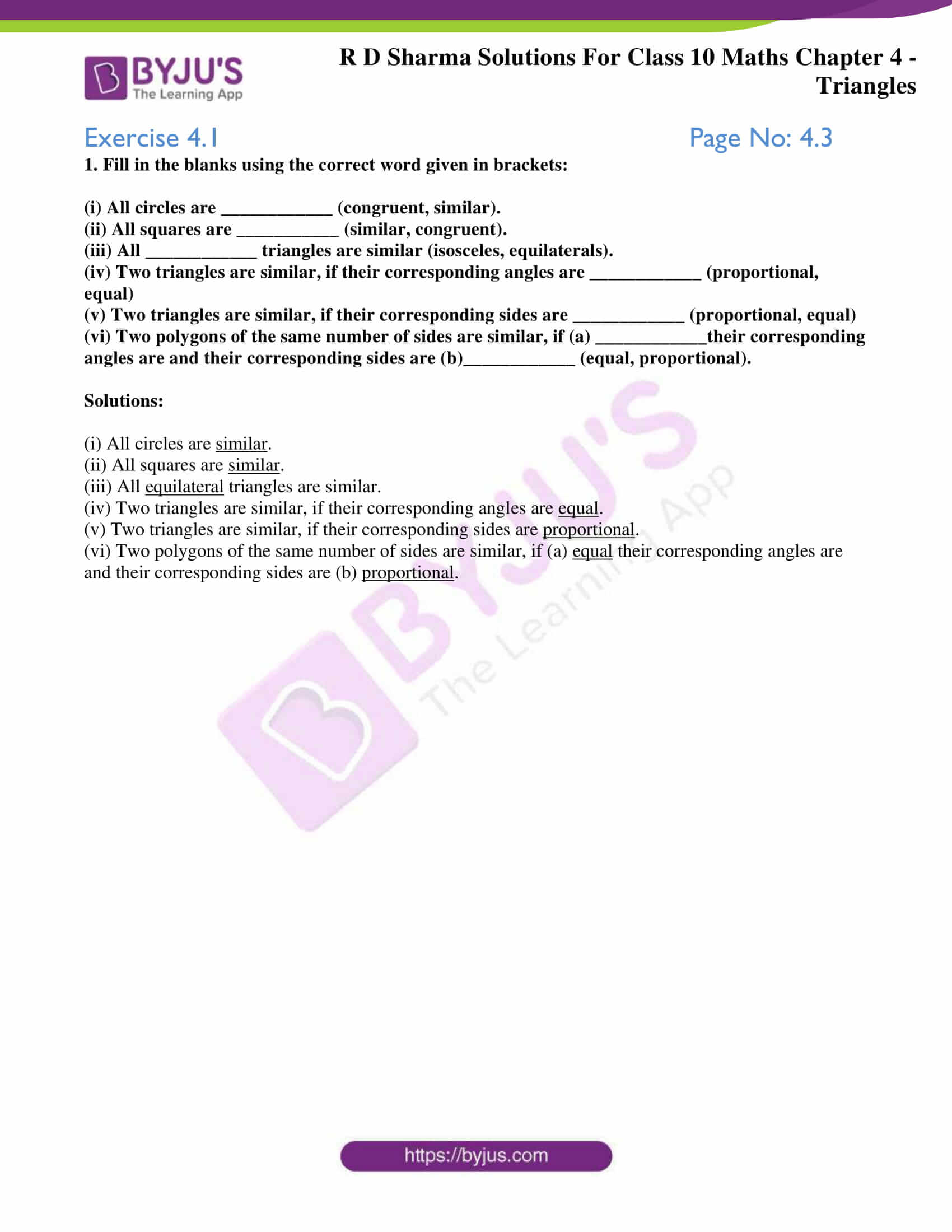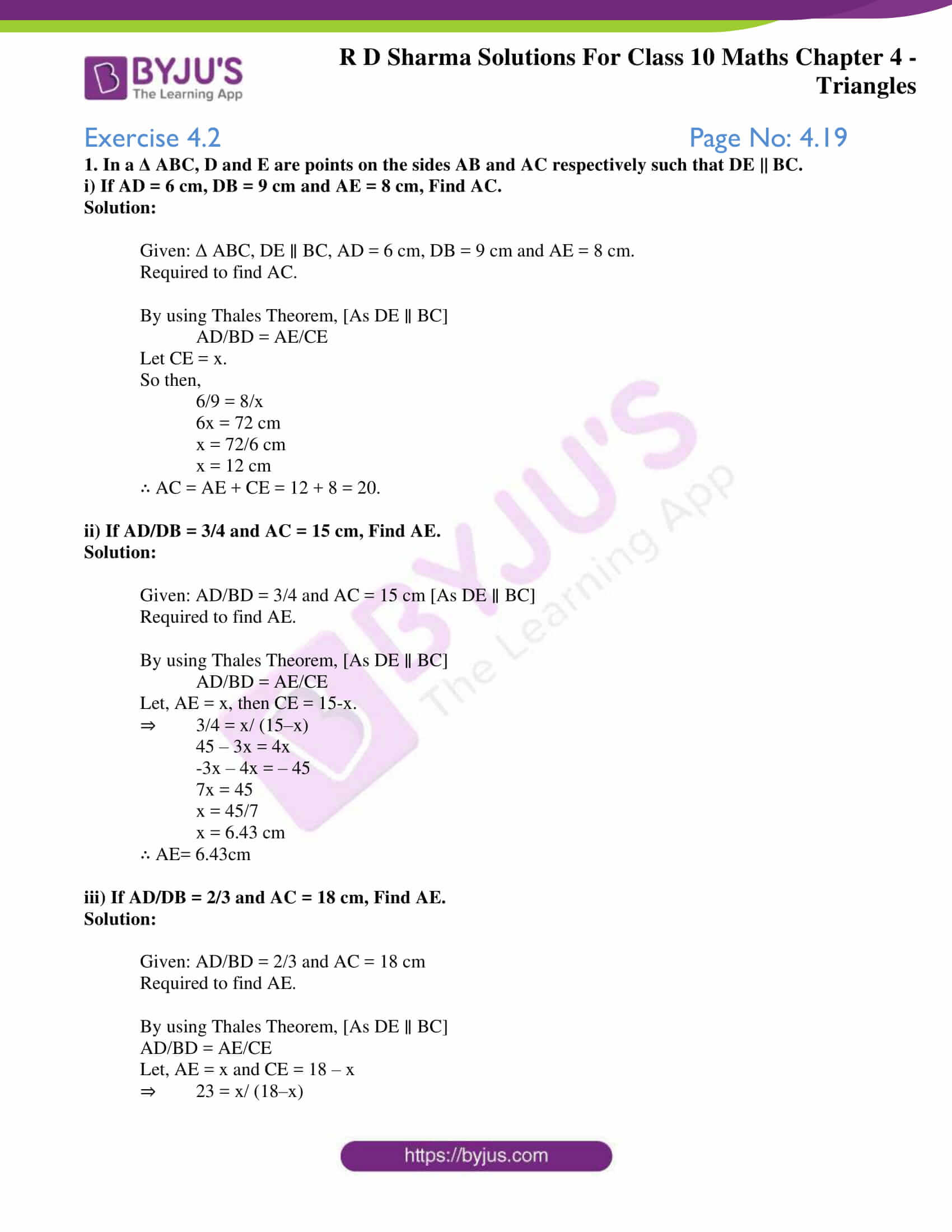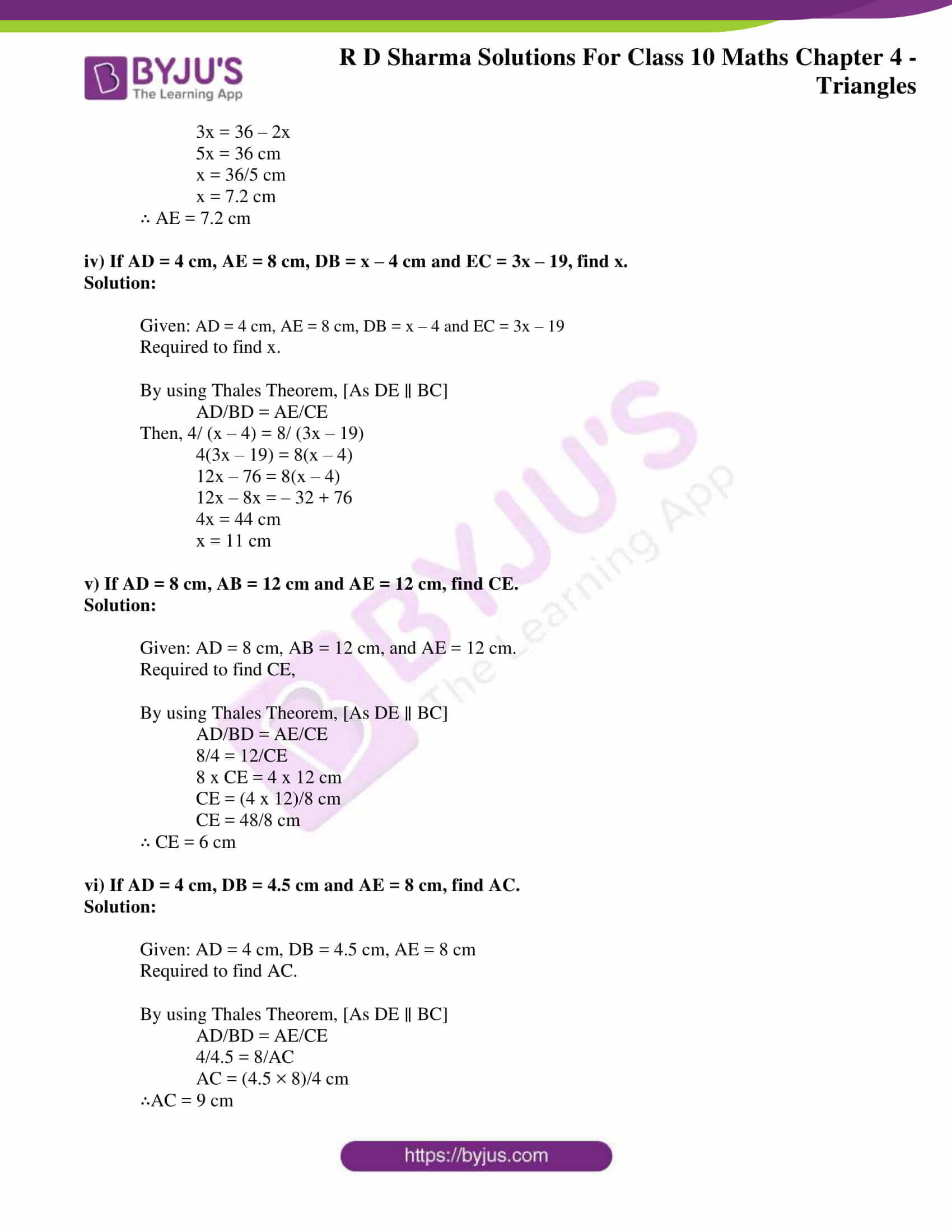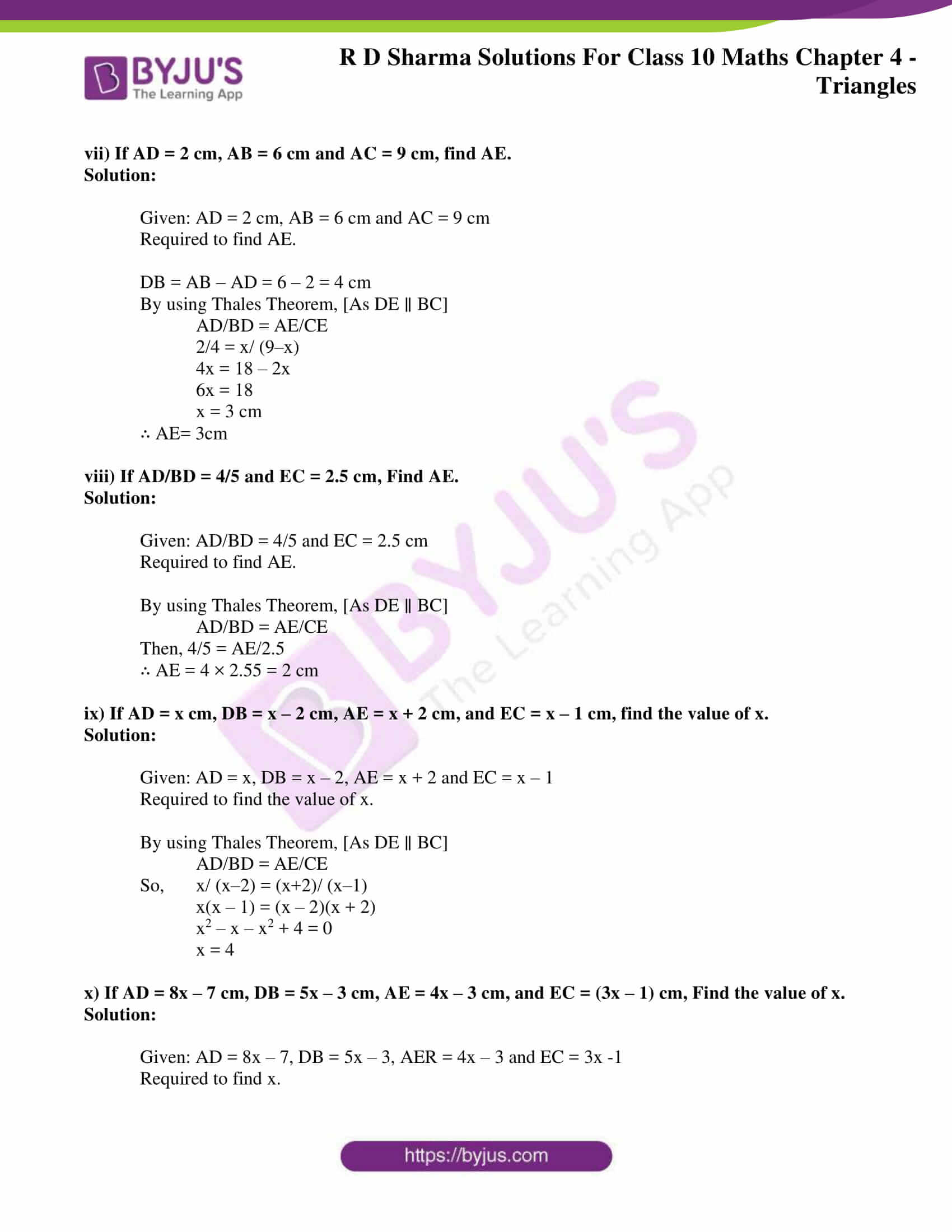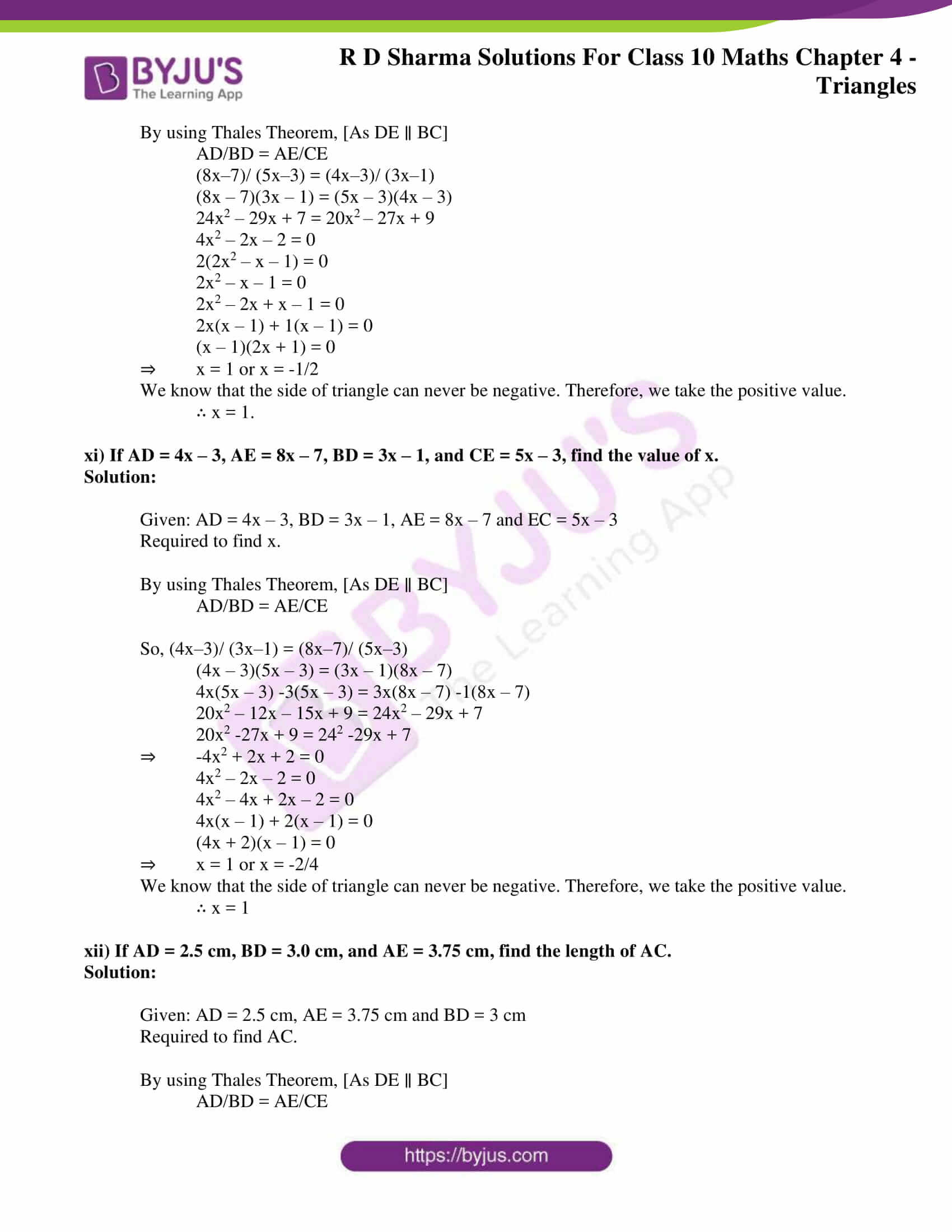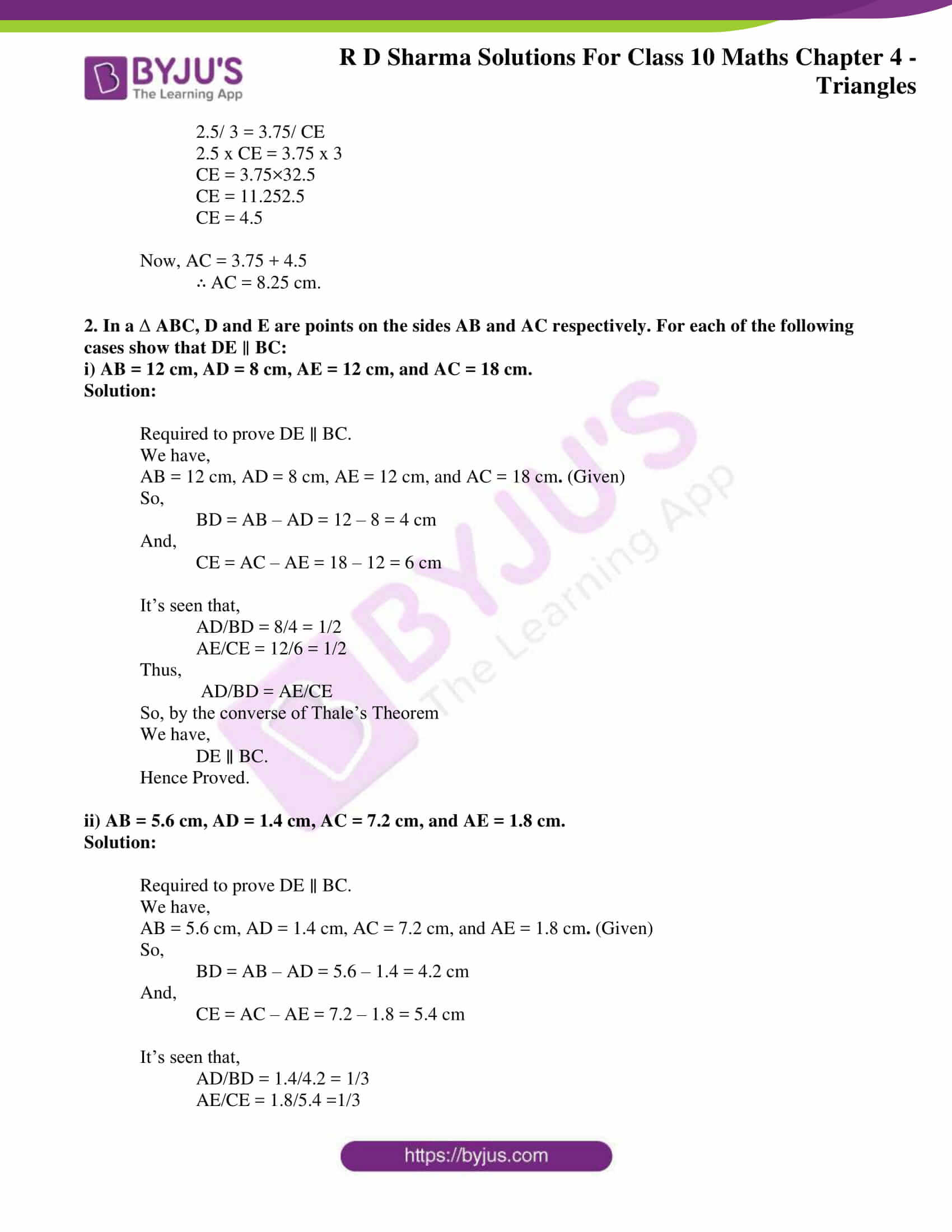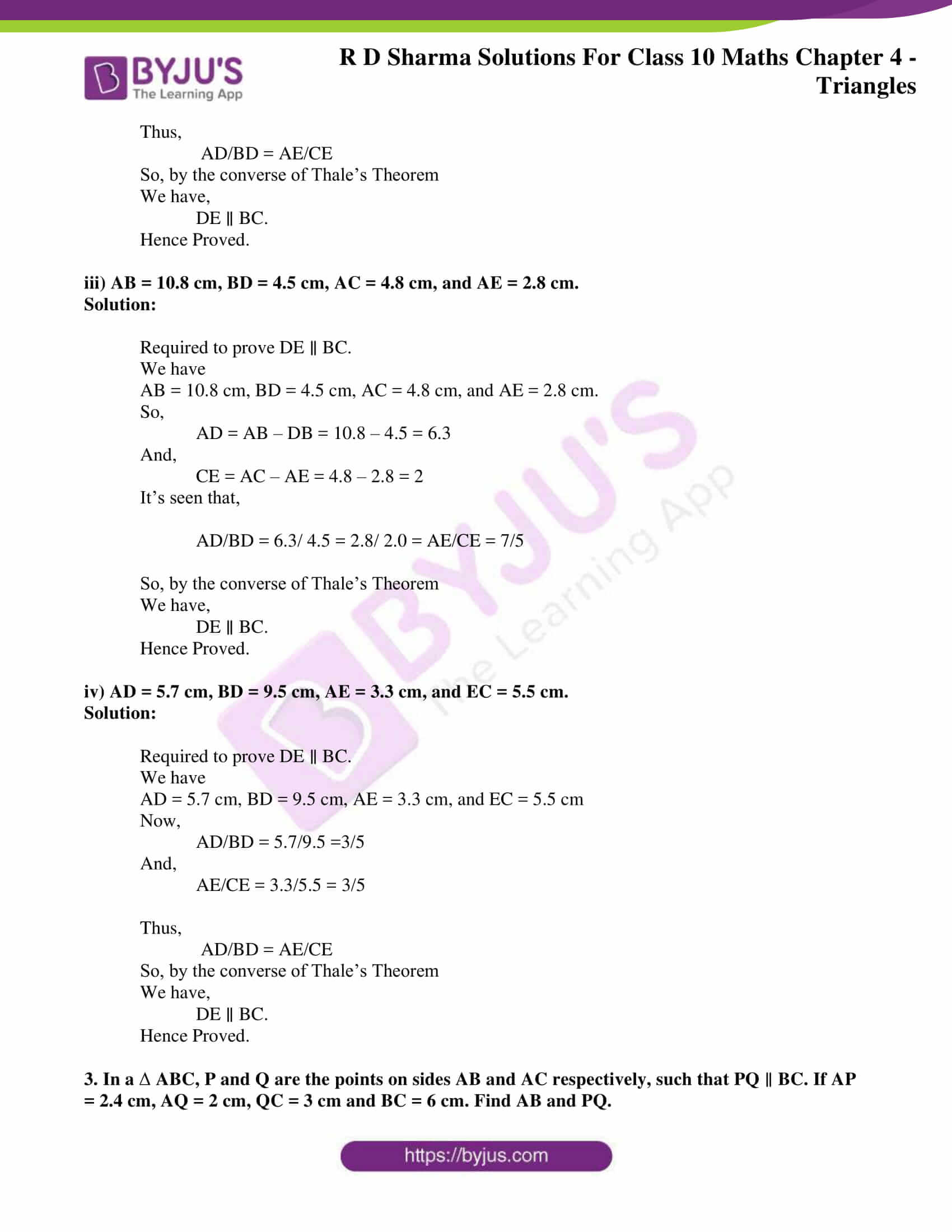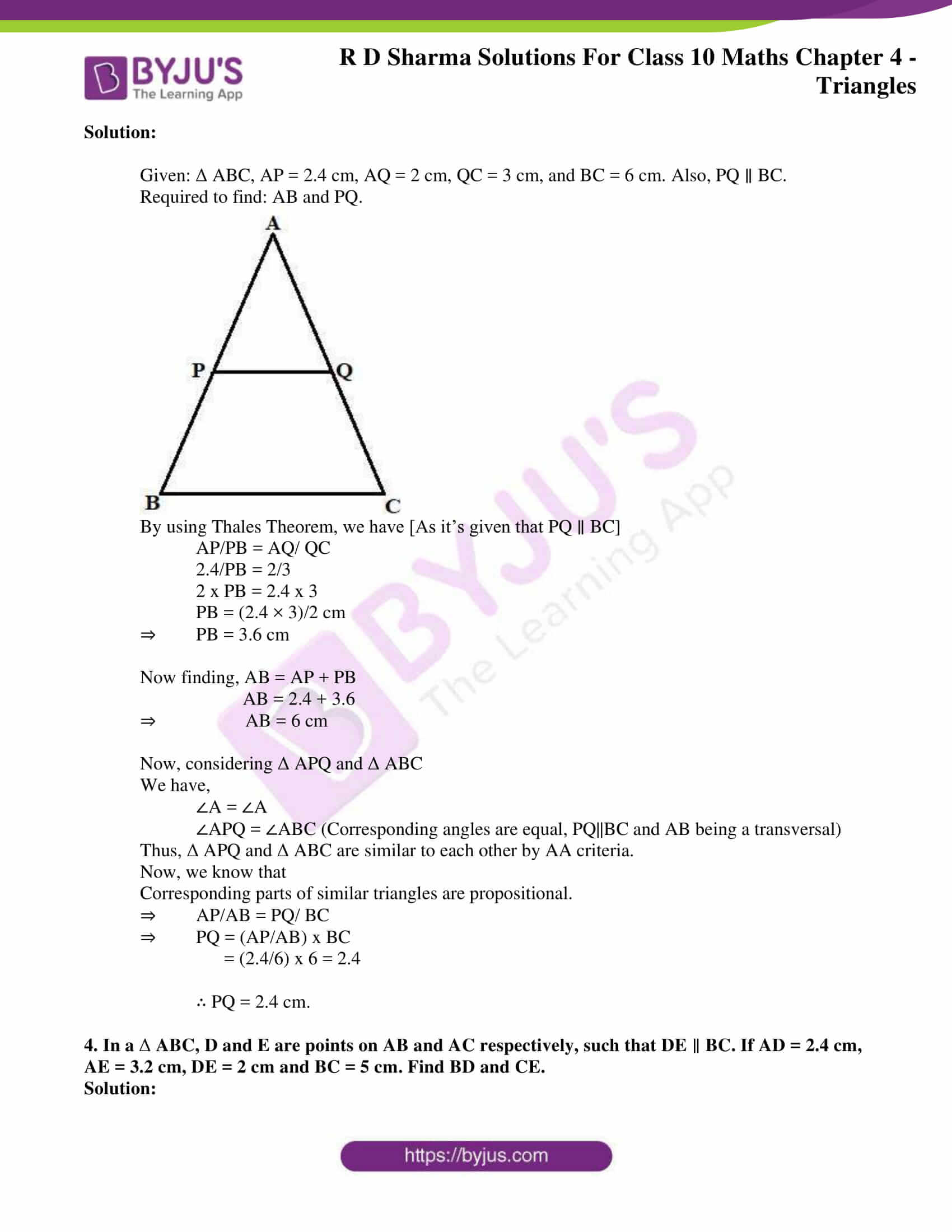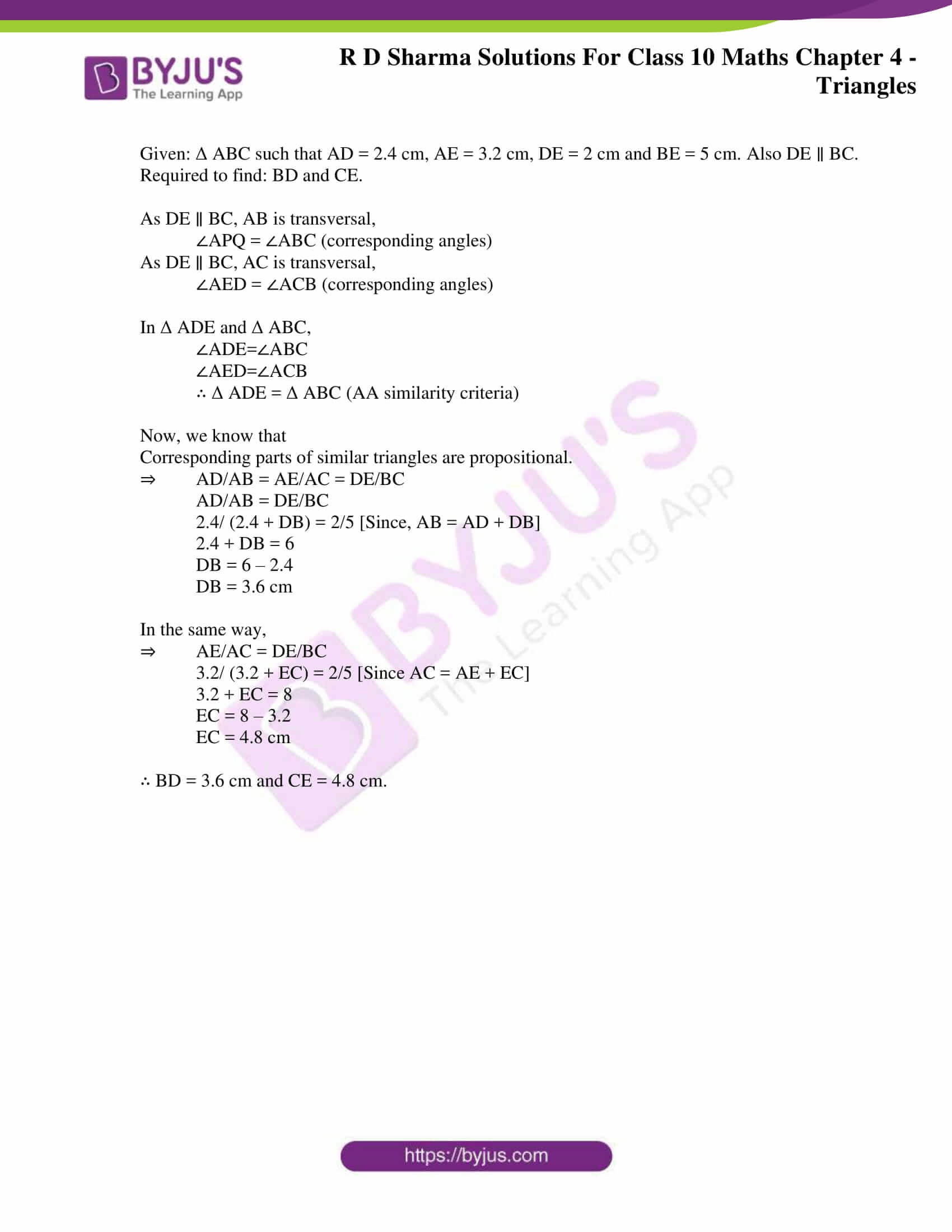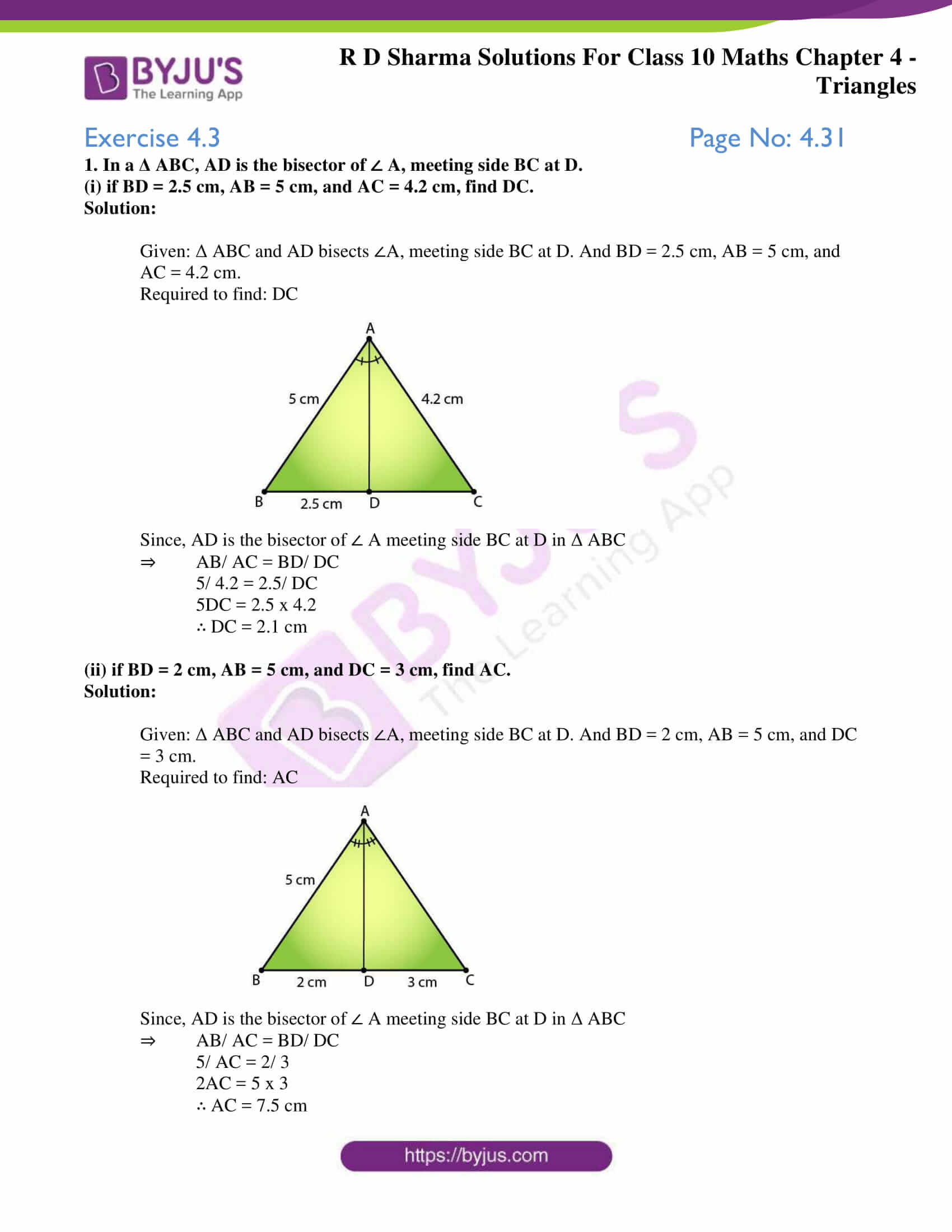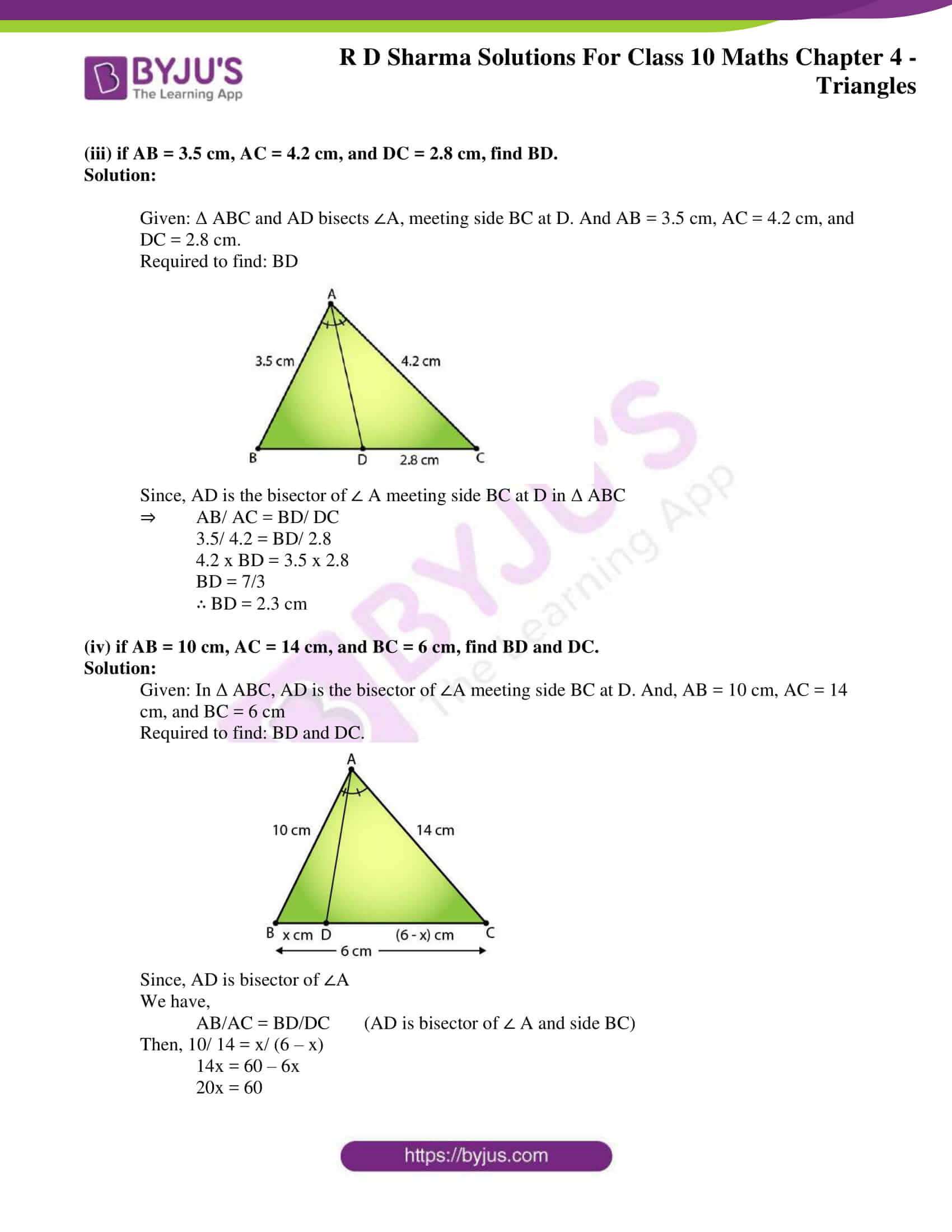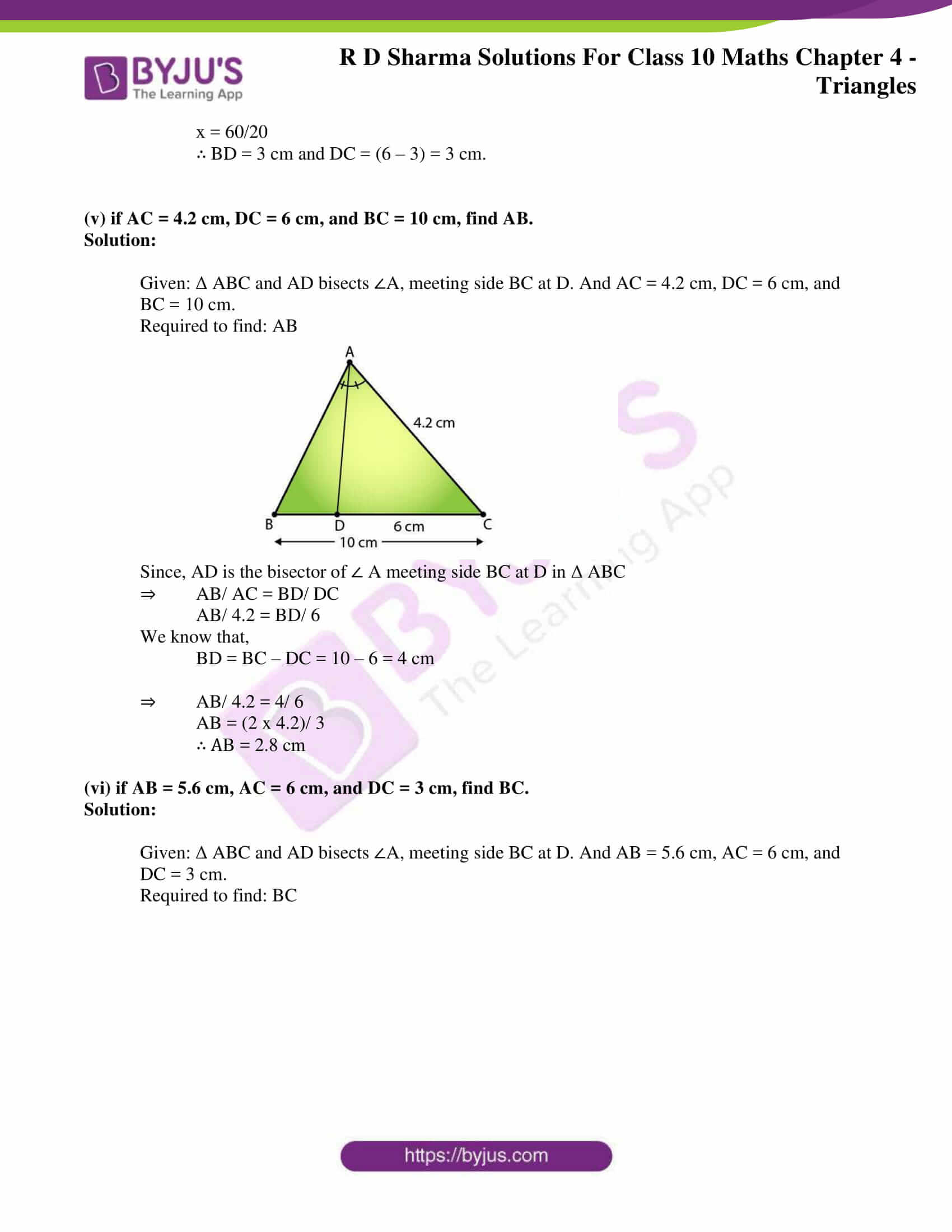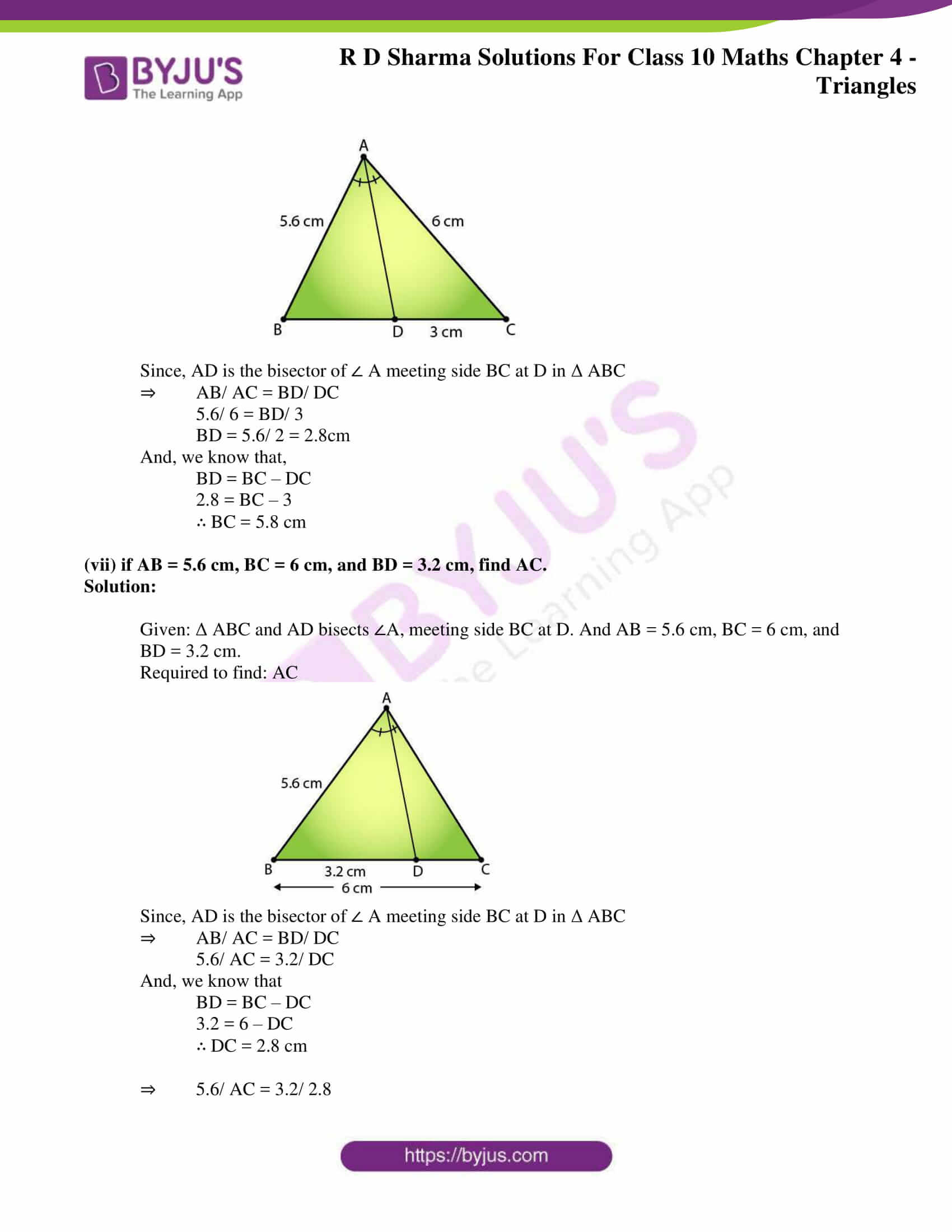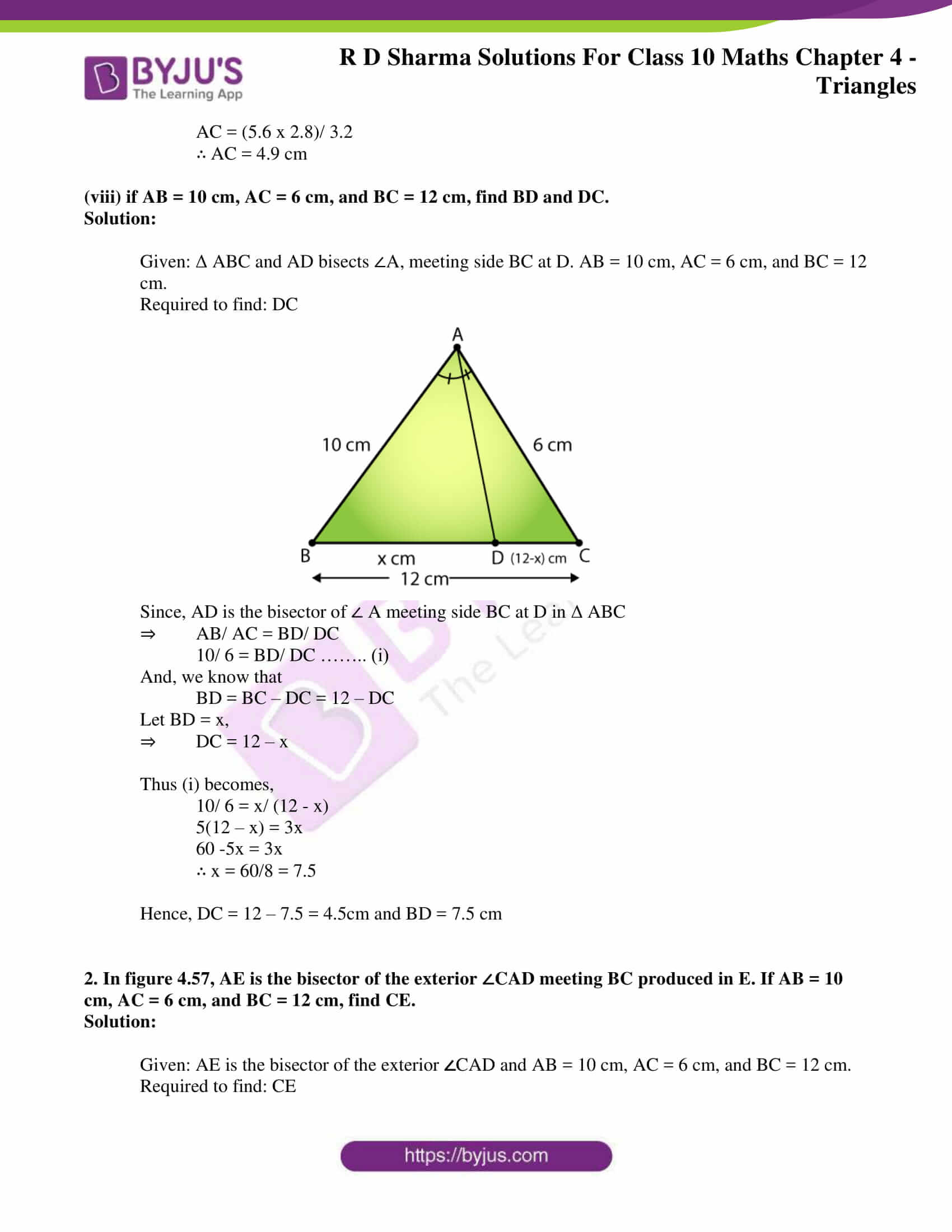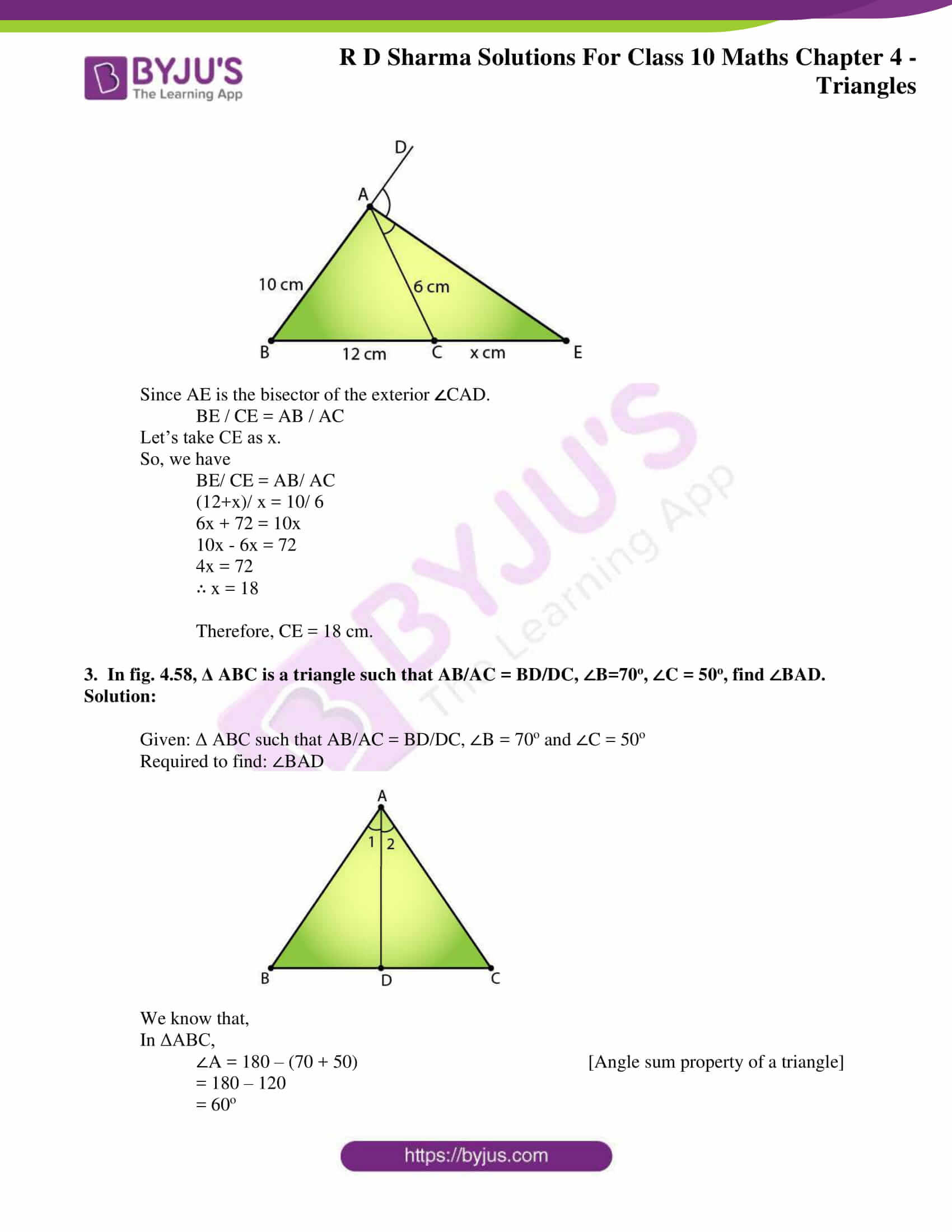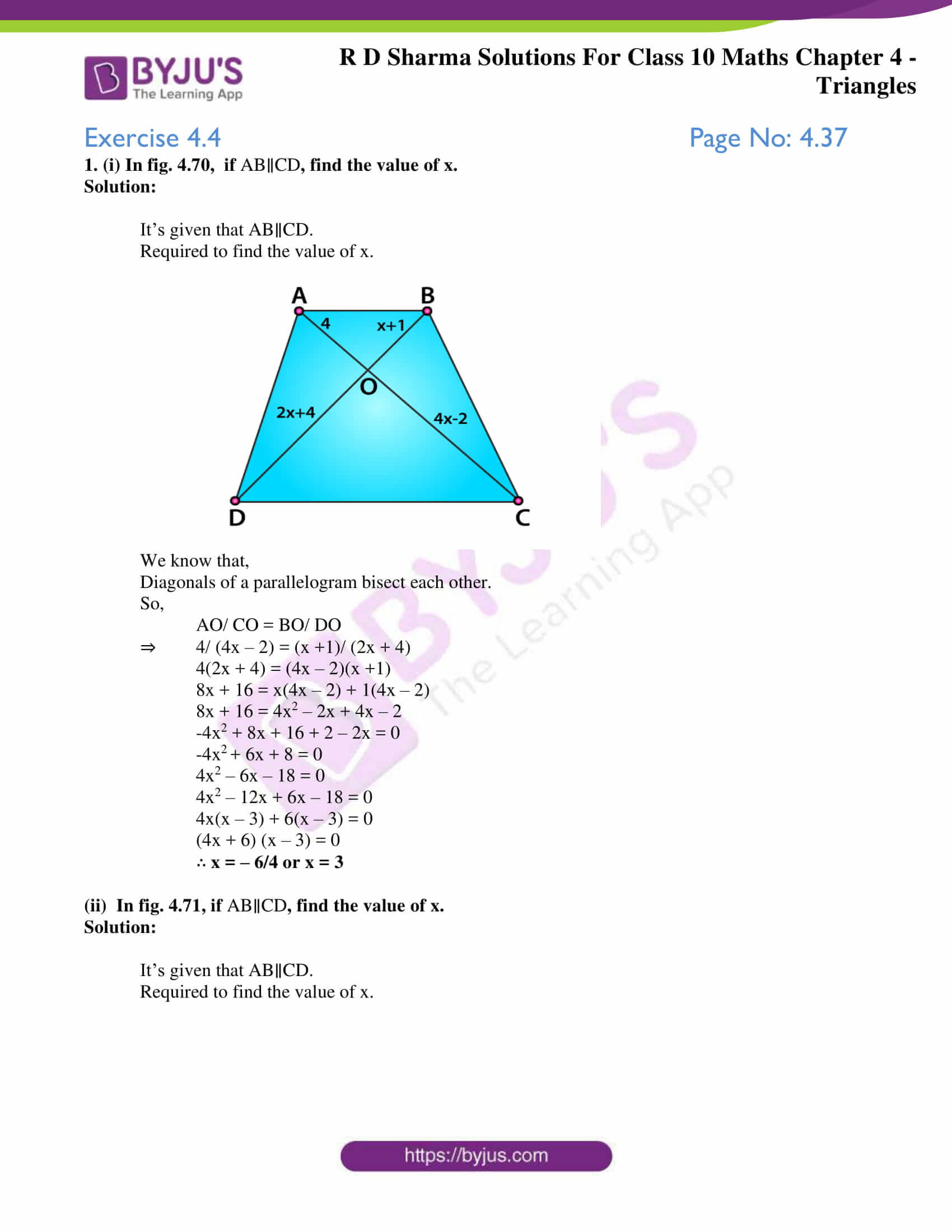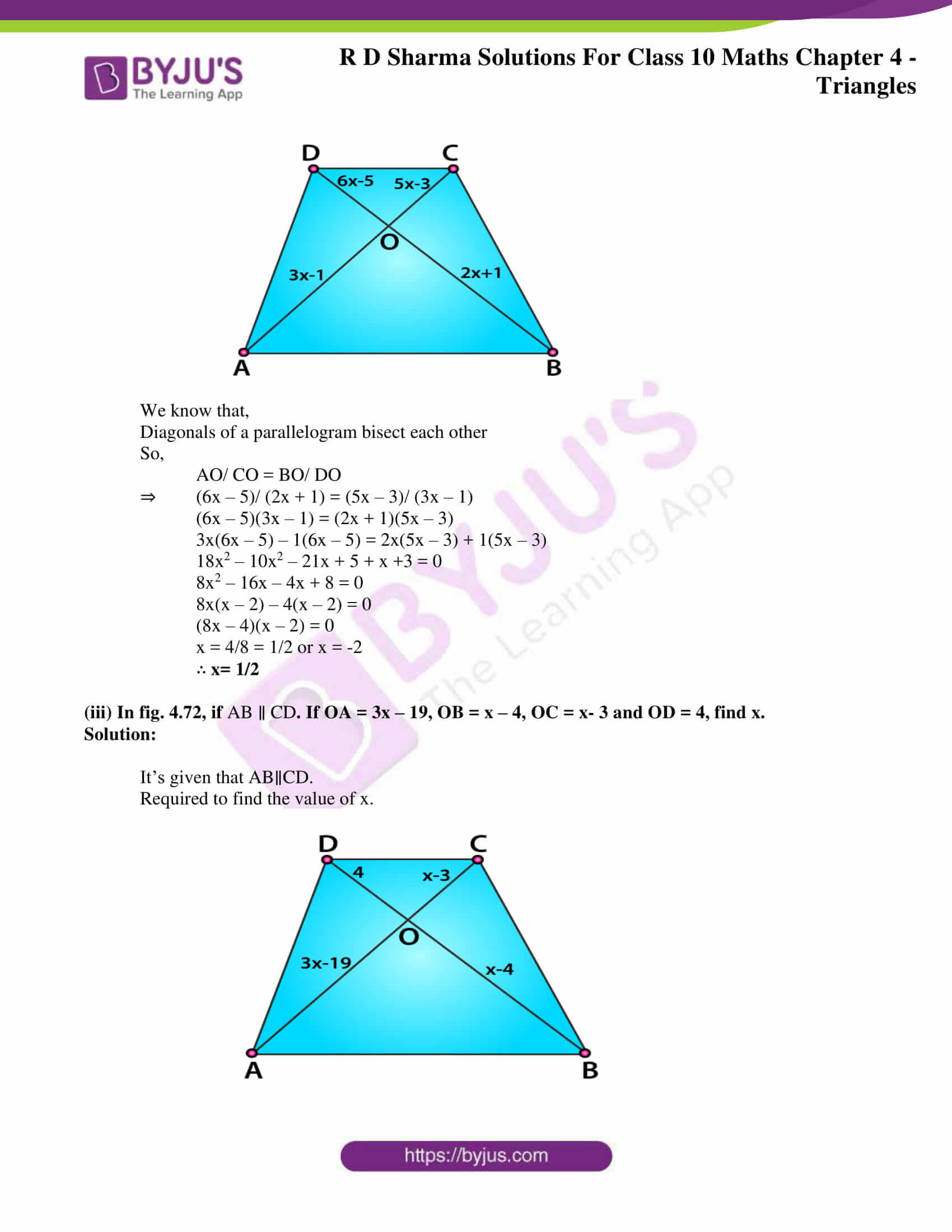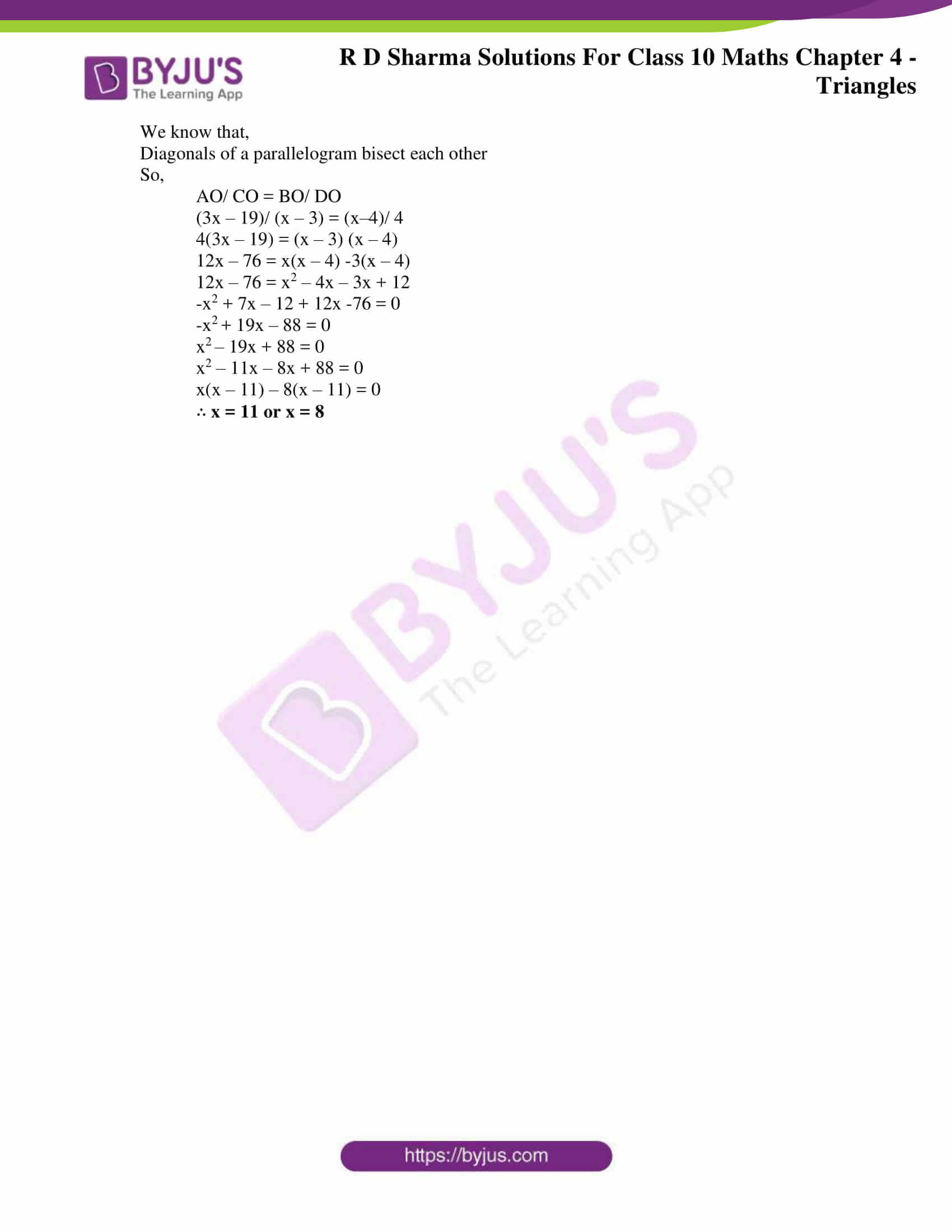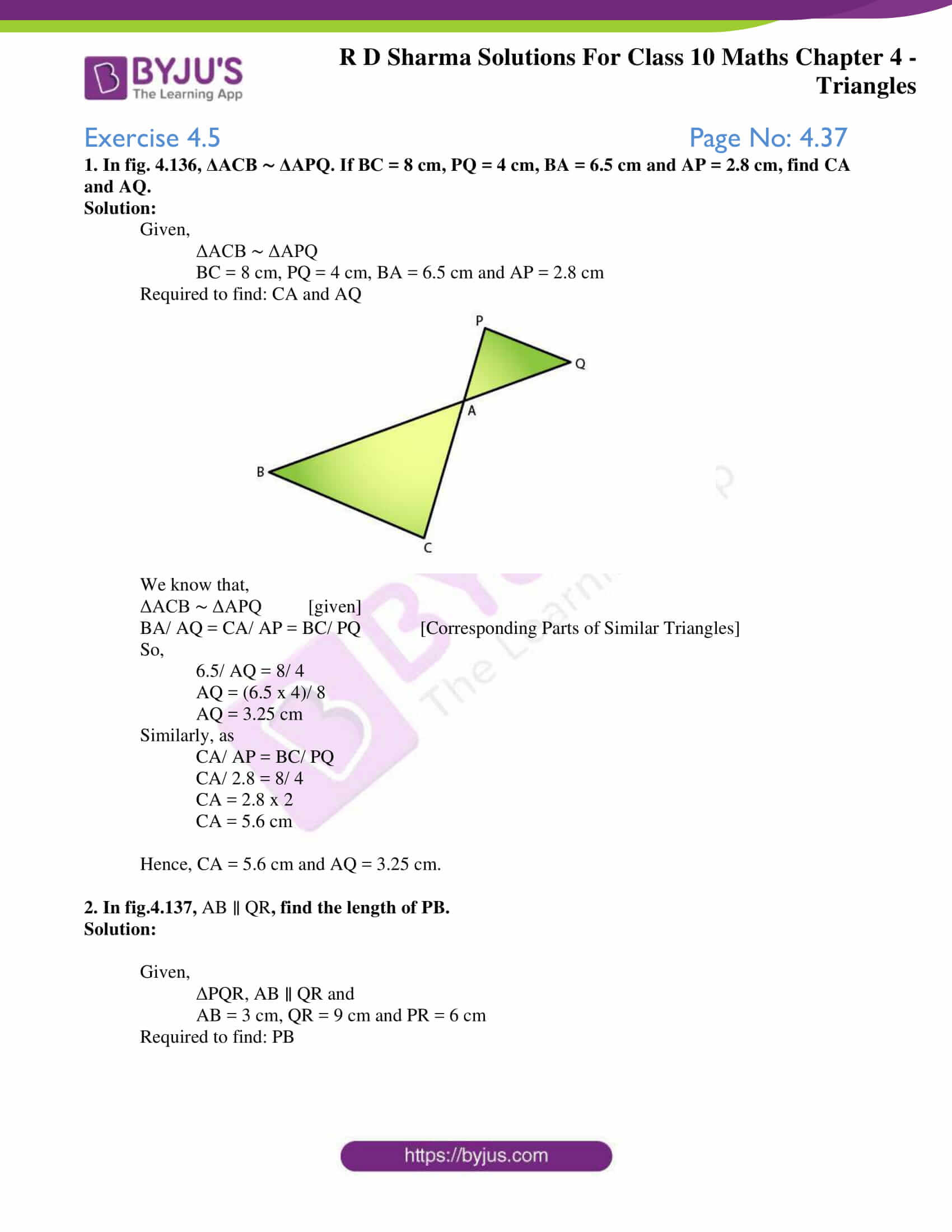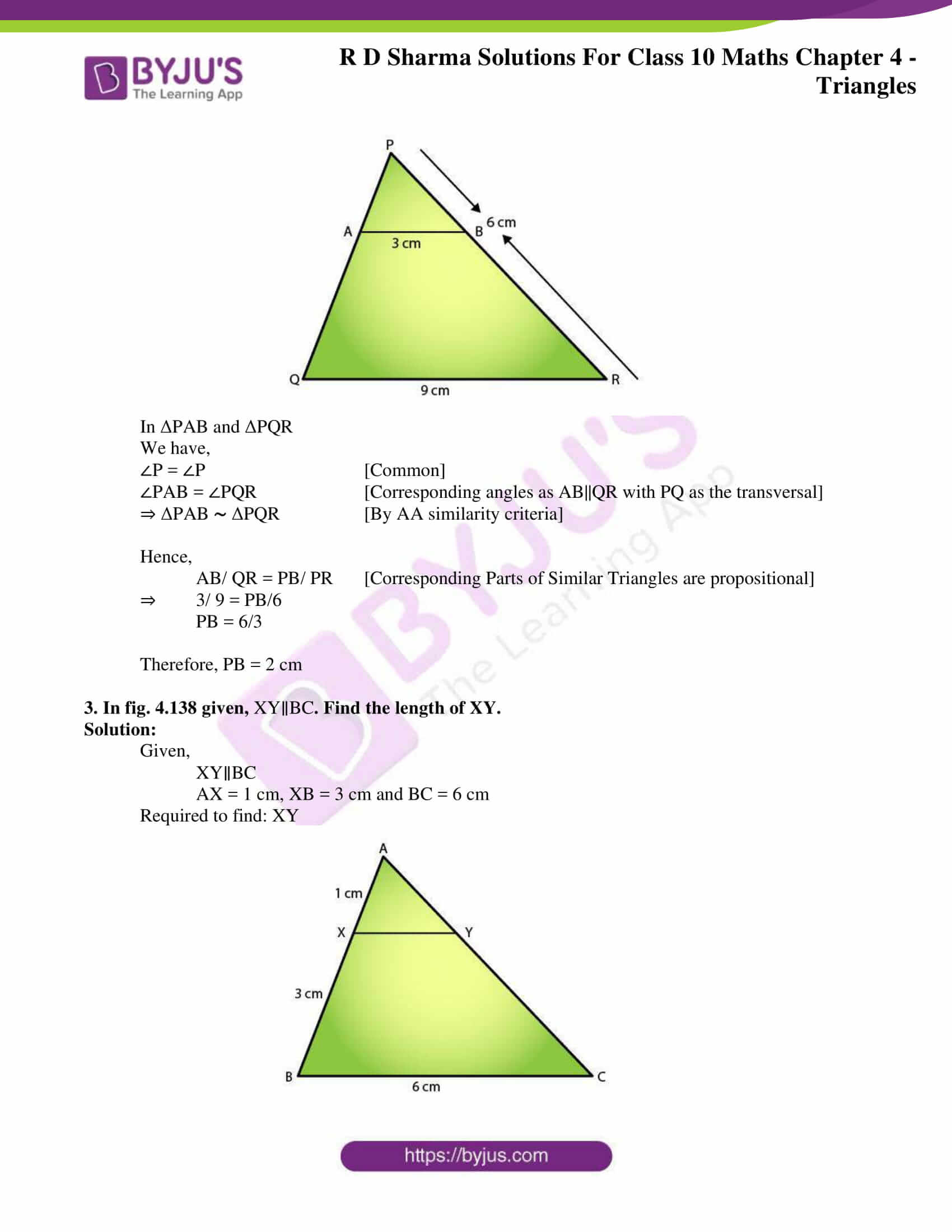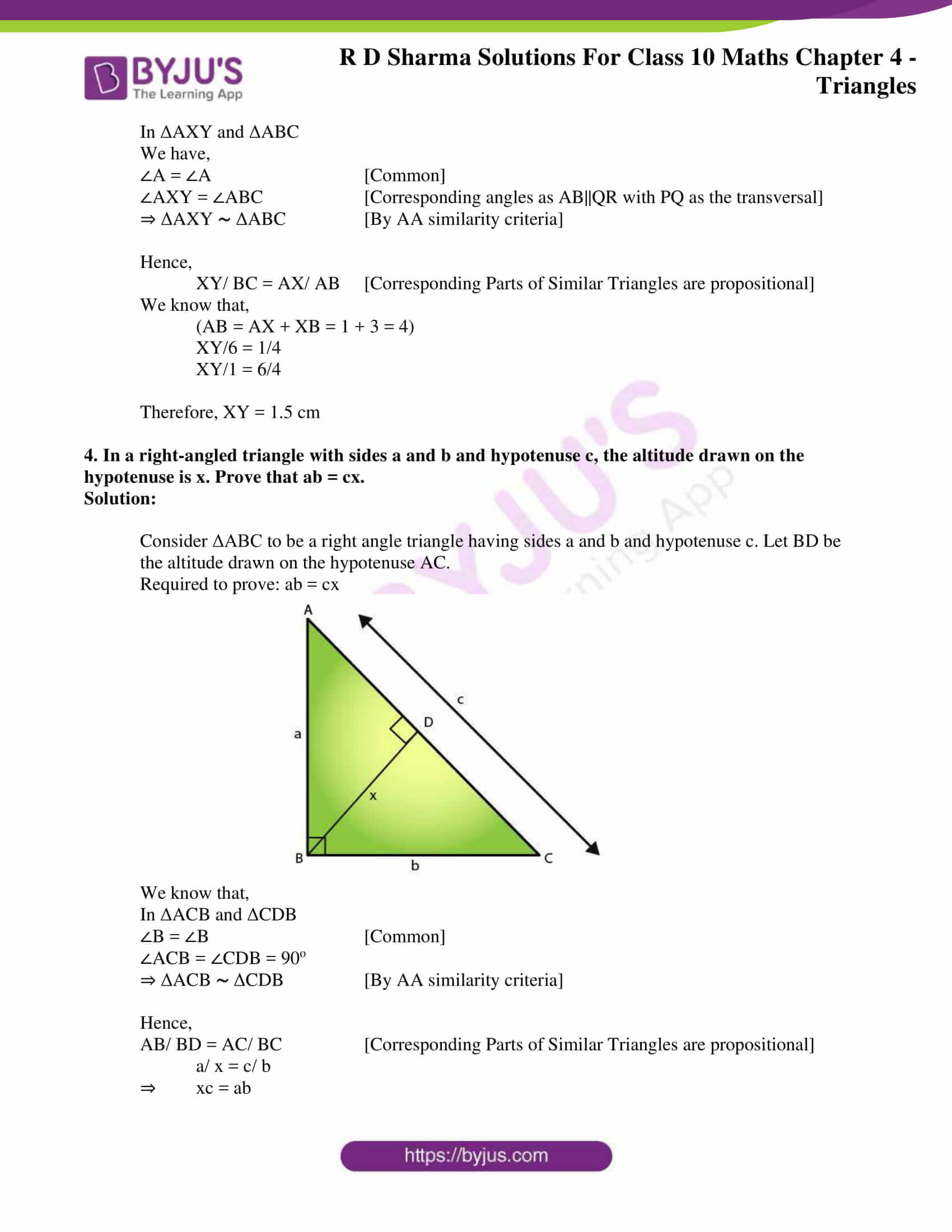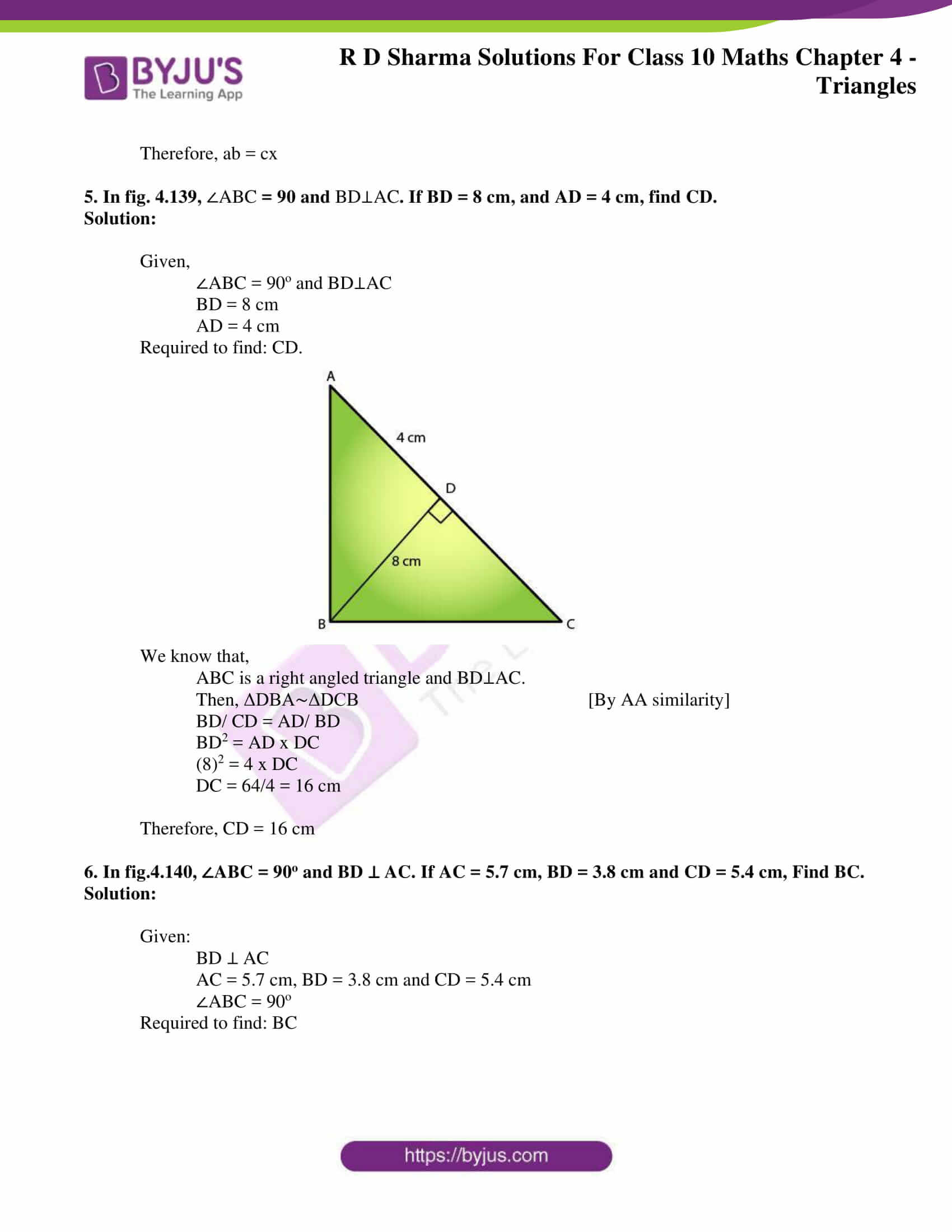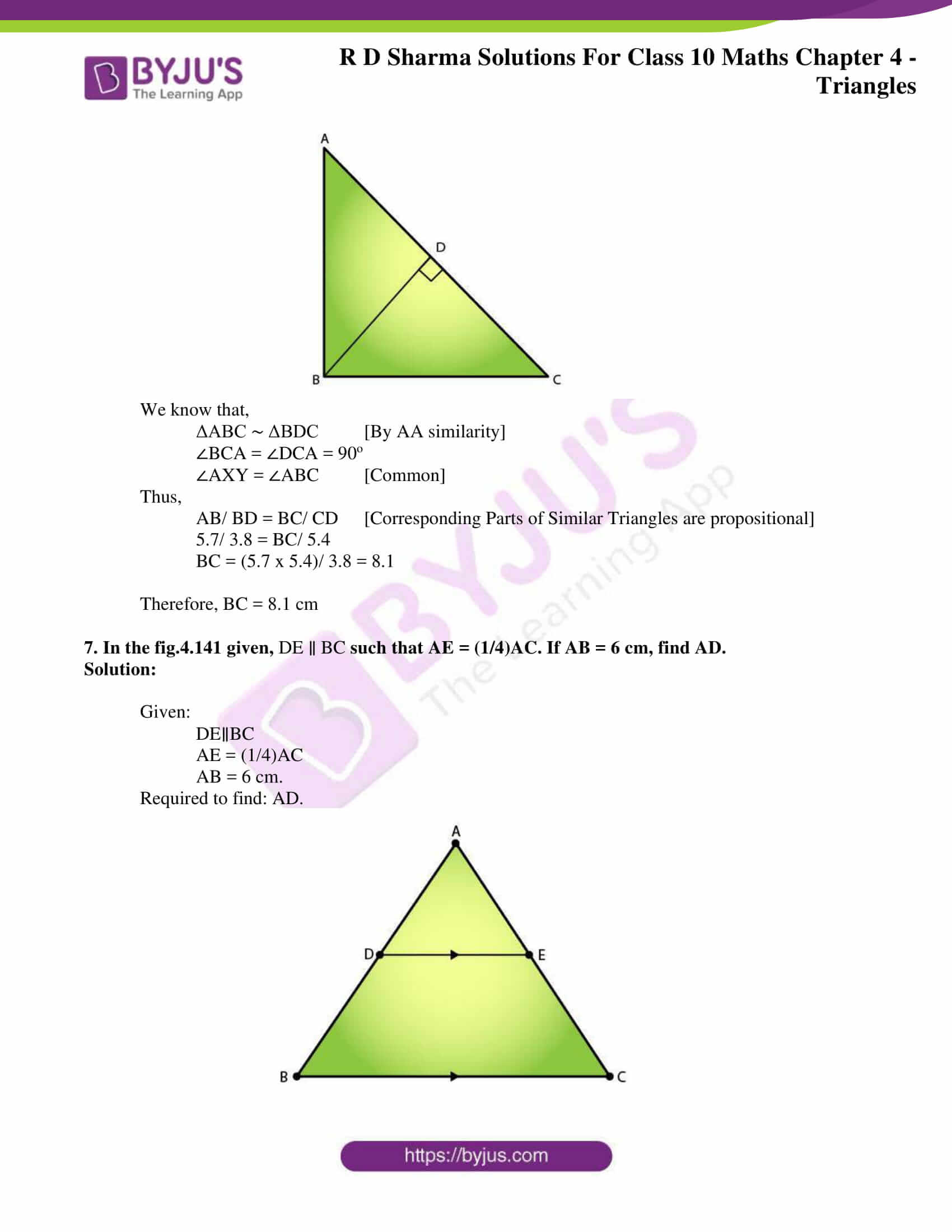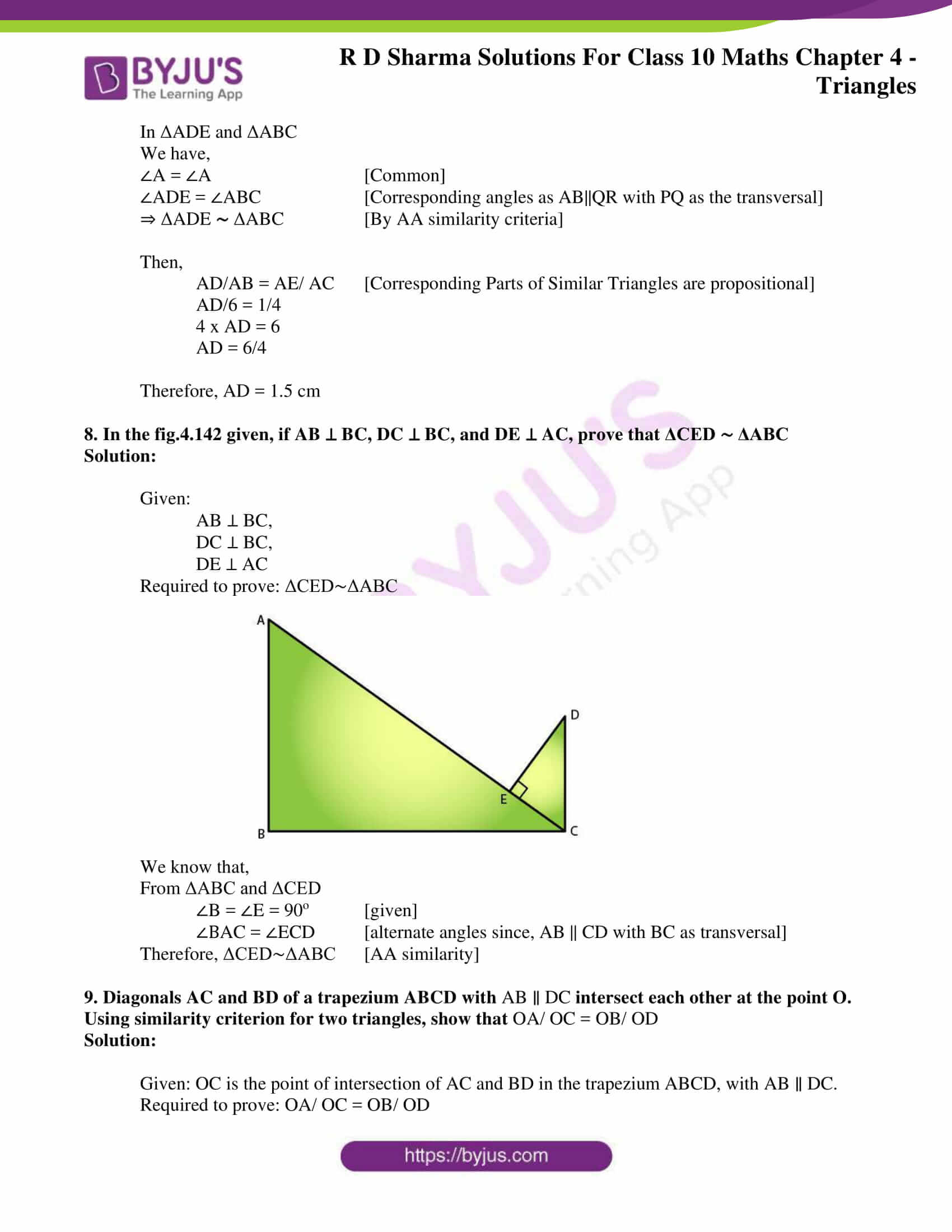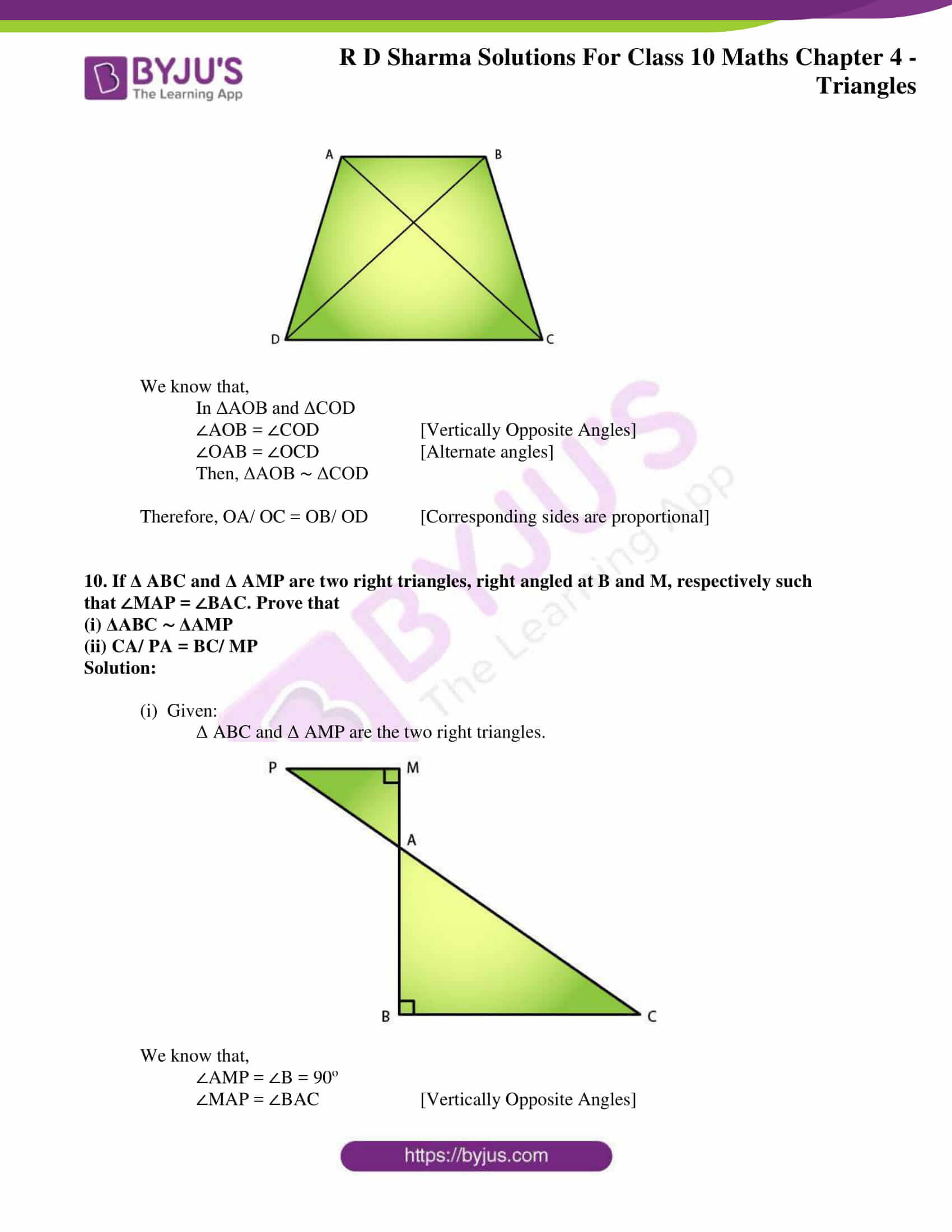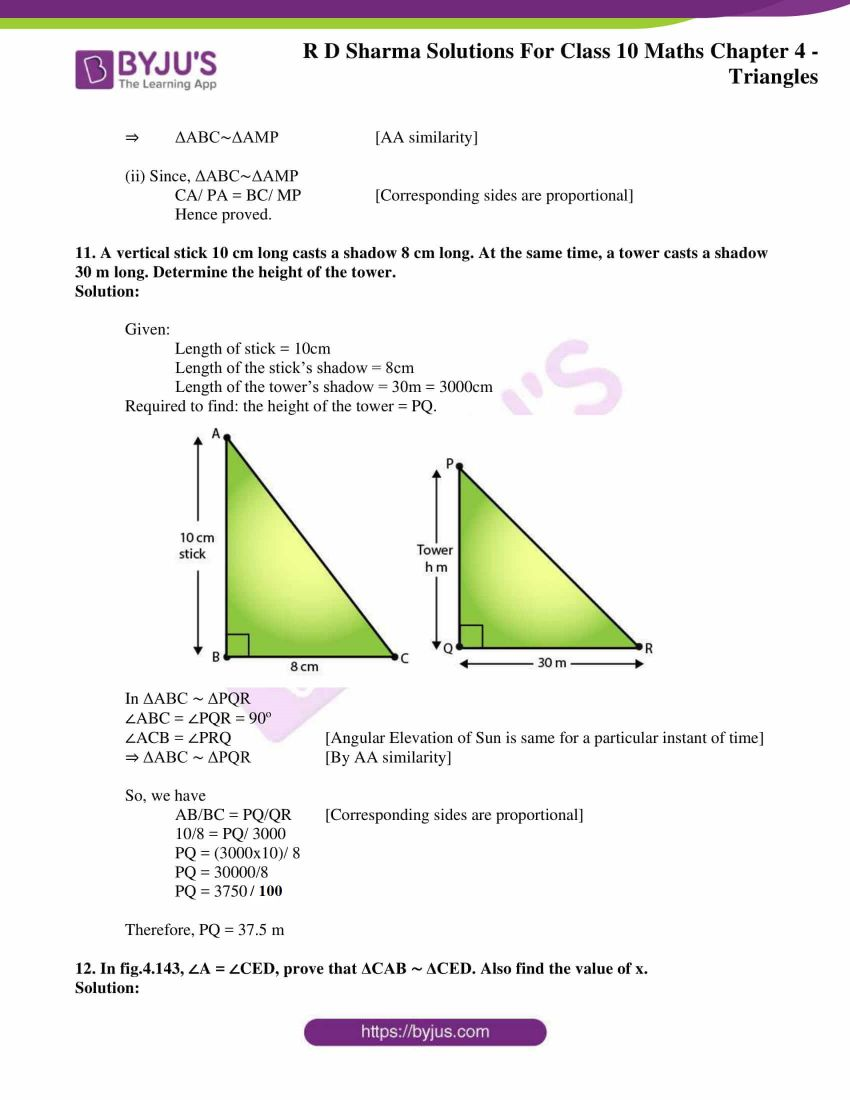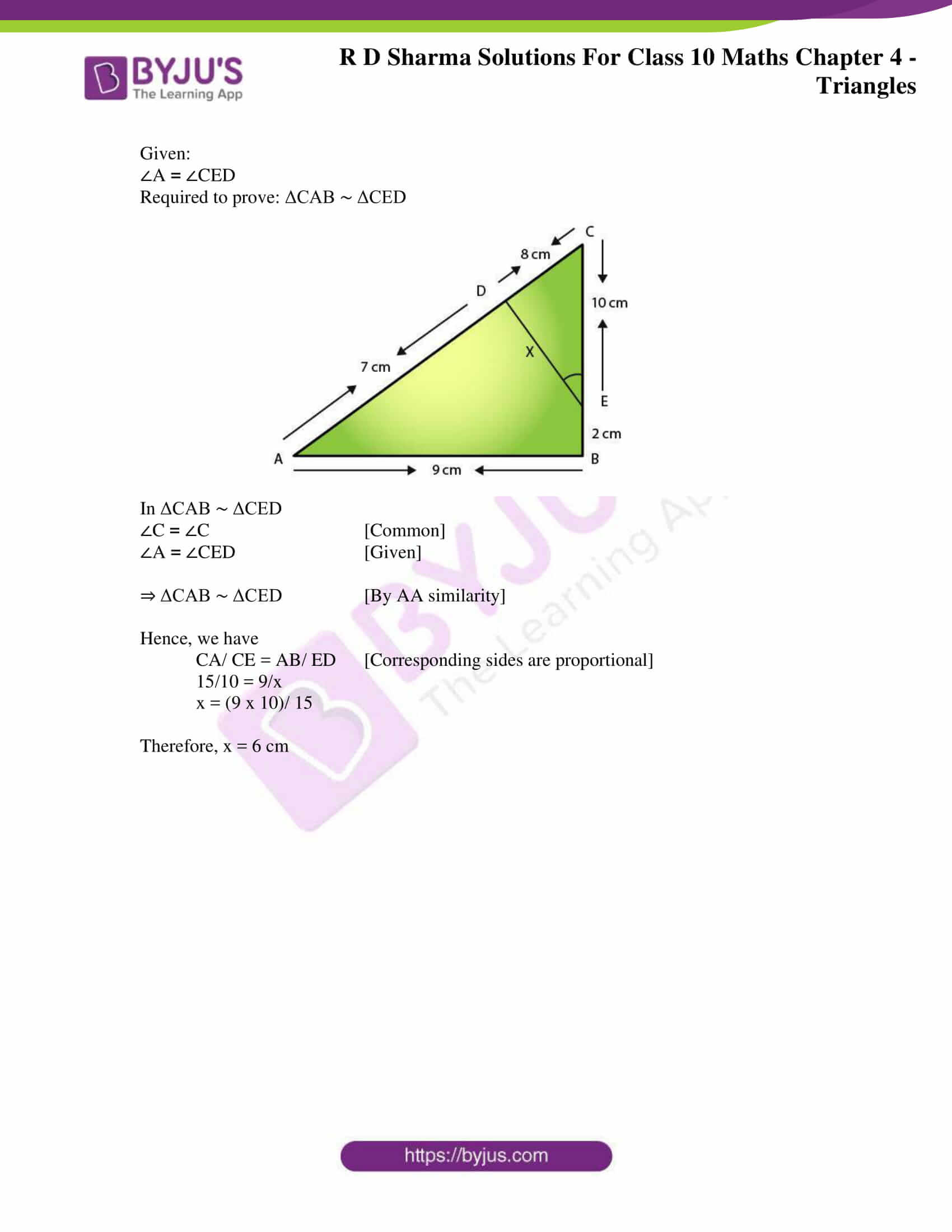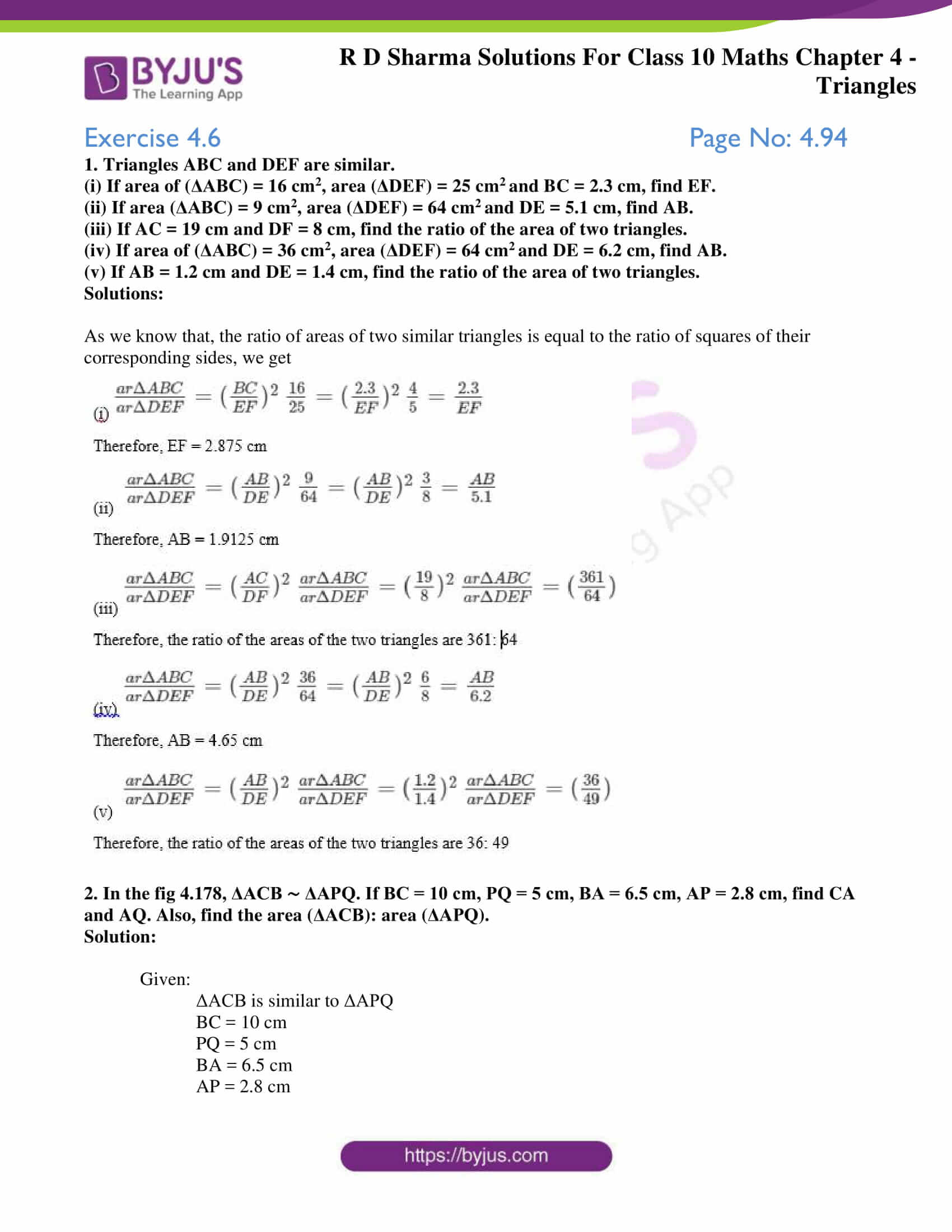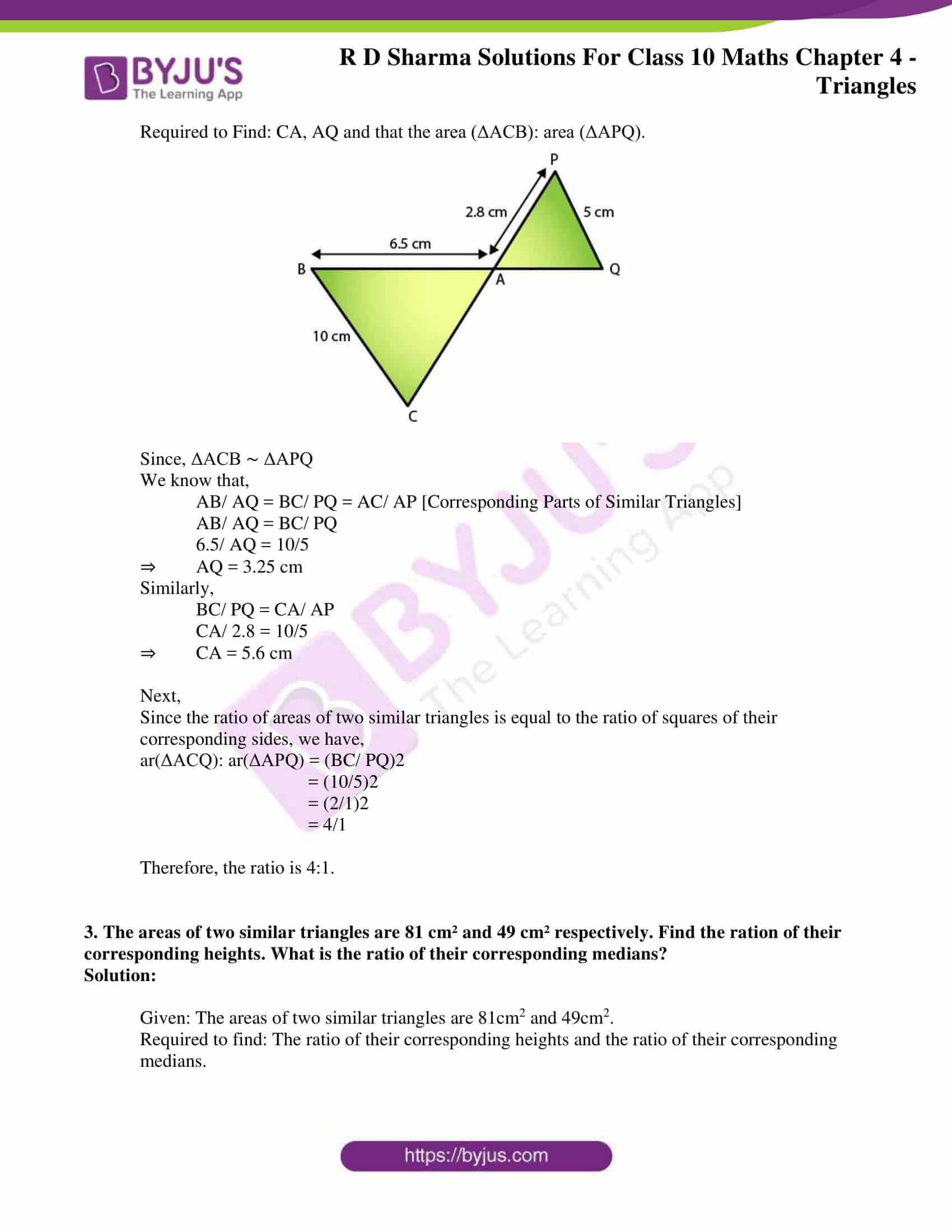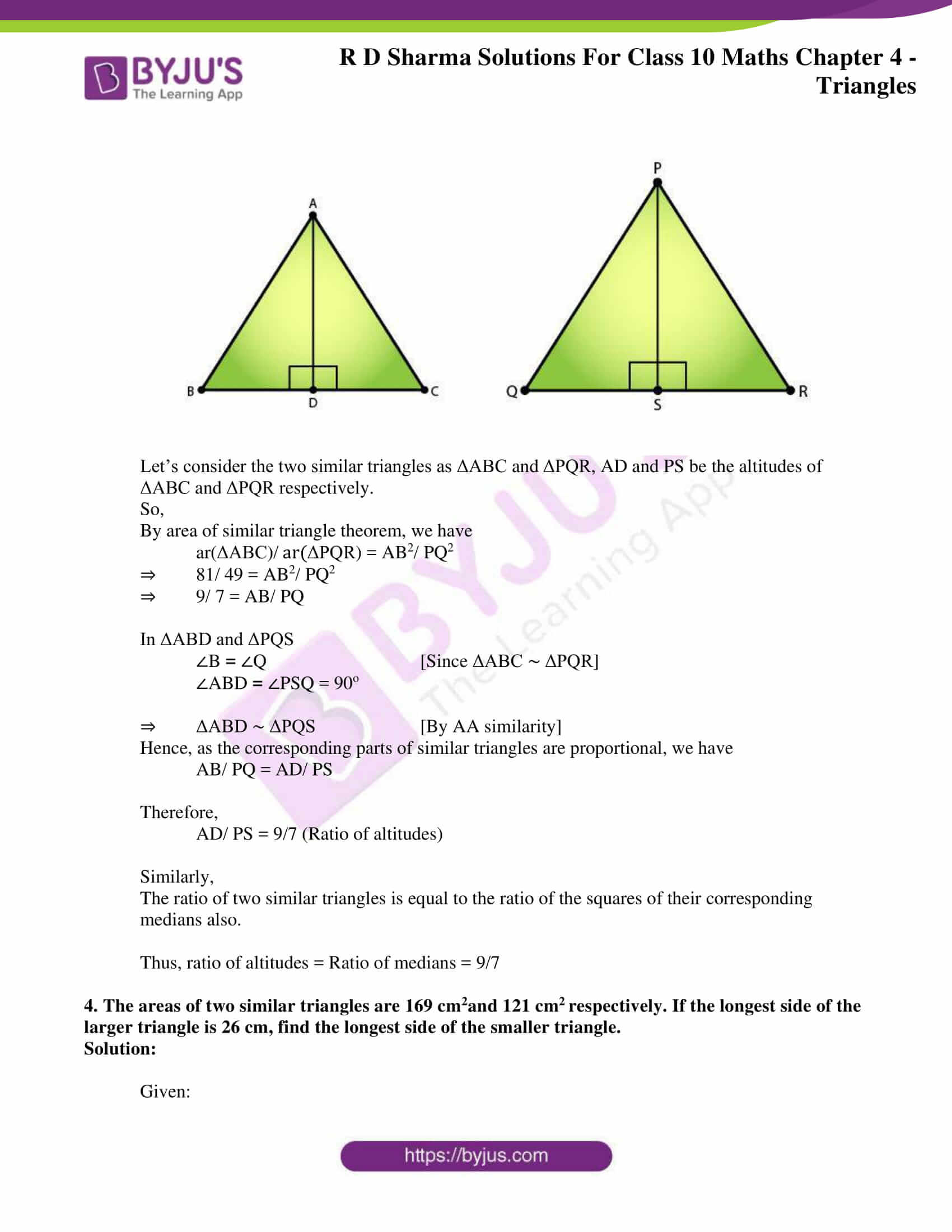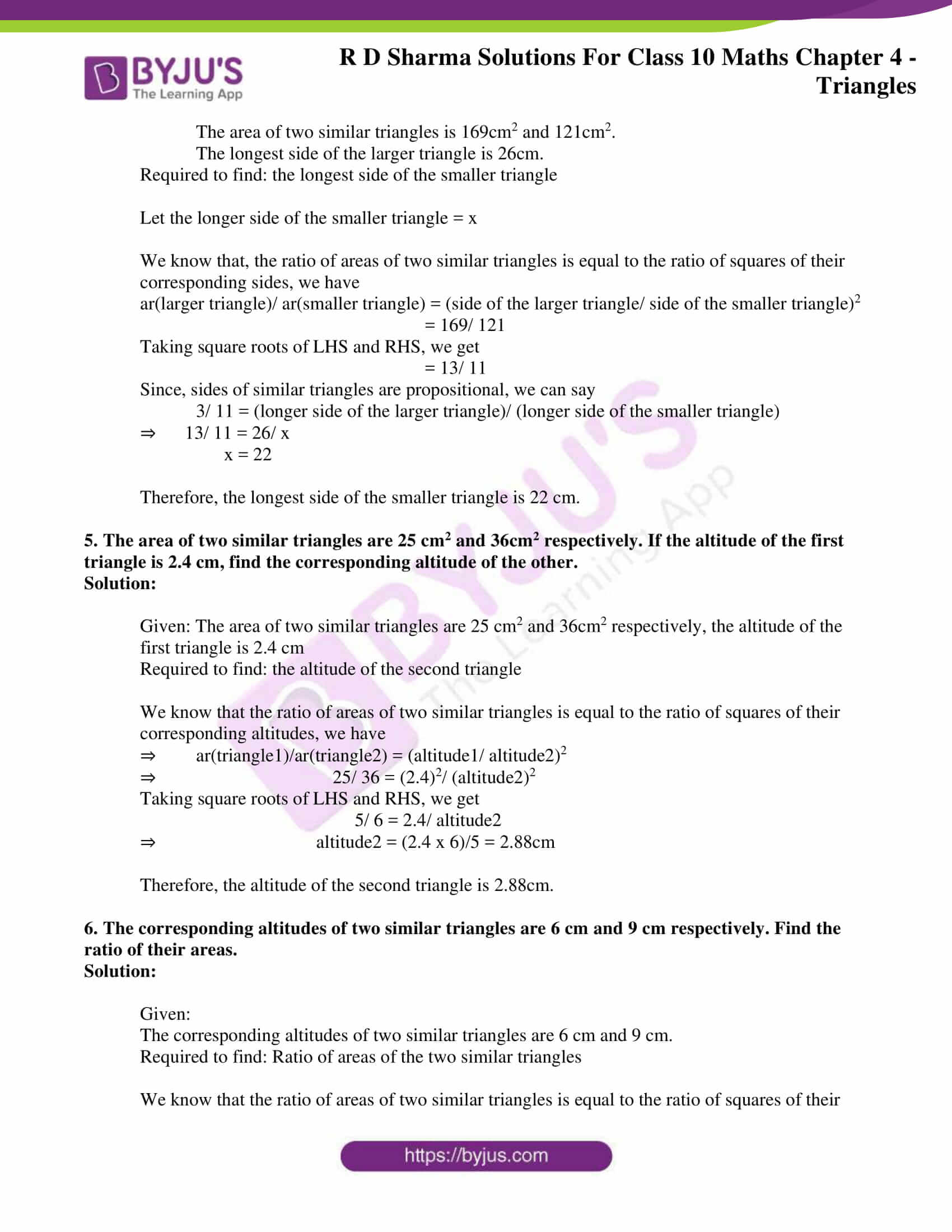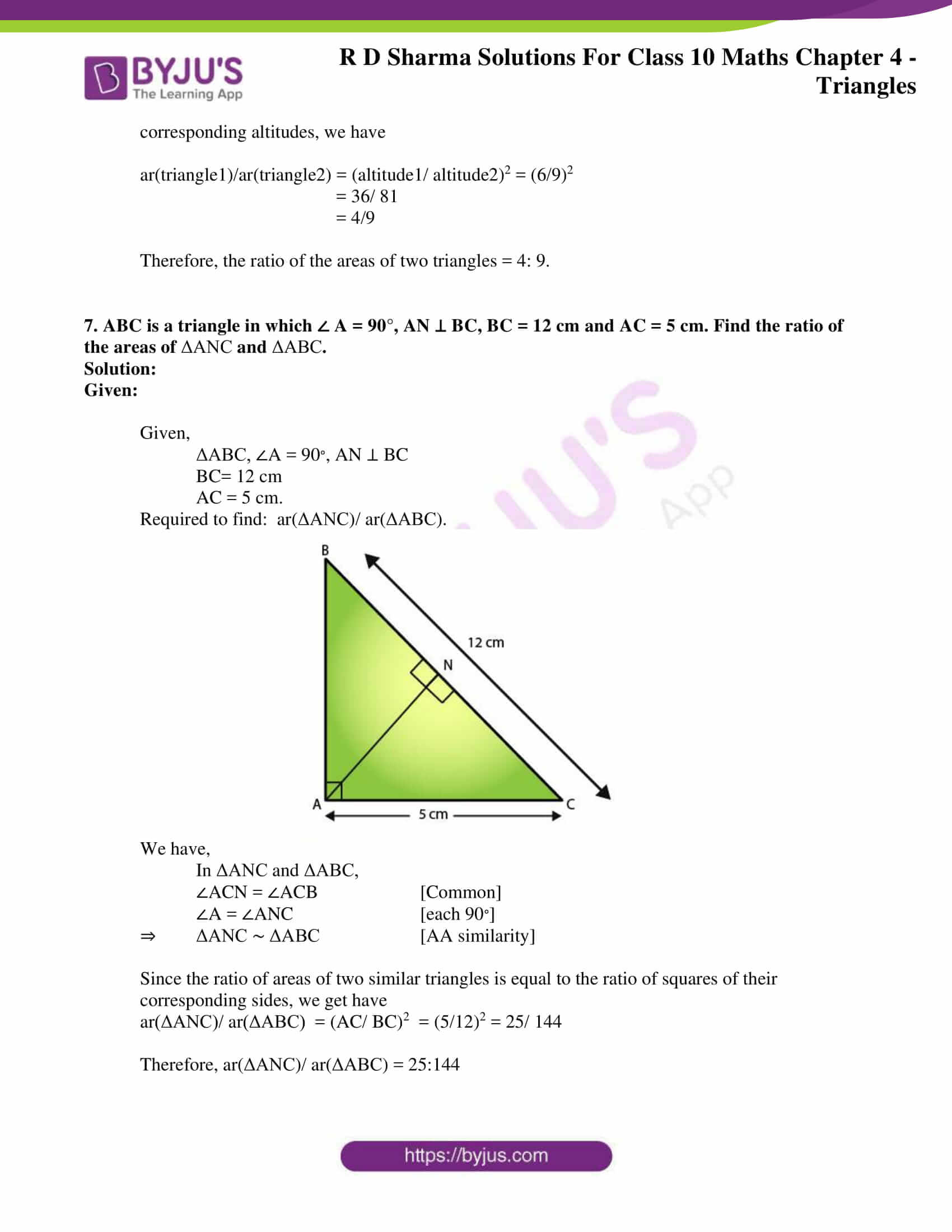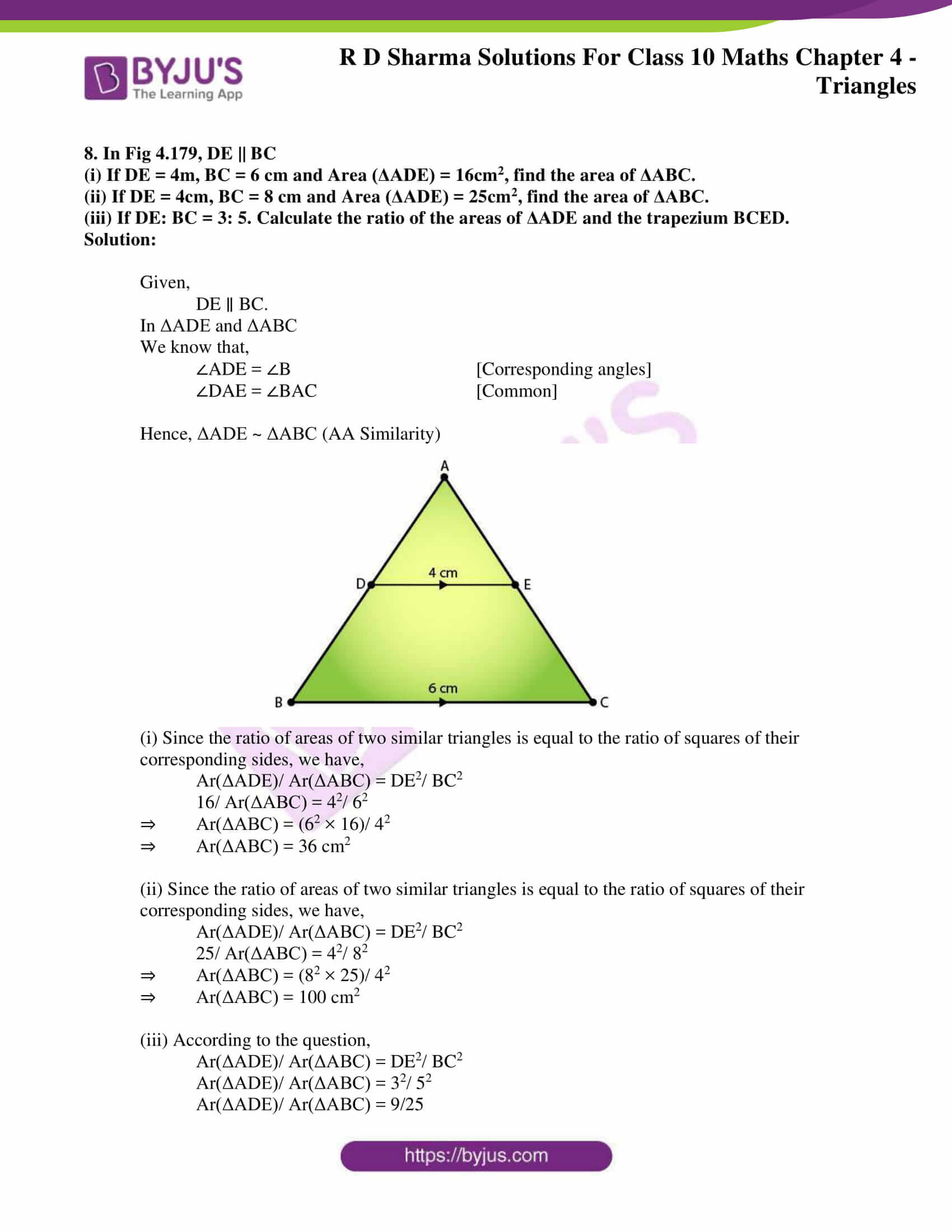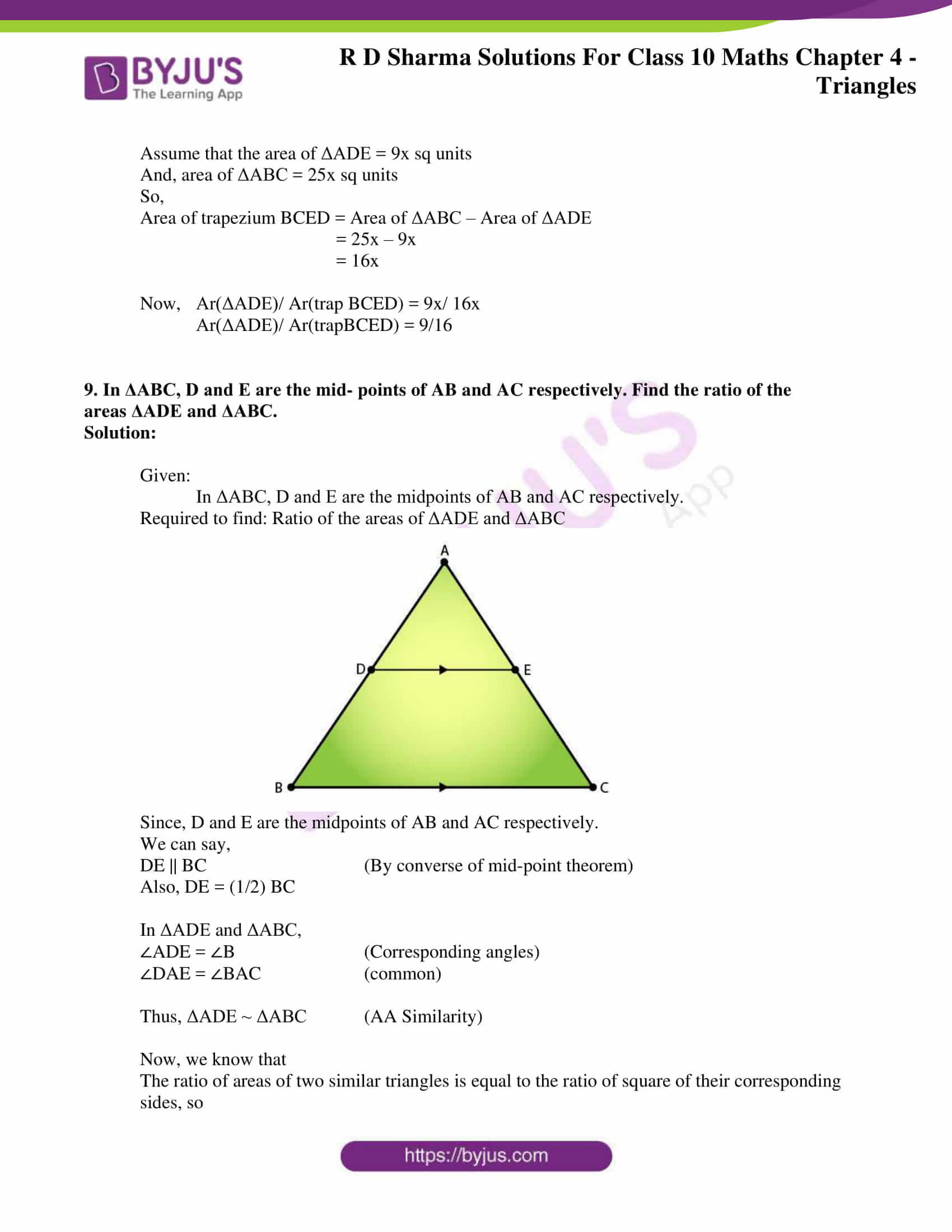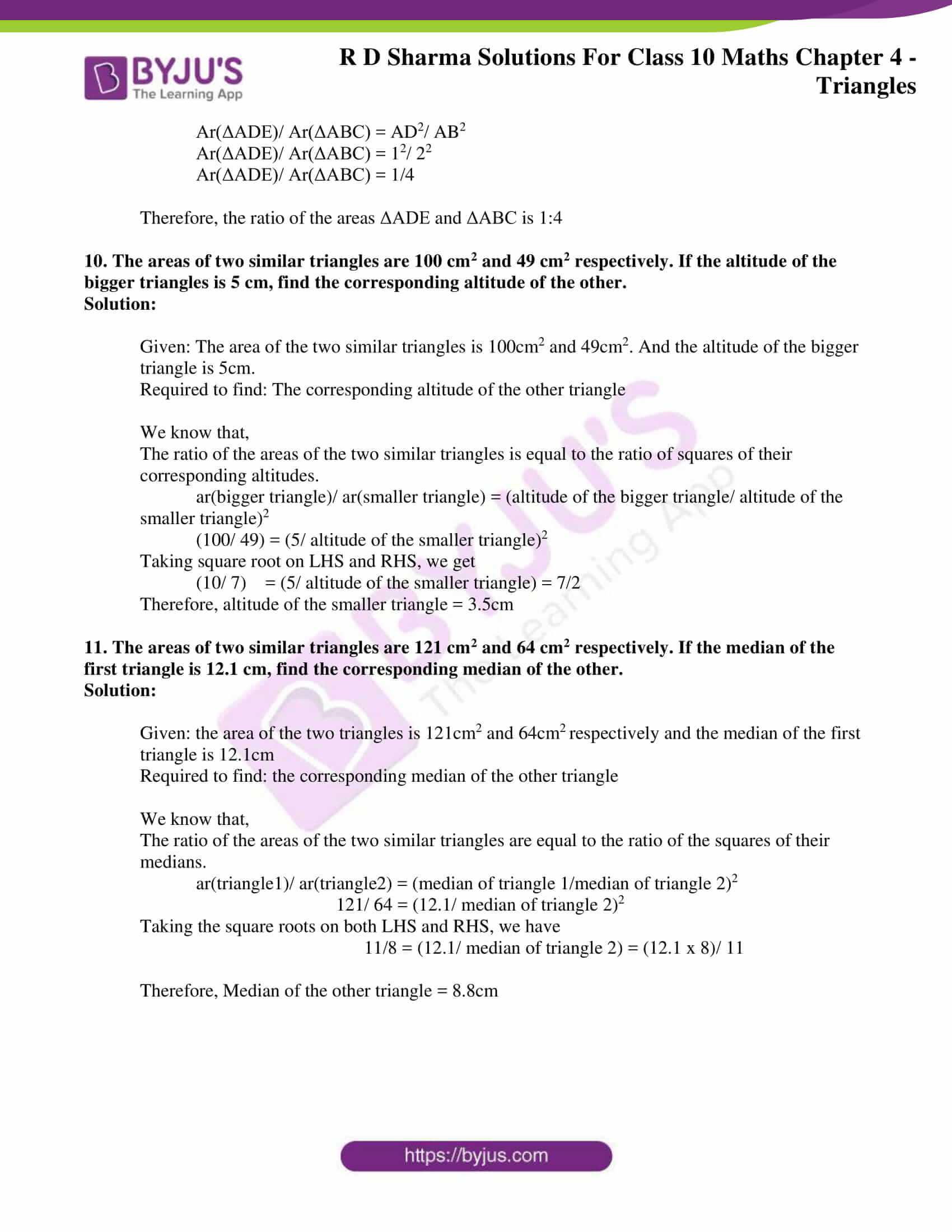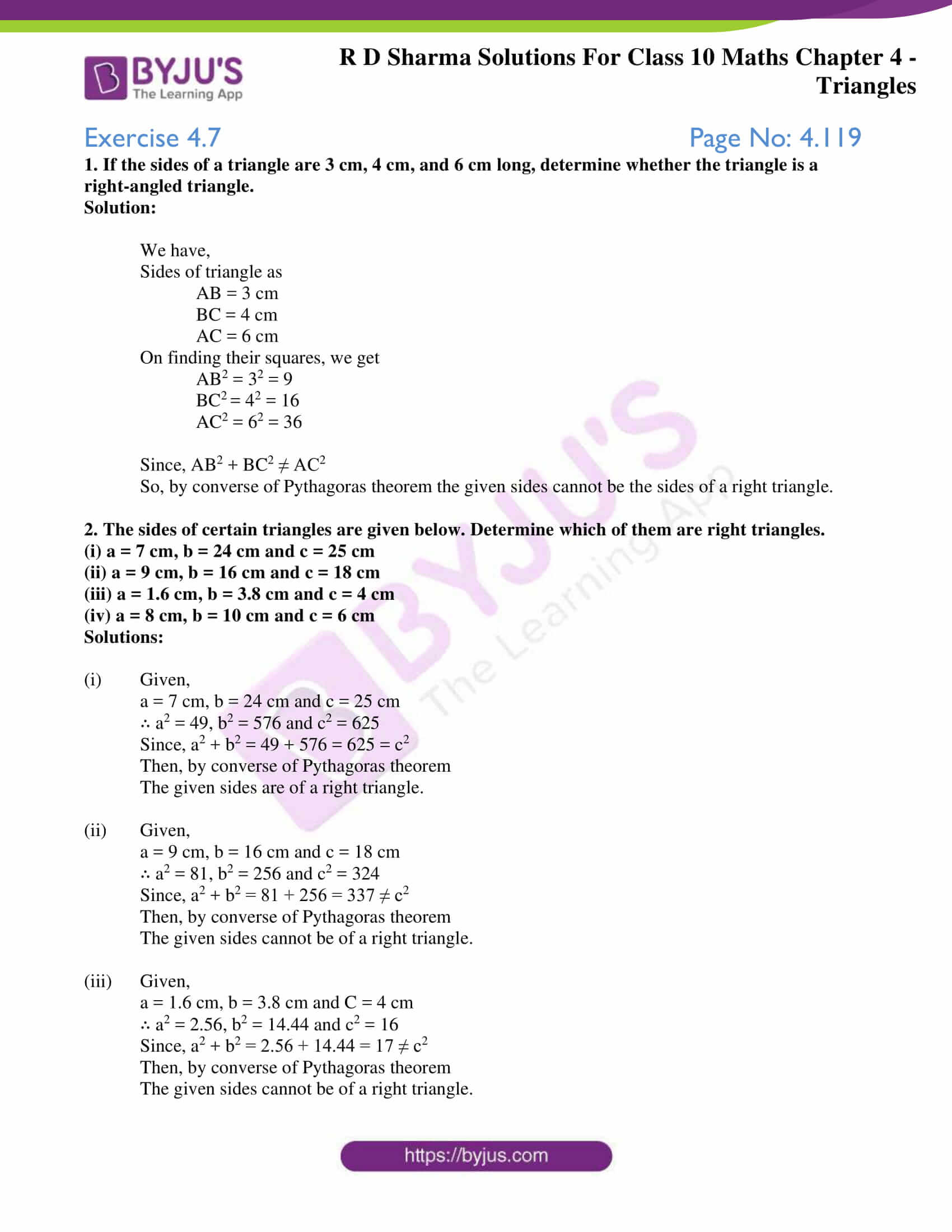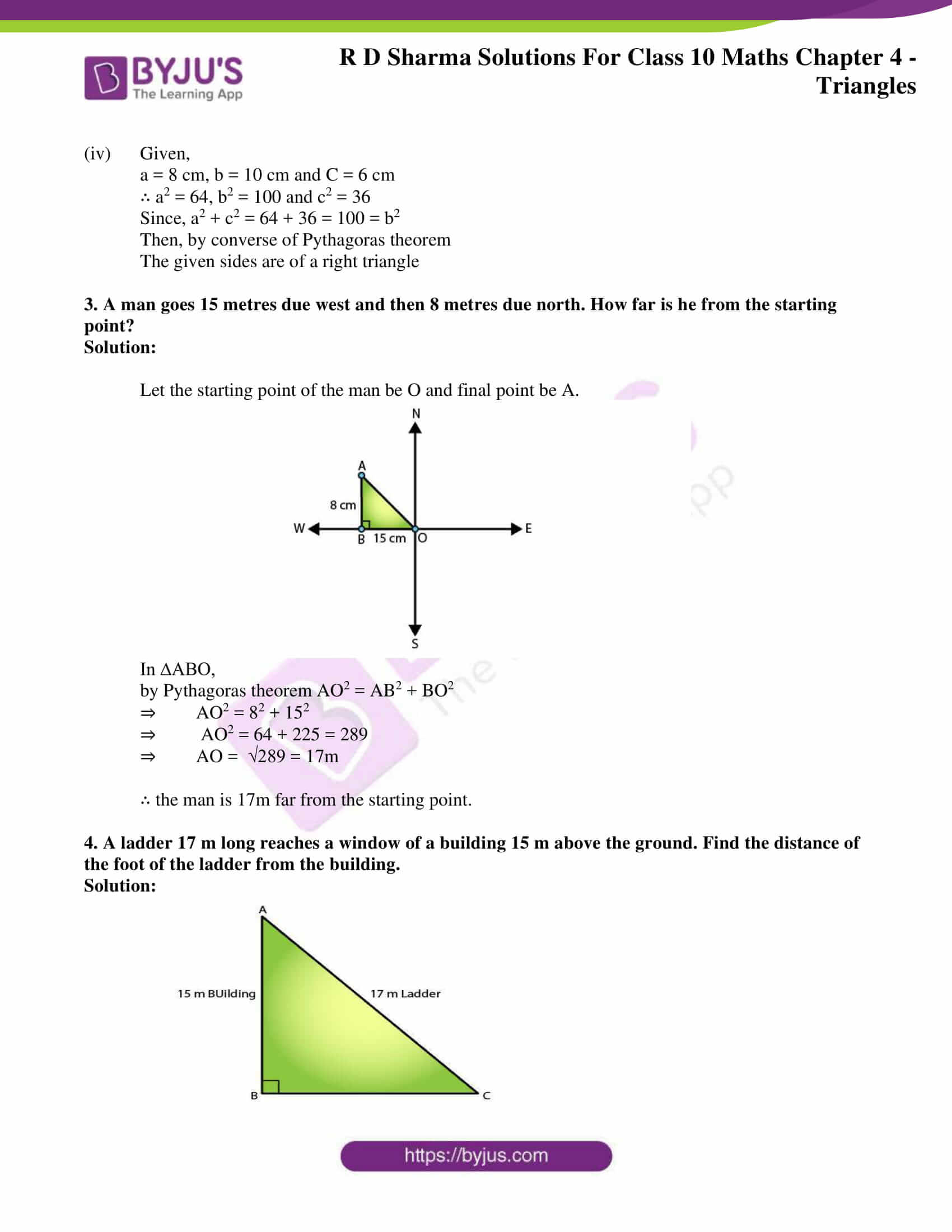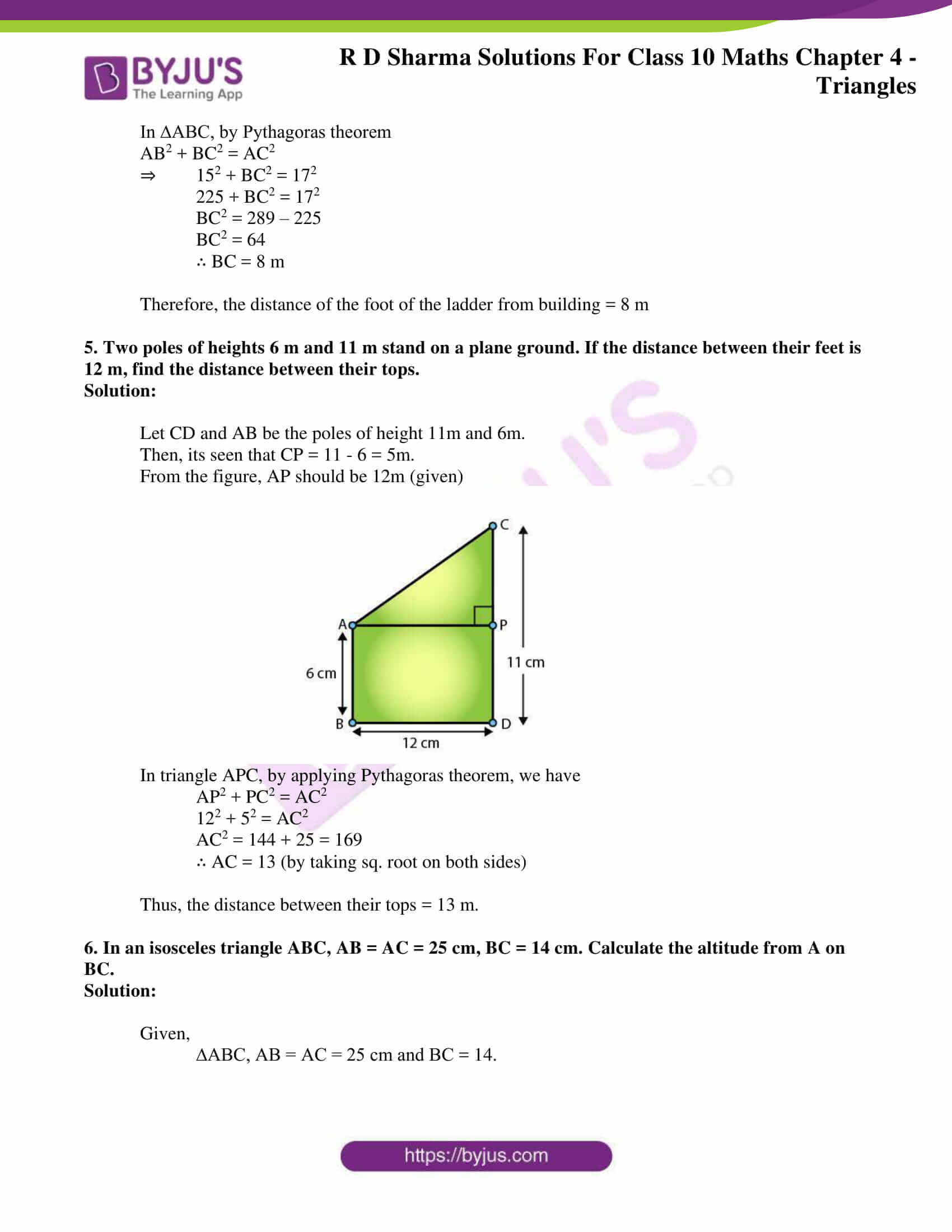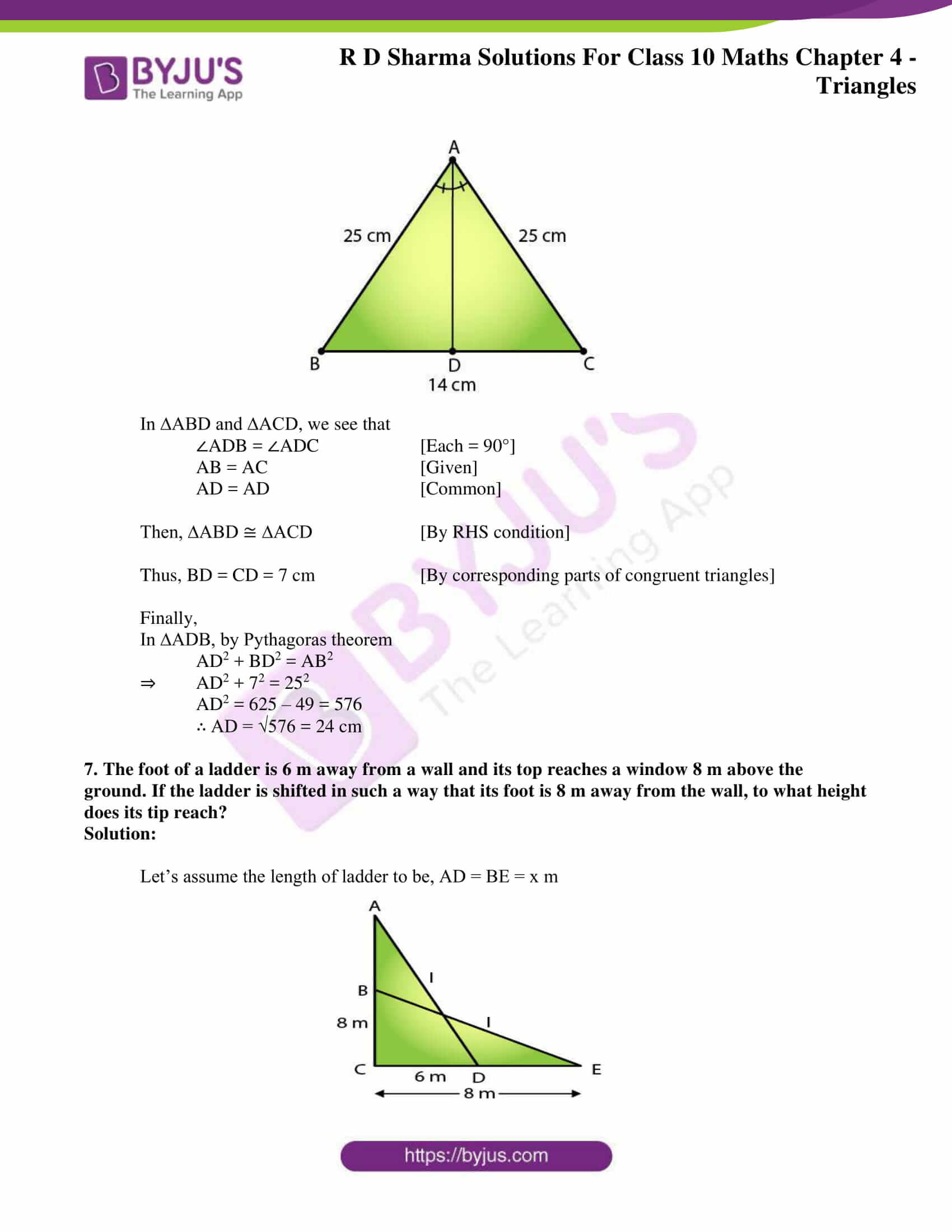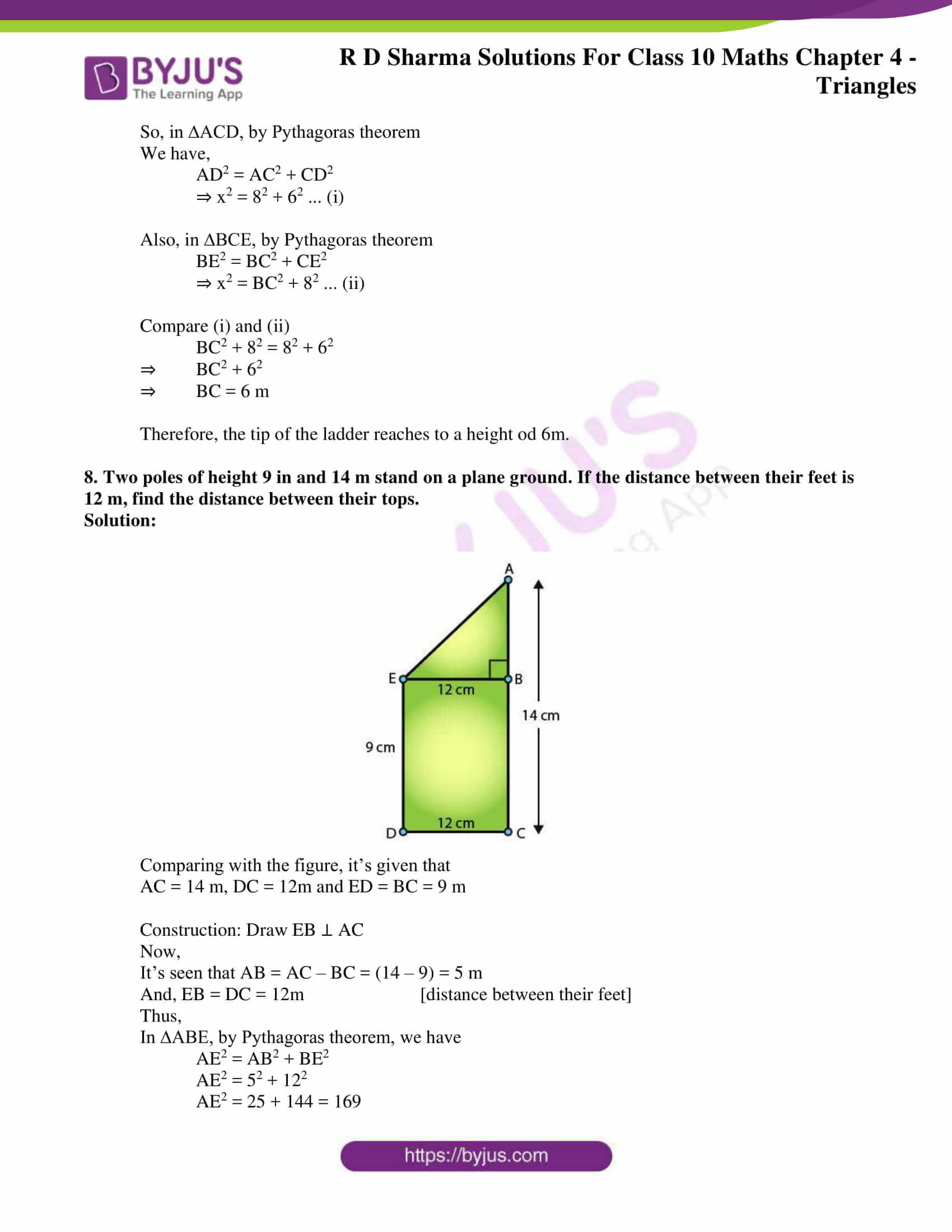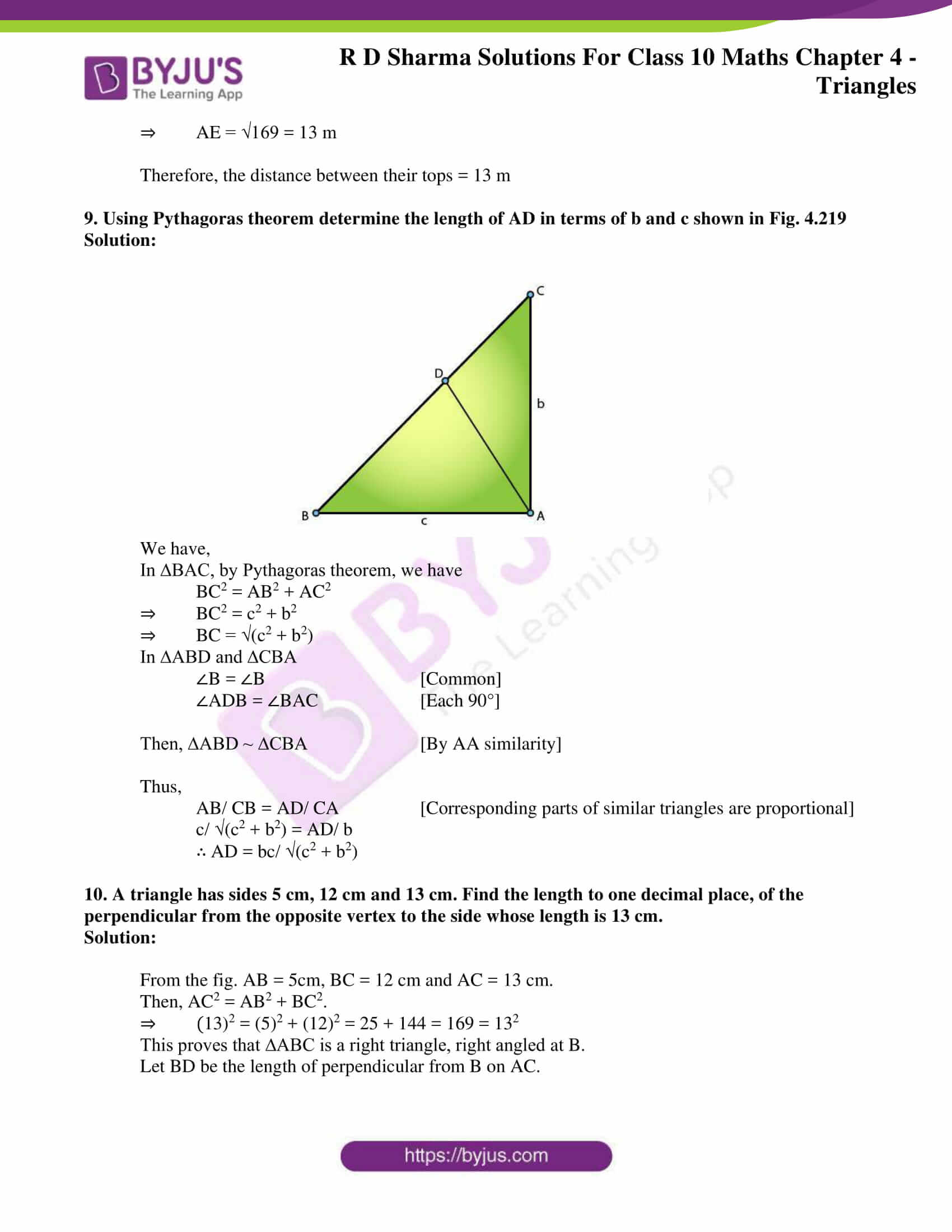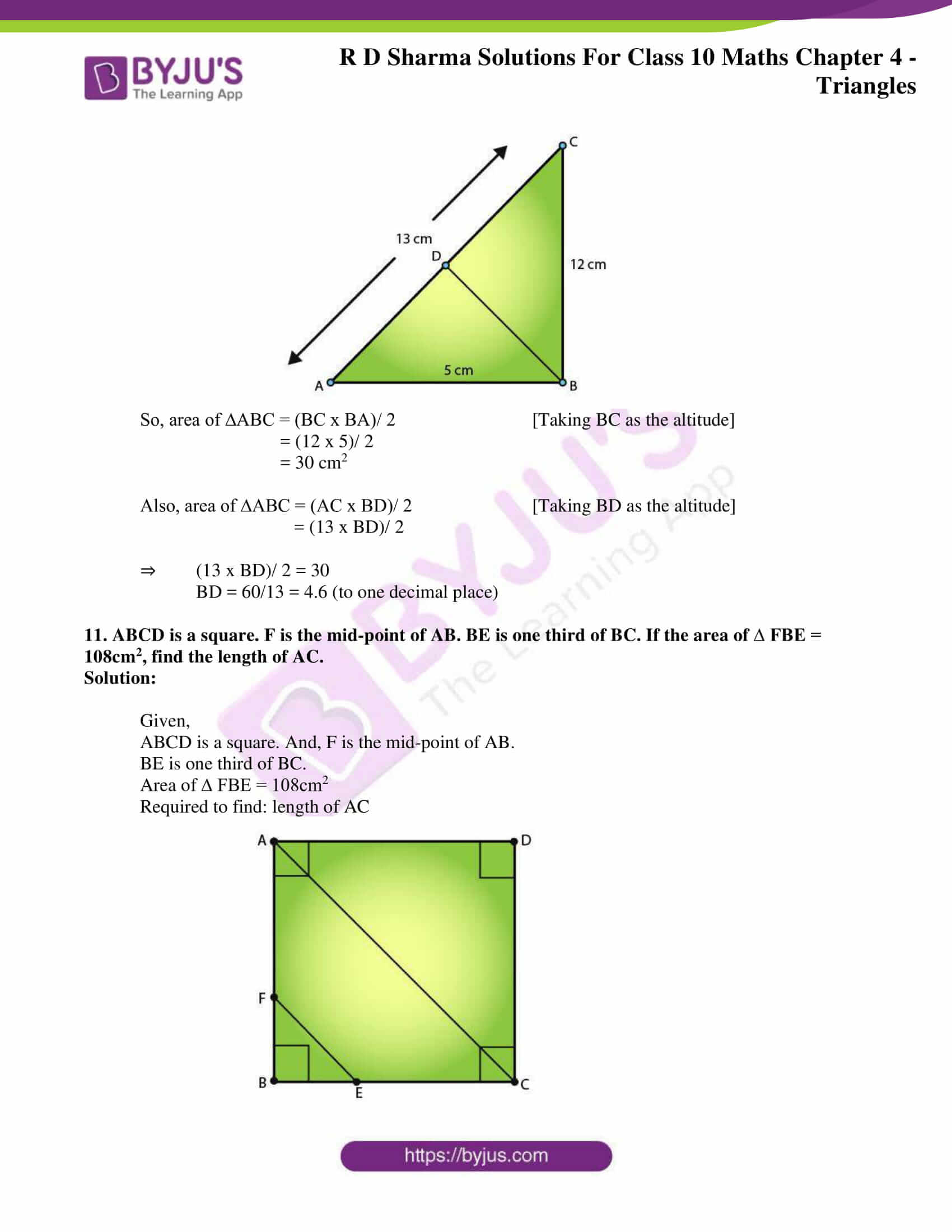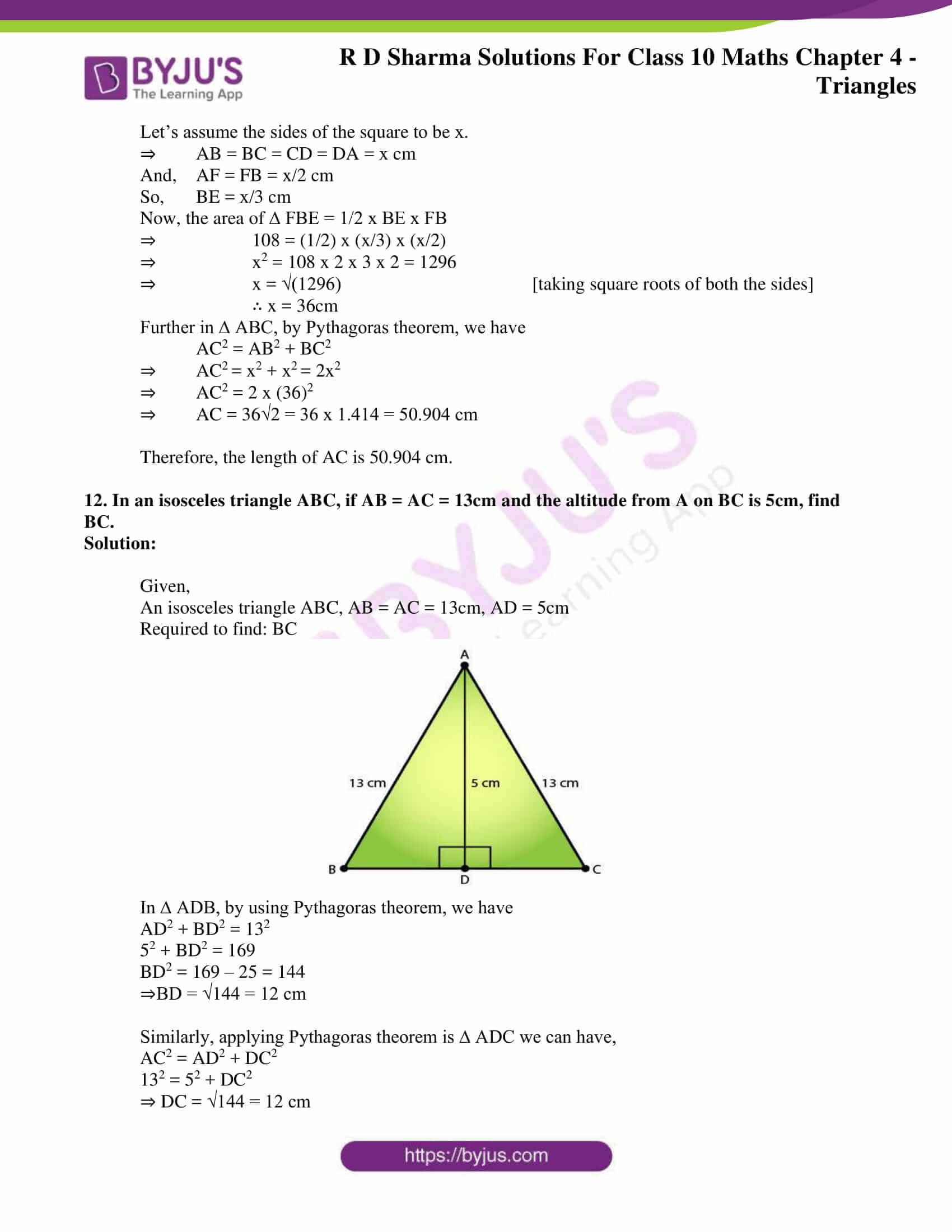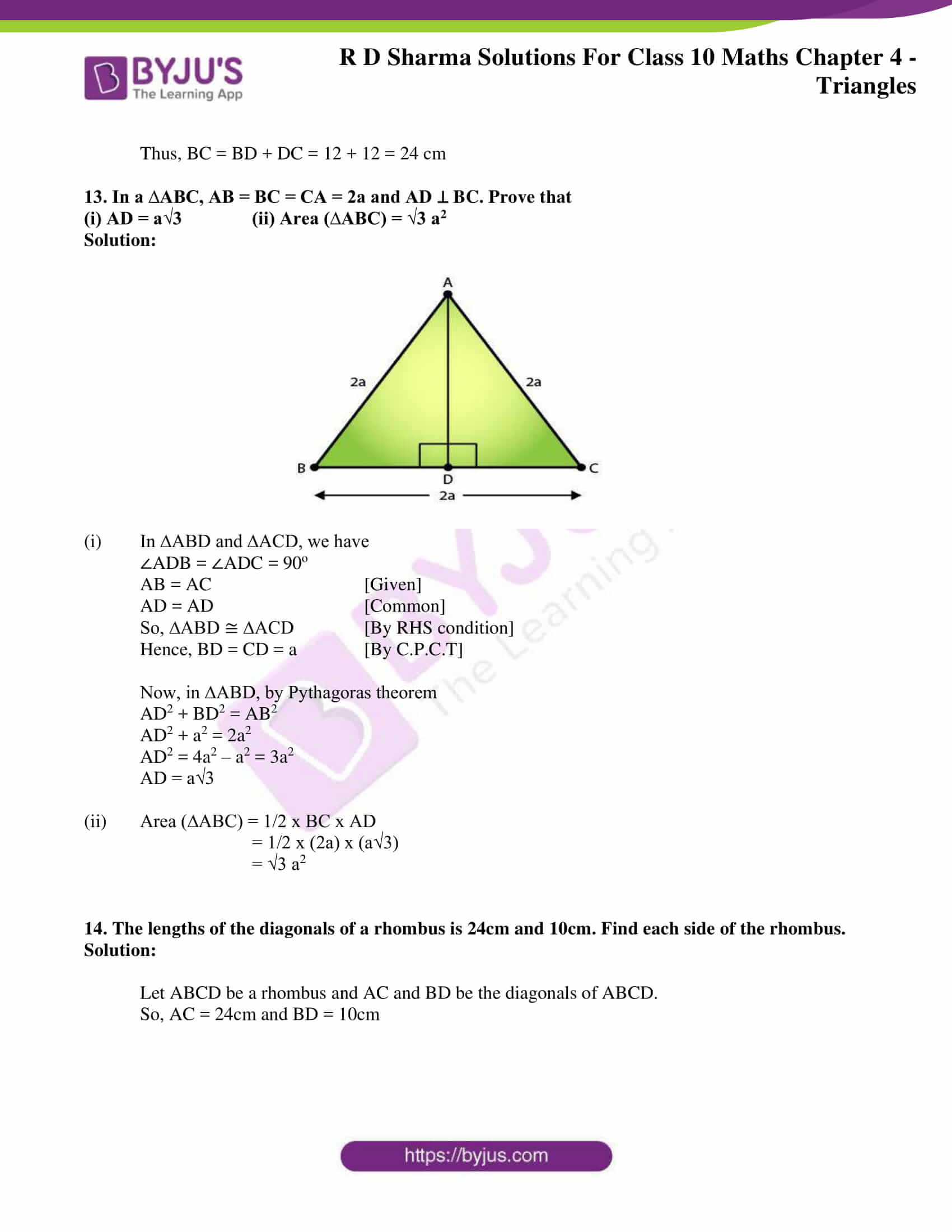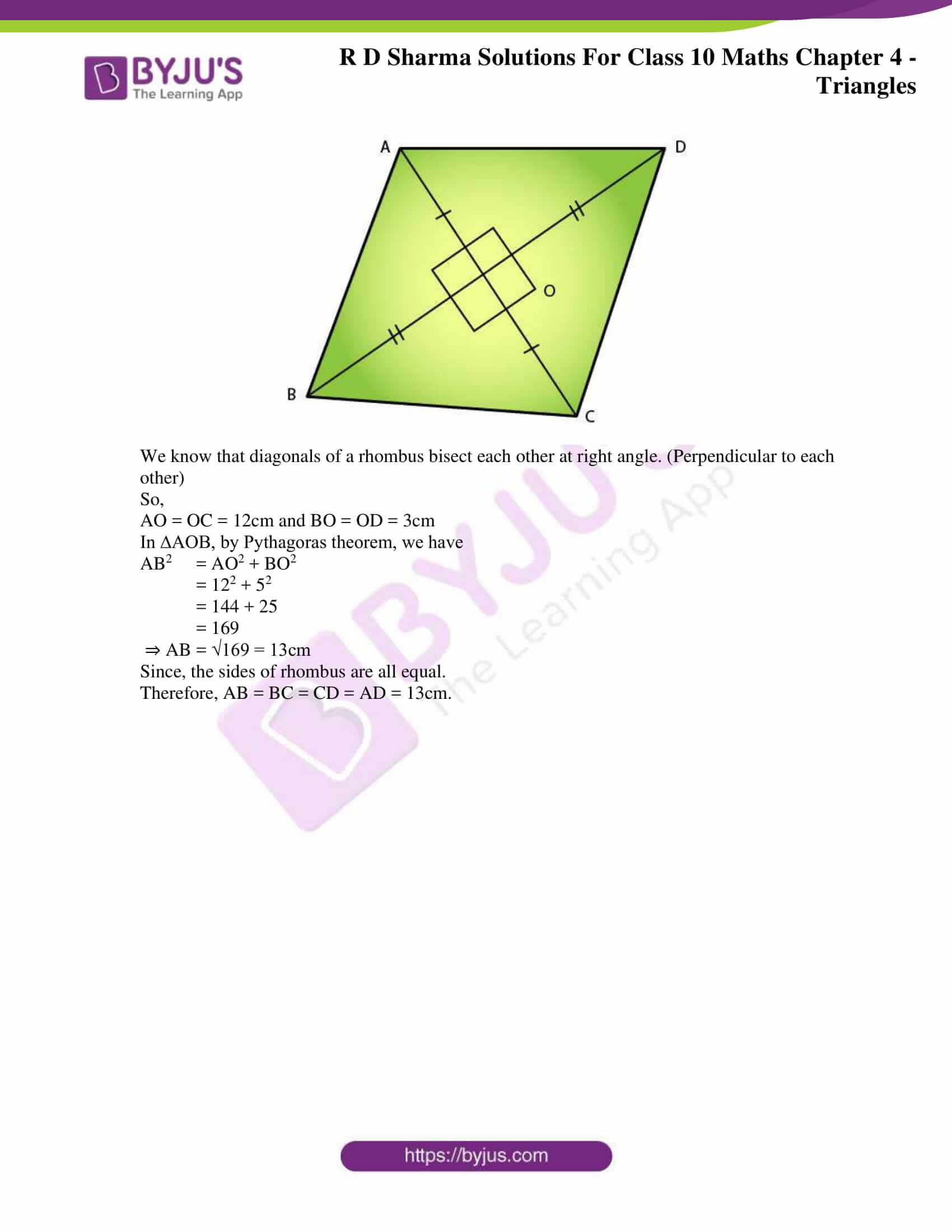### Access the RD Sharma Solutions for Class 10 Maths Chapter 4 – Triangles

Exercise 4.1 Page No: 4.3

1. Fill in the blanks using the correct word given in brackets.

(i) All circles are ____________ (congruent, similar).

(ii) All squares are ___________ (similar, congruent).

(iii) All ____________ triangles are similar (isosceles, equilaterals).

(iv) Two triangles are similar, if their corresponding angles are ____________ (proportional, equal)

(v) Two triangles are similar, if their corresponding sides are ____________ (proportional, equal)

(vi) Two polygons of the same number of sides are similar, if (a) ____________their corresponding angles are and their corresponding sides are (b)____________ (equal, proportional).

Solutions:

(i) All circles are similar.

(ii) All squares are similar.

(iii) All equilateral triangles are similar.

(iv) Two triangles are similar, if their corresponding angles are equal.

(v) Two triangles are similar, if their corresponding sides are proportional.

(vi) Two polygons of the same number of sides are similar, if (a) equal their corresponding angles and their corresponding sides are (b) proportional.

Exercise 4.2 Page No: 4.19

1. In a Δ ABC, D and E are points on the sides AB and AC, respectively, such that DE || BC.

i) If AD = 6 cm, DB = 9 cm and AE = 8 cm, Find AC.

Solution:

Given: Δ ABC, DE ∥ BC, AD = 6 cm, DB = 9 cm and AE = 8 cm.

Required to find AC.

By using Thales Theorem, [As DE ∥ BC]

Let CE = x

So then,

6/9 = 8/x

6x = 72 cm

x = 72/6 cm

x = 12 cm

∴ AC = AE + CE = 12 + 8 = 20.

ii) If AD/DB = 3/4 and AC = 15 cm, Find AE.

Solution:

Given: AD/BD = 3/4 and AC = 15 cm [As DE ∥ BC]

Required to find AE.

By using Thales Theorem, [As DE ∥ BC]

Let, AE = x, then CE = 15-x.

⇒ 3/4 = x/ (15–x)

45 – 3x = 4x

-3x – 4x = – 45

7x = 45

x = 45/7

x = 6.43 cm

∴ AE= 6.43cm

iii) If AD/DB = 2/3 and AC = 18 cm, Find AE.

Solution:

Given: AD/BD = 2/3 and AC = 18 cm

Required to find AE.

By using Thales Theorem, [As DE ∥ BC]

Let, AE = x and CE = 18 – x

⇒ 23 = x/ (18–x)

3x = 36 – 2x

5x = 36 cm

x = 36/5 cm

x = 7.2 cm

∴ AE = 7.2 cm

iv) If AD = 4 cm, AE = 8 cm, DB = x – 4 cm and EC = 3x – 19, find x.

Solution:

Given: AD = 4 cm, AE = 8 cm, DB = x – 4 and EC = 3x – 19

Required to find x.

By using Thales Theorem, [As DE ∥ BC]

Then, 4/ (x – 4) = 8/ (3x – 19)

4(3x – 19) = 8(x – 4)

12x – 76 = 8(x – 4)

12x – 8x = – 32 + 76

4x = 44 cm

x = 11 cm

v) If AD = 8 cm, AB = 12 cm and AE = 12 cm, find CE.

Solution:

Given: AD = 8 cm, AB = 12 cm, and AE = 12 cm.

Required to find CE,

By using Thales Theorem, [As DE ∥ BC]

8/4 = 12/CE

8 x CE = 4 x 12 cm

CE = (4 x 12)/8 cm

CE = 48/8 cm

∴ CE = 6 cm

vi) If AD = 4 cm, DB = 4.5 cm and AE = 8 cm, find AC.

Solution:

Given: AD = 4 cm, DB = 4.5 cm, AE = 8 cm

Required to find AC.

By using Thales Theorem, [As DE ∥ BC]

4/4.5 = 8/AC

AC = (4.5 × 8)/4 cm

∴ AC = 9 cm

vii) If AD = 2 cm, AB = 6 cm and AC = 9 cm, find AE.

Solution:

Given: AD = 2 cm, AB = 6 cm and AC = 9 cm

Required to find AE.

DB = AB – AD = 6 – 2 = 4 cm

By using Thales Theorem, [As DE ∥ BC]

2/4 = x/ (9–x)

4x = 18 – 2x

6x = 18

x = 3 cm

∴ AE= 3cm

viii) If AD/BD = 4/5 and EC = 2.5 cm, Find AE.

Solution:

Given: AD/BD = 4/5 and EC = 2.5 cm

Required to find AE.

By using Thales Theorem, [As DE ∥ BC]

Then, 4/5 = AE/2.5

∴ AE = 4 × 2.55 = 2 cm

ix) If AD = x cm, DB = x – 2 cm, AE = x + 2 cm, and EC = x – 1 cm, find the value of x.

Solution:

Given: AD = x, DB = x – 2, AE = x + 2 and EC = x – 1

Required to find the value of x.

By using Thales Theorem, [As DE ∥ BC]

So, x/ (x–2) = (x+2)/ (x–1)

x(x – 1) = (x – 2)(x + 2)

x2 – x – x2 + 4 = 0

x = 4

x) If AD = 8x – 7 cm, DB = 5x – 3 cm, AE = 4x – 3 cm, and EC = (3x – 1) cm, find the value of x.

Solution:

Given: AD = 8x – 7, DB = 5x – 3, AER = 4x – 3 and EC = 3x -1

Required to find x.

By using Thales Theorem, [As DE ∥ BC]

(8x–7)/ (5x–3) = (4x–3)/ (3x–1)

(8x – 7)(3x – 1) = (5x – 3)(4x – 3)

24x2 – 29x + 7 = 20x– 27x + 9

4x2 – 2x – 2 = 0

2(2x2 – x – 1) = 0

2x2 – x – 1 = 0

2x2 – 2x + x – 1 = 0

2x(x – 1) + 1(x – 1) = 0

(x – 1)(2x + 1) = 0

⇒ x = 1 or x = -1/2

We know that the side of a triangle can never be negative. Therefore, we take the positive value.

∴ x = 1

xi) If AD = 4x – 3, AE = 8x – 7, BD = 3x – 1, and CE = 5x – 3, find the value of x.

Solution:

Given: AD = 4x – 3, BD = 3x – 1, AE = 8x – 7 and EC = 5x – 3

Required to find x.

By using Thales Theorem, [As DE ∥ BC]

So, (4x–3)/ (3x–1) = (8x–7)/ (5x–3)

(4x – 3)(5x – 3) = (3x – 1)(8x – 7)

4x(5x – 3) -3(5x – 3) = 3x(8x – 7) -1(8x – 7)

20x2 – 12x – 15x + 9 = 24x2 – 29x + 7

20x2 -27x + 9 = 242 -29x + 7

⇒ -4x2 + 2x + 2 = 0

4x2 – 2x – 2 = 0

4x2 – 4x + 2x – 2 = 0

4x(x – 1) + 2(x – 1) = 0

(4x + 2)(x – 1) = 0

⇒ x = 1 or x = -2/4

We know that the side of a triangle can never be negative. Therefore, we take the positive value.

∴ x = 1

xii) If AD = 2.5 cm, BD = 3.0 cm, and AE = 3.75 cm, find the length of AC.

Solution:

Given: AD = 2.5 cm, AE = 3.75 cm and BD = 3 cm

Required to find AC.

By using Thales Theorem, [As DE ∥ BC]

2.5/ 3 = 3.75/ CE

2.5 x CE = 3.75 x 3

CE = 3.75×32.5

CE = 11.252.5

CE = 4.5

Now, AC = 3.75 + 4.5

∴ AC = 8.25 cm.

2. In a Δ ABC, D and E are points on the sides AB and AC, respectively. For each of the following cases show that DE  BC.

i) AB = 12 cm, AD = 8 cm, AE = 12 cm, and AC = 18 cm.

Solution:

Required to prove DE ∥ BC.

We have,

AB = 12 cm, AD = 8 cm, AE = 12 cm, and AC = 18 cm. (Given)

So,

BD = AB – AD = 12 – 8 = 4 cm

And,

CE = AC – AE = 18 – 12 = 6 cm

It’s seen that,

AE/CE = 12/6 = 1/2

Thus,

So, by the converse of Thale’s Theorem,

We have,

DE ∥ BC.

Hence, proved.

ii) AB = 5.6 cm, AD = 1.4 cm, AC = 7.2 cm, and AE = 1.8 cm.

Solution:

Required to prove DE ∥ BC.

We have,

AB = 5.6 cm, AD = 1.4 cm, AC = 7.2 cm, and AE = 1.8 cm. (Given)

So,

BD = AB – AD = 5.6 – 1.4 = 4.2 cm

And,

CE = AC – AE = 7.2 – 1.8 = 5.4 cm

It’s seen that,

AE/CE = 1.8/5.4 =1/3

Thus,

So, by the converse of Thale’s Theorem,

We have,

DE ∥ BC.

Hence, proved.

iii) AB = 10.8 cm, BD = 4.5 cm, AC = 4.8 cm, and AE = 2.8 cm.

Solution:

Required to prove DE ∥ BC.

We have

AB = 10.8 cm, BD = 4.5 cm, AC = 4.8 cm, and AE = 2.8 cm.

So,

AD = AB – DB = 10.8 – 4.5 = 6.3

And,

CE = AC – AE = 4.8 – 2.8 = 2

It’s seen that,

AD/BD = 6.3/ 4.5 = 2.8/ 2.0 = AE/CE = 7/5

So, by the converse of Thale’s Theorem,

We have,

DE ∥ BC.

Hence, proved.

iv) AD = 5.7 cm, BD = 9.5 cm, AE = 3.3 cm, and EC = 5.5 cm.

Solution:

Required to prove DE ∥ BC.

We have

AD = 5.7 cm, BD = 9.5 cm, AE = 3.3 cm, and EC = 5.5 cm

Now,

And,

AE/CE = 3.3/5.5 = 3/5

Thus,

So, by the converse of Thale’s Theorem,

We have,

DE ∥ BC.

Hence, proved.

3. In a Δ ABC, P and Q are the points on sides AB and AC, respectively, such that PQ  BC. If AP = 2.4 cm, AQ = 2 cm, QC = 3 cm and BC = 6 cm. Find AB and PQ.

Solution:

Given: Δ ABC, AP = 2.4 cm, AQ = 2 cm, QC = 3 cm, and BC = 6 cm. Also, PQ ∥ BC.

Required to find: AB and PQ.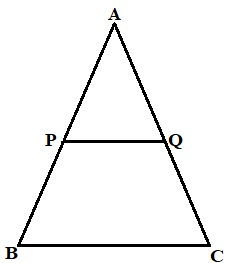By using Thales Theorem, we have [As it’s given that PQ ∥ BC]

AP/PB = AQ/ QC

2.4/PB = 2/3

2 x PB = 2.4 x 3

PB = (2.4 × 3)/2 cm

⇒ PB = 3.6 cm

Now finding, AB = AP + PB

AB = 2.4 + 3.6

⇒ AB = 6 cm

Now, considering Δ APQ and Δ ABC,

We have,

∠A = ∠A

∠APQ = ∠ABC (Corresponding angles are equal, PQ||BC and AB being a transversal)

Thus, Δ APQ and Δ ABC are similar to each other by AA criteria.

Now, we know that

Corresponding parts of similar triangles are propositional.

⇒ AP/AB = PQ/ BC

⇒ PQ = (AP/AB) x BC

= (2.4/6) x 6 = 2.4

∴ PQ = 2.4 cm.

4. In a Δ ABC, D and E are points on AB and AC, respectively, such that DE  BC. If AD = 2.4 cm, AE = 3.2 cm, DE = 2 cm and BC = 5 cm. Find BD and CE.

Solution:

Given: Δ ABC such that AD = 2.4 cm, AE = 3.2 cm, DE = 2 cm and BE = 5 cm. Also, DE ∥ BC.

Required to find: BD and CE.

As DE ∥ BC, AB is transversal.

∠APQ = ∠ABC (corresponding angles)

As DE ∥ BC, AC is transversal.

∠AED = ∠ACB (corresponding angles)

In Δ ADE and Δ ABC,

∠AED=∠ACB

∴ Δ ADE = Δ ABC (AA similarity criteria)

Now, we know that

Corresponding parts of similar triangles are propositional.

⇒ AD/AB = AE/AC = DE/BC

2.4/ (2.4 + DB) = 2/5 [Since, AB = AD + DB]

2.4 + DB = 6

DB = 6 – 2.4

DB = 3.6 cm

In the same way,

⇒ AE/AC = DE/BC

3.2/ (3.2 + EC) = 2/5 [Since AC = AE + EC]

3.2 + EC = 8

EC = 8 – 3.2

EC = 4.8 cm

∴ BD = 3.6 cm and CE = 4.8 cm.

Exercise 4.3 Page No: 4.31

1. In a Δ ABC, AD is the bisector of ∠ A, meeting side BC at D.

(i) if BD = 2.5 cm, AB = 5 cm, and AC = 4.2 cm, find DC.

Solution:

Given: Δ ABC and AD bisects ∠A, meeting side BC at D. And BD = 2.5 cm, AB = 5 cm, and AC = 4.2 cm.

Required to find: DC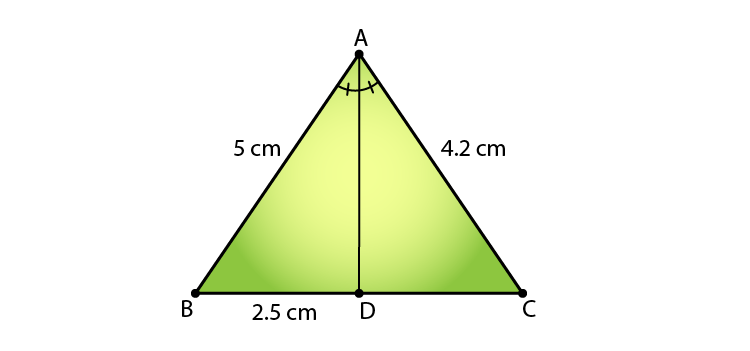Since AD is the bisector of ∠ A meeting side BC at D in Δ ABC,

⇒ AB/ AC = BD/ DC

5/ 4.2 = 2.5/ DC

5DC = 2.5 x 4.2

∴ DC = 2.1 cm

(ii) if BD = 2 cm, AB = 5 cm, and DC = 3 cm, find AC.

Solution:

Given: Δ ABC and AD bisects ∠A, meeting side BC at D. And BD = 2 cm, AB = 5 cm, and DC = 3 cm.

Required to find: AC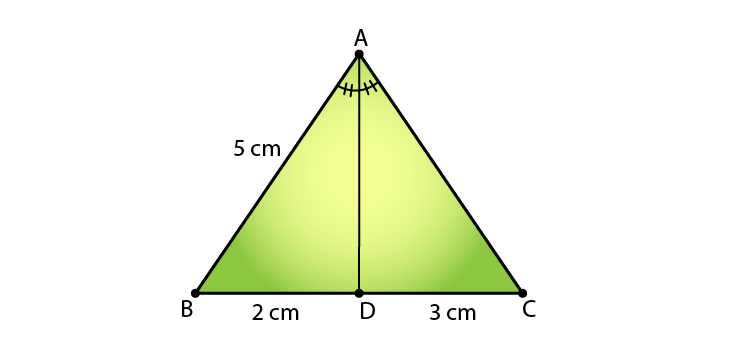Since AD is the bisector of ∠ A meeting side BC at D in Δ ABC,

⇒ AB/ AC = BD/ DC

5/ AC = 2/ 3

2AC = 5 x 3

∴ AC = 7.5 cm

(iii) if AB = 3.5 cm, AC = 4.2 cm, and DC = 2.8 cm, find BD.

Solution:

Given: Δ ABC and AD bisects ∠A, meeting side BC at D. And AB = 3.5 cm, AC = 4.2 cm, and DC = 2.8 cm.

Required to find: BD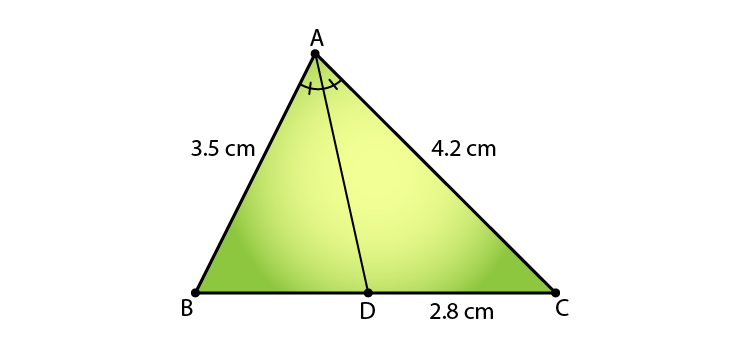Since AD is the bisector of ∠ A meeting side BC at D in Δ ABC,

⇒ AB/ AC = BD/ DC

3.5/ 4.2 = BD/ 2.8

4.2 x BD = 3.5 x 2.8

BD = 7/3

∴ BD = 2.3 cm

(iv) if AB = 10 cm, AC = 14 cm, and BC = 6 cm, find BD and DC.

Solution:

Given: In Δ ABC, AD is the bisector of ∠A meeting side BC at D. And, AB = 10 cm, AC = 14 cm, and BC = 6 cm.

Required to find: BD and DC.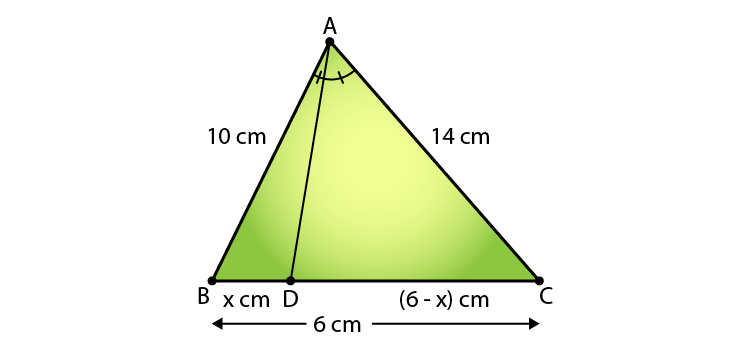Since AD is the bisector of ∠A,

We have,

AB/AC = BD/DC (AD is bisector of ∠ A and side BC)

Then, 10/ 14 = x/ (6 – x)

14x = 60 – 6x

20x = 60

x = 60/20

∴ BD = 3 cm and DC = (6 – 3) = 3 cm.

(v) if AC = 4.2 cm, DC = 6 cm, and BC = 10 cm, find AB.

Solution:

Given: Δ ABC and AD bisects ∠A, meeting side BC at D. And AC = 4.2 cm, DC = 6 cm, and BC = 10 cm.

Required to find: AB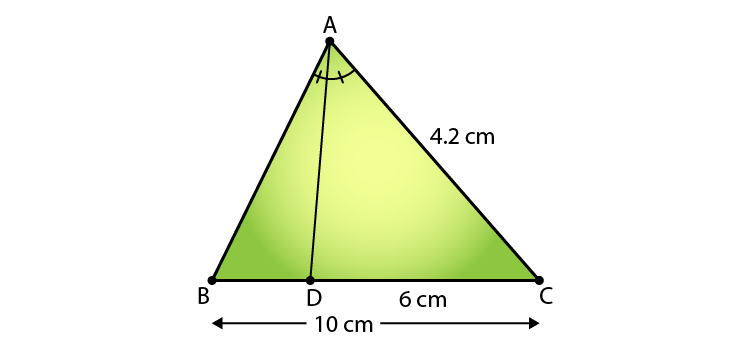Since AD is the bisector of ∠ A meeting side BC at D in Δ ABC,

⇒ AB/ AC = BD/ DC

AB/ 4.2 = BD/ 6

We know that,

BD = BC – DC = 10 – 6 = 4 cm

⇒ AB/ 4.2 = 4/ 6

AB = (2 x 4.2)/ 3

∴ AB = 2.8 cm

(vi) if AB = 5.6 cm, AC = 6 cm, and DC = 3 cm, find BC.

Solution:

Given: Δ ABC and AD bisects ∠A, meeting side BC at D. And AB = 5.6 cm, AC = 6 cm, and DC = 3 cm.

Required to find: BC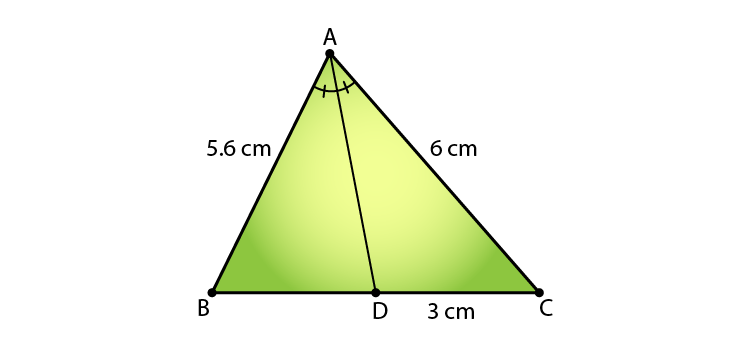Since AD is the bisector of ∠ A meeting side BC at D in Δ ABC,

⇒ AB/ AC = BD/ DC

5.6/ 6 = BD/ 3

BD = 5.6/ 2 = 2.8cm

And we know that,

BD = BC – DC

2.8 = BC – 3

∴ BC = 5.8 cm

(vii) if AB = 5.6 cm, BC = 6 cm, and BD = 3.2 cm, find AC.

Solution:

Given: Δ ABC and AD bisects ∠A, meeting side BC at D. And AB = 5.6 cm, BC = 6 cm, and BD = 3.2 cm.

Required to find: AC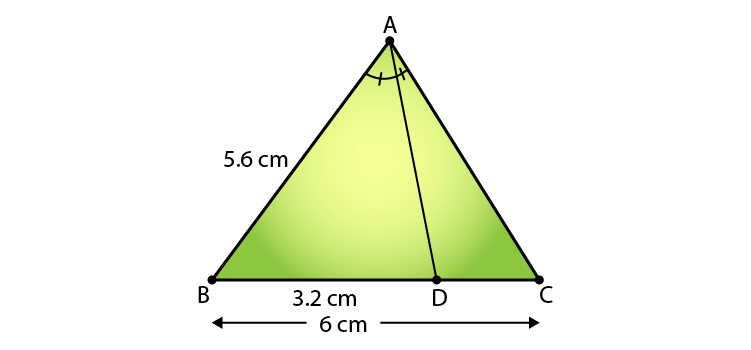Since AD is the bisector of ∠ A meeting side BC at D in Δ ABC,

⇒ AB/ AC = BD/ DC

5.6/ AC = 3.2/ DC

And we know that

BD = BC – DC

3.2 = 6 – DC

∴ DC = 2.8 cm

⇒ 5.6/ AC = 3.2/ 2.8

AC = (5.6 x 2.8)/ 3.2

∴ AC = 4.9 cm

(viii) if AB = 10 cm, AC = 6 cm, and BC = 12 cm, find BD and DC.

Solution:

Given: Δ ABC and AD bisects ∠A, meeting side BC at D. AB = 10 cm, AC = 6 cm, and BC = 12 cm.

Required to find: DC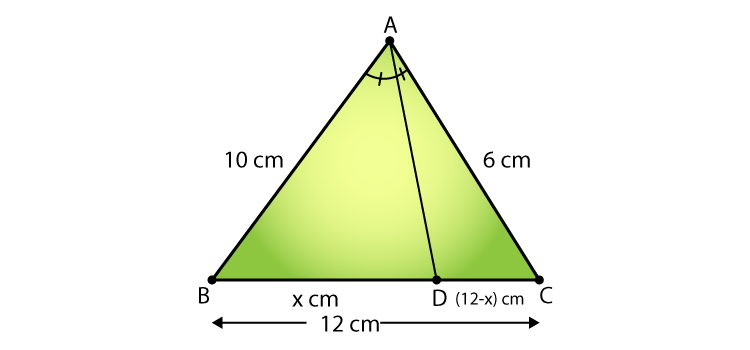Since AD is the bisector of ∠ A meeting side BC at D in Δ ABC,

⇒ AB/ AC = BD/ DC

10/ 6 = BD/ DC …….. (i)

And we know that

BD = BC – DC = 12 – DC

Let BD = x,

⇒ DC = 12 – x

Thus, (i) becomes

10/ 6 = x/ (12 – x)

5(12 – x) = 3x

60 -5x = 3x

∴ x = 60/8 = 7.5

Hence, DC = 12 – 7.5 = 4.5cm and BD = 7.5 cm

2. In figure 4.57, AE is the bisector of the exterior ∠CAD meeting BC produced in E. If AB = 10 cm, AC = 6 cm, and BC = 12 cm, find CE.

Solution:

Given: AE is the bisector of the exterior CAD and AB = 10 cm, AC = 6 cm, and BC = 12 cm.

Required to find: CE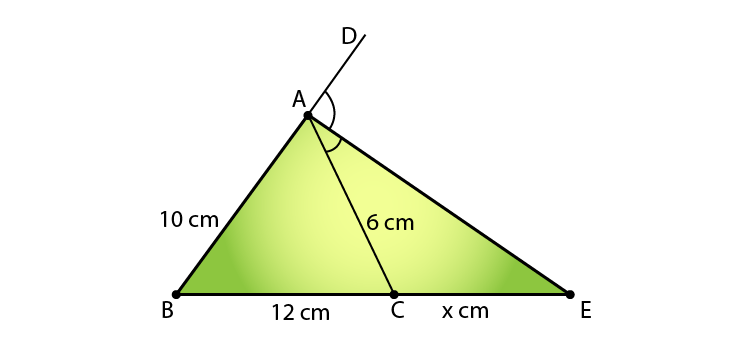Since AE is the bisector of the exterior CAD,

BE / CE = AB / AC

Let’s take CE as x.

So, we have

BE/ CE = AB/ AC

(12+x)/ x = 10/ 6

6x + 72 = 10x

10x – 6x = 72

4x = 72

∴ x = 18

Therefore, CE = 18 cm.

3.  In fig. 4.58, Δ ABC is a triangle such that AB/AC = BD/DC, ∠B=70o, ∠C = 50o, find ∠BAD.

Solution:

Given: Δ ABC, such that AB/AC = BD/DC, ∠B = 70o and ∠C = 50o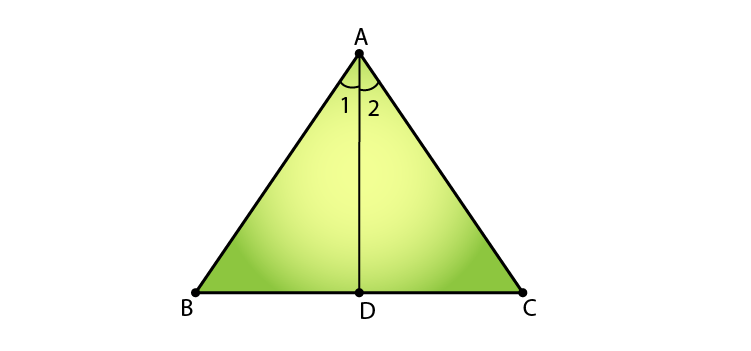We know that,

In ΔABC,

∠A = 180 – (70 + 50) [Angle sum property of a triangle]

= 180 – 120

= 60o

Since,

AB/AC = BD/DC,

AD is the angle bisector of angle ∠A.

Thus,

∠BAD = ∠A/2 = 60/2 = 30o

Exercise 4.4 Page No: 4.37

1. (i) In fig. 4.70, if AB∥CD, find the value of x.

Solution:

It’s given that AB∥CD.

Required to find the value of x.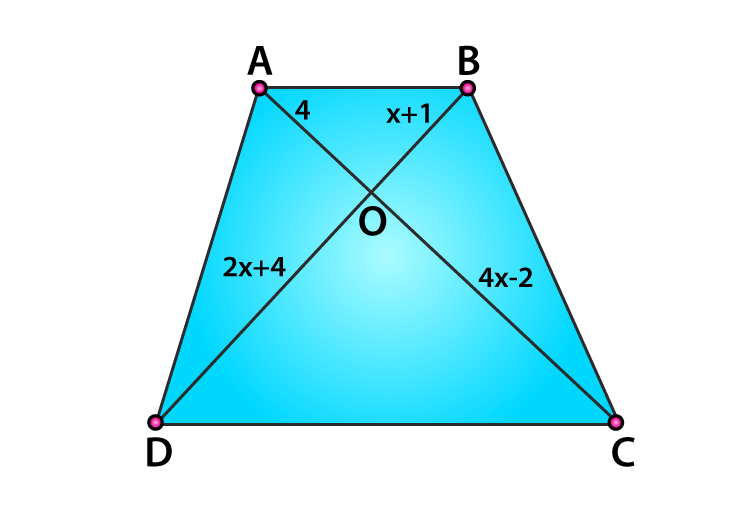We know that,

Diagonals of a parallelogram bisect each other.

So,

AO/ CO = BO/ DO

⇒ 4/ (4x – 2) = (x +1)/ (2x + 4)

4(2x + 4) = (4x – 2)(x +1)

8x + 16 = x(4x – 2) + 1(4x – 2)

8x + 16 = 4x2 – 2x + 4x – 2

-4x2 + 8x + 16 + 2 – 2x = 0

-4x+ 6x + 8 = 0

4x2 – 6x – 18 = 0

4x2 – 12x + 6x – 18 = 0

4x(x – 3) + 6(x – 3) = 0

(4x + 6) (x – 3) = 0

x = – 6/4 or x = 3

(ii)  In fig. 4.71, if AB∥CD, find the value of x.

Solution:

It’s given that AB∥CD.

Required to find the value of x.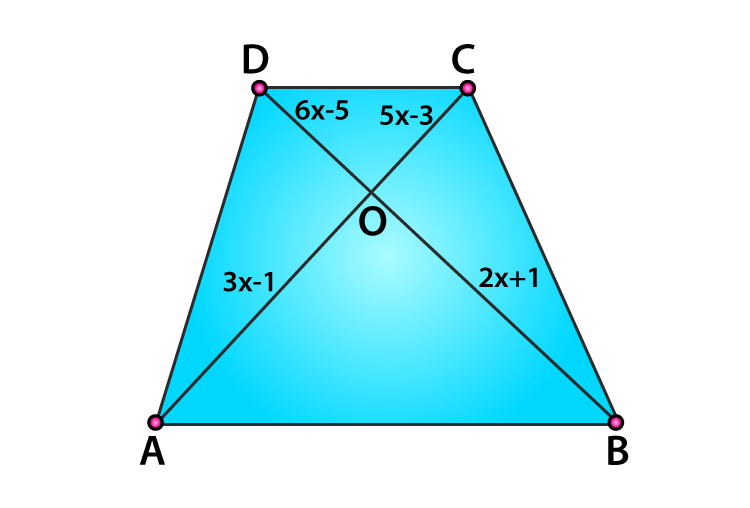We know that,

Diagonals of a parallelogram bisect each other.

So,

AO/ CO = BO/ DO

⇒ (6x – 5)/ (2x + 1) = (5x – 3)/ (3x – 1)

(6x – 5)(3x – 1) = (2x + 1)(5x – 3)

3x(6x – 5) – 1(6x – 5) = 2x(5x – 3) + 1(5x – 3)

18x2 – 10x2 – 21x + 5 + x +3 = 0

8x2 – 16x – 4x + 8 = 0

8x(x – 2) – 4(x – 2) = 0

(8x – 4)(x – 2) = 0

x = 4/8 = 1/2 or x = -2

x= 1/2

(iii) In fig. 4.72, if AB ∥ CD. If OA = 3x – 19, OB = x – 4, OC = x- 3 and OD = 4, find x.

Solution:

It’s given that AB∥CD.

Required to find the value of x.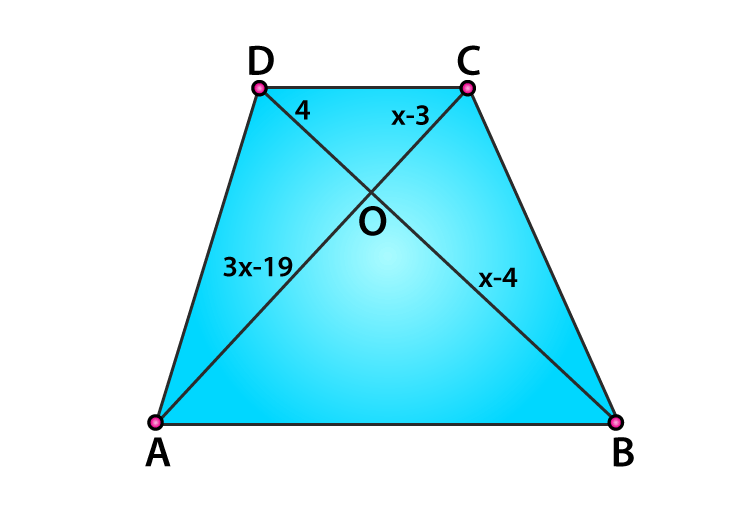We know that,

Diagonals of a parallelogram bisect each other.

So,

AO/ CO = BO/ DO

(3x – 19)/ (x – 3) = (x–4)/ 4

4(3x – 19) = (x – 3) (x – 4)

12x – 76 = x(x – 4) -3(x – 4)

12x – 76 = x2 – 4x – 3x + 12

-x2 + 7x – 12 + 12x -76 = 0

-x+ 19x – 88 = 0

x– 19x + 88 = 0

x2 – 11x – 8x + 88 = 0

x(x – 11) – 8(x – 11) = 0

x = 11 or x = 8

Exercise 4.5 Page No: 4.37

1. In fig. 4.136, ΔACB ∼ ΔAPQ. If BC = 8 cm, PQ = 4 cm, BA = 6.5 cm and AP = 2.8 cm, find CA and AQ.

Solution:

Given,

ΔACB ∼ ΔAPQ

BC = 8 cm, PQ = 4 cm, BA = 6.5 cm and AP = 2.8 cm

Required to find: CA and AQ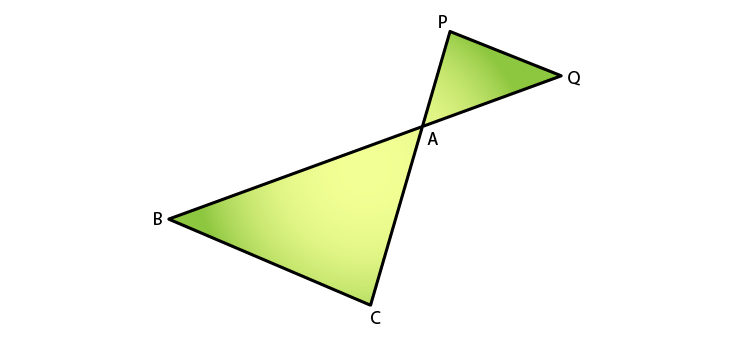We know that,

ΔACB ∼ ΔAPQ [given]

BA/ AQ = CA/ AP = BC/ PQ [Corresponding Parts of Similar Triangles]

So,

6.5/ AQ = 8/ 4

AQ = (6.5 x 4)/ 8

AQ = 3.25 cm

Similarly, as

CA/ AP = BC/ PQ

CA/ 2.8 = 8/ 4

CA = 2.8 x 2

CA = 5.6 cm

Hence, CA = 5.6 cm and AQ = 3.25 cm.

2. In fig.4.137, AB ∥ QR, find the length of PB.

Solution:

Given,

ΔPQR, AB ∥ QR and

AB = 3 cm, QR = 9 cm and PR = 6 cm

Required to find: PB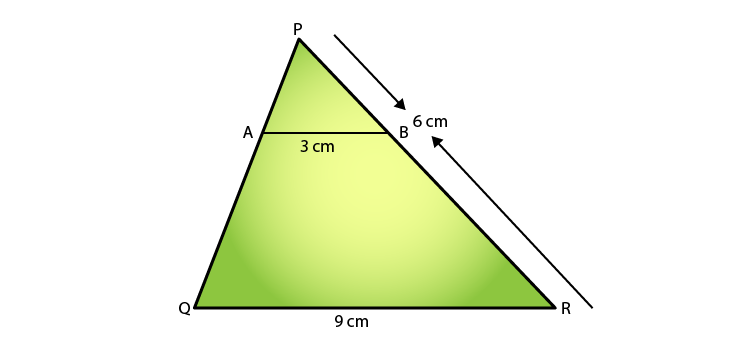In ΔPAB and ΔPQR,

We have,

∠P = ∠P  [Common]

∠PAB = ∠PQR [Corresponding angles as AB||QR with PQ as the transversal]

⇒ ΔPAB ΔPQR [By AA similarity criteria]

Hence,

AB/ QR = PB/ PR [Corresponding Parts of Similar Triangles are propositional]

⇒ 3/ 9 = PB/6

PB = 6/3

Therefore, PB = 2 cm

3. In fig. 4.138 given, XY∥BC. Find the length of XY.

Solution:

Given,

XY∥BC

AX = 1 cm, XB = 3 cm and BC = 6 cm

Required to find: XY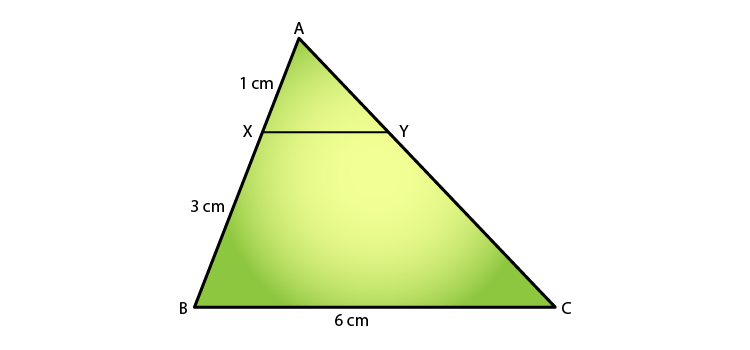In ΔAXY and ΔABC,

We have,

∠A = ∠A  [Common]

∠AXY = ∠ABC [Corresponding angles as AB||QR with PQ as the transversal]

⇒ ΔAXY ΔABC [By AA similarity criteria]

Hence,

XY/ BC = AX/ AB [Corresponding Parts of Similar Triangles are propositional]

We know that,

(AB = AX + XB = 1 + 3 = 4)

XY/6 = 1/4

XY/1 = 6/4

Therefore, XY = 1.5 cm

4. In a right-angled triangle with sides a and b and hypotenuse c, the altitude drawn on the hypotenuse is x. Prove that ab = cx.

Solution:

Consider ΔABC to be a right-angle triangle having sides a and b and hypotenuse c. Let BD be the altitude drawn on the hypotenuse AC.

Required to prove: ab = cx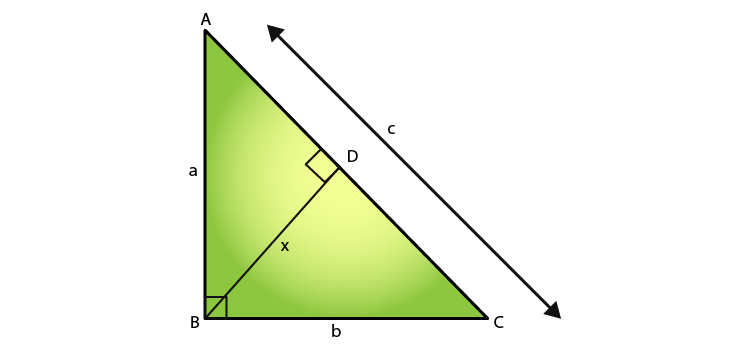We know that,

In ΔACB and ΔCDB

∠B = ∠B  [Common]

∠ACB = ∠CDB = 90o

⇒ ΔACB ΔCDB [By AA similarity criteria]

Hence,

AB/ BD = AC/ BC [Corresponding Parts of Similar Triangles are propositional]

a/ x = c/ b

⇒ xc = ab

Therefore, ab = cx

5. In fig. 4.139, ∠ABC = 90 and BD⊥AC. If BD = 8 cm, and AD = 4 cm, find CD.

Solution:

Given,

∠ABC = 90o and BD⊥AC

BD = 8 cm

Required to find: CD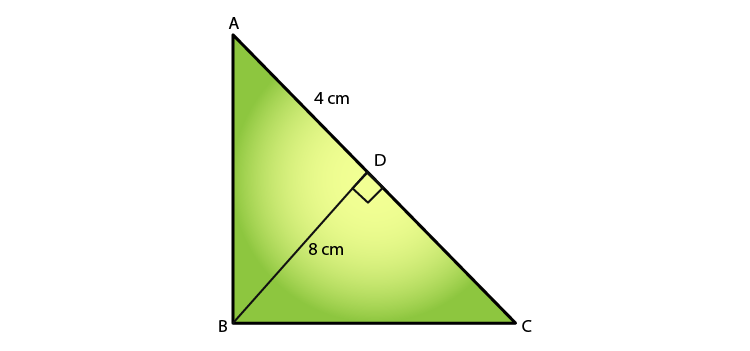We know that,

ABC is a right-angled triangle, and BD⊥AC.

Then, ΔDBA∼ΔDCB  [By AA similarity]

(8)2 = 4 x DC

DC = 64/4 = 16 cm

Therefore, CD = 16 cm

6. In fig.4.140, ∠ABC = 90o and BD ⊥ AC. If AC = 5.7 cm, BD = 3.8 cm and CD = 5.4 cm, Find BC.

Solution:

Given:

BD ⊥ AC

AC = 5.7 cm, BD = 3.8 cm and CD = 5.4 cm

∠ABC = 90o

Required to find: BC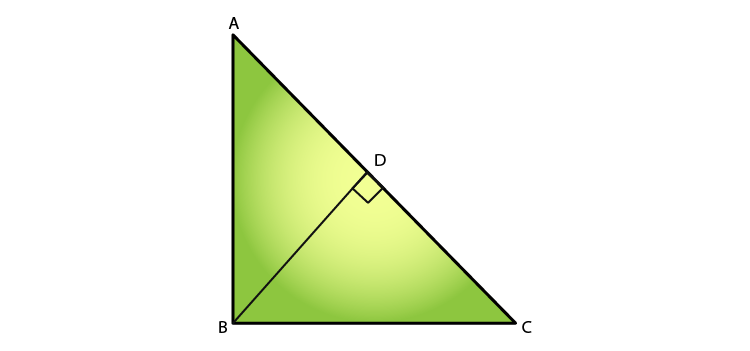We know that,

ΔABC ∼ ΔBDC [By AA similarity]

∠BCA = ∠DCA = 90o

∠AXY = ∠ABC [Common]

Thus,

AB/ BD = BC/ CD [Corresponding Parts of Similar Triangles are propositional]

5.7/ 3.8 = BC/ 5.4

BC = (5.7 x 5.4)/ 3.8 = 8.1

Therefore, BC = 8.1 cm

7. In the fig.4.141 given, DE ∥ BC, such that AE = (1/4)AC. If AB = 6 cm, find AD.

Solution:

Given:

DE∥BC

AE = (1/4)AC

AB = 6 cm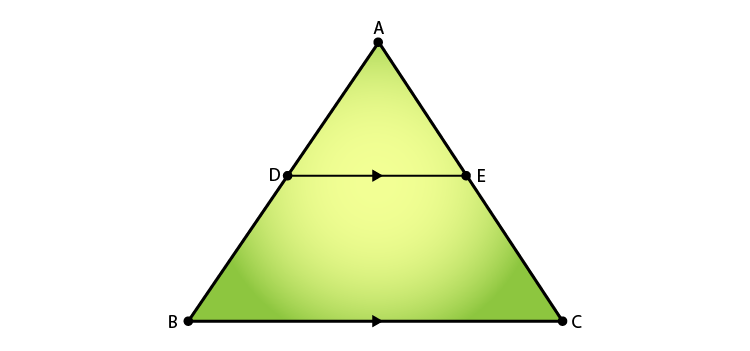We have,

∠A = ∠A  [Common]

∠ADE = ∠ABC [Corresponding angles as AB||QR with PQ as the transversal]

⇒ ΔADE ΔABC [By AA similarity criteria]

Then,

AD/AB = AE/ AC [Corresponding Parts of Similar Triangles are propositional]

8. In the fig.4.142 given, if AB ⊥ BC, DC ⊥ BC, and DE ⊥ AC, prove that ΔCED ∼ ΔABC.

Solution:

Given:

AB ⊥ BC,

DC ⊥ BC,

DE ⊥ AC

Required to prove: ΔCED∼ΔABC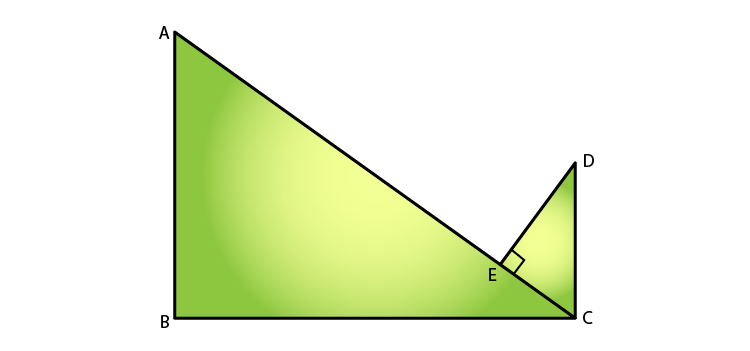We know that,

From ΔABC and ΔCED

∠B = ∠E = 90o   [given]

∠BAC = ∠ECD     [alternate angles since, AB || CD with BC as transversal]

Therefore, ΔCED∼ΔABC  [AA similarity]

9. Diagonals AC and BD of a trapezium ABCD with AB ∥ DC intersect each other at point O. Using the similarity criterion for two triangles, show that OA/ OC = OB/ OD.

Solution:

Given: OC is the point of intersection of AC and BD in the trapezium ABCD, with AB ∥ DC.

Required to prove: OA/ OC = OB/ OD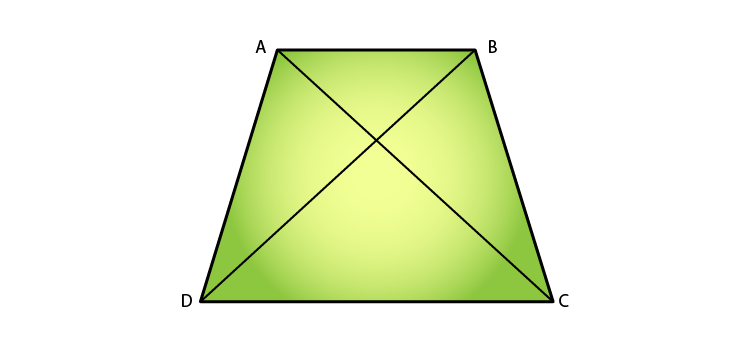We know that,

In ΔAOB and ΔCOD

∠AOB = ∠COD [Vertically Opposite Angles]

∠OAB = ∠OCD    [Alternate angles]

Then, ΔAOB ∼ ΔCOD

Therefore, OA/ OC = OB/ OD   [Corresponding sides are proportional]

10. If Δ ABC and Δ AMP are two right triangles, right angled at B and M, respectively, such that ∠MAP = ∠BAC. Prove that

(i) ΔABC ∼ ΔAMP

(ii) CA/ PA = BC/ MP

Solution:

(i)  Given:

Δ ABC and Δ AMP are the two right triangles.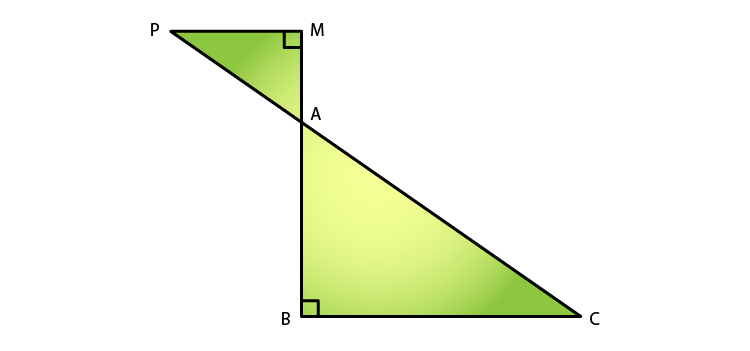We know that,

∠AMP = ∠B = 90o

∠MAP = ∠BAC [Vertically Opposite Angles]

⇒ ΔABC∼ΔAMP     [AA similarity]

(ii) Since, ΔABC∼ΔAMP

CA/ PA = BC/ MP  [Corresponding sides are proportional]

Hence, proved.

11. A vertical stick 10 cm long casts a shadow 8 cm long. At the same time, a tower casts a shadow 30 m long. Determine the height of the tower.

Solution:

Given:

Length of stick = 10cm

Length of the stick’s shadow = 8cm

Length of the tower’s shadow = 30m = 3000cm

Required to find: the height of the tower = PQ.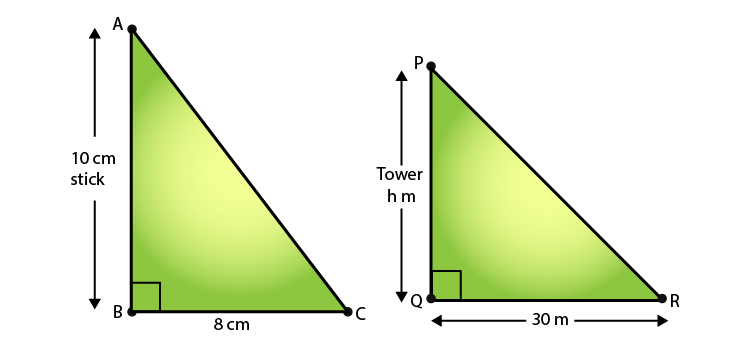In ΔABC ∼ ΔPQR

∠ABC = ∠PQR = 90o

∠ACB = ∠PRQ [Angular Elevation of Sun is same for a particular instant of time]

⇒ ΔABC ∼ ΔPQR    [By AA similarity]

So, we have

AB/BC = PQ/QR [Corresponding sides are proportional]

10/8 = PQ/ 3000

PQ = (3000×10)/ 8

PQ = 30000/8

PQ = 3750/100

Therefore, PQ = 37.5 m

12. In fig.4.143, ∠A = ∠CED, prove that ΔCAB ∼ ΔCED. Also, find the value of x.

Solution:

Given:

∠A = ∠CED

Required to prove: ΔCAB ∼ ΔCED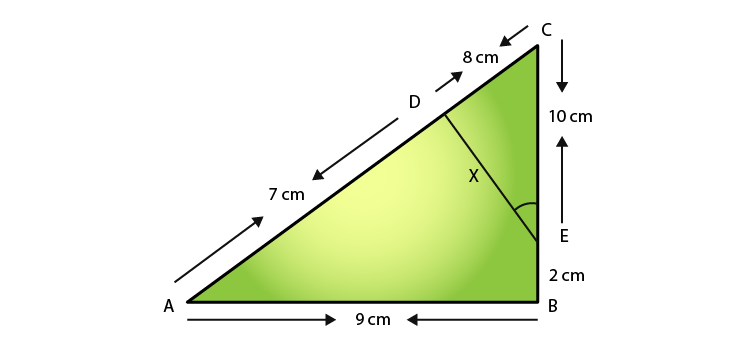In ΔCAB ∼ ΔCED

∠C = ∠C  [Common]

∠A = ∠CED [Given]

⇒ ΔCAB ∼ ΔCED [By AA similarity]

Hence, we have

CA/ CE = AB/ ED  [Corresponding sides are proportional]

15/10 = 9/x

x = (9 x 10)/ 15

Therefore, x = 6 cm

Exercise 4.6 Page No: 4.94

1. Triangles ABC and DEF are similar.

(i) If area of (ΔABC) = 16 cm2, area (ΔDEF) = 25 cmand BC = 2.3 cm, find EF.

(ii) If area (ΔABC) = 9 cm2, area (ΔDEF) = 64 cmand DE = 5.1 cm, find AB.

(iii) If AC = 19 cm and DF = 8 cm, find the ratio of the area of two triangles.

(iv) If area of (ΔABC) = 36 cm2, area (ΔDEF) = 64 cmand DE = 6.2 cm, find AB.

(v) If AB = 1.2 cm and DE = 1.4 cm, find the ratio of the area of two triangles.

Solutions:

As we know, the ratio of areas of two similar triangles is equal to the ratio of squares of their corresponding sides, we get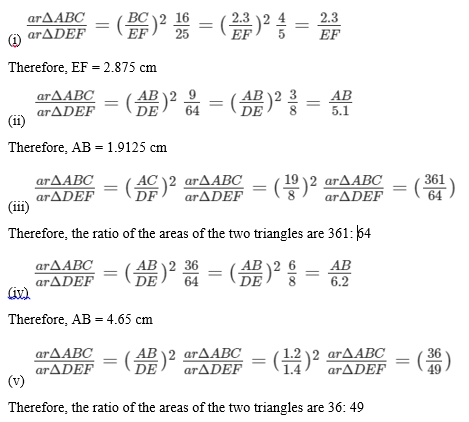2. In the fig 4.178, ΔACB ∼ ΔAPQ. If BC = 10 cm, PQ = 5 cm, BA = 6.5 cm, AP = 2.8 cm, find CA and AQ. Also, find the area (ΔACB): area (ΔAPQ).

Solution:

Given:

ΔACB is similar to ΔAPQ.

BC = 10 cm

PQ = 5 cm

BA = 6.5 cm

AP = 2.8 cm

Required to Find: CA, AQ and that the area (ΔACB): area (ΔAPQ).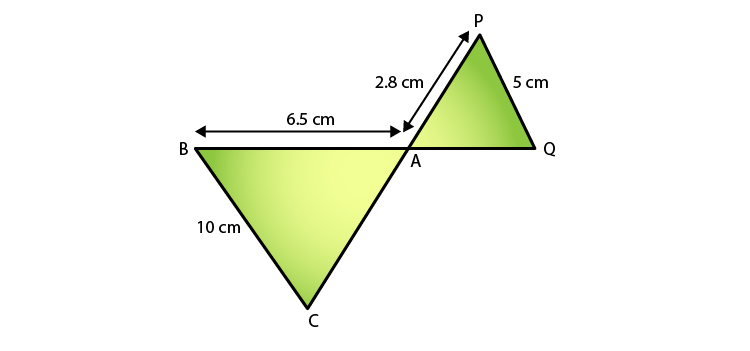Since ΔACB ∼ ΔAPQ,

We know that,

AB/ AQ = BC/ PQ = AC/ AP [Corresponding Parts of Similar Triangles]

AB/ AQ = BC/ PQ

6.5/ AQ = 10/5

⇒ AQ = 3.25 cm

Similarly,

BC/ PQ = CA/ AP

CA/ 2.8 = 10/5

⇒ CA = 5.6 cm

Next,

Since the ratio of areas of two similar triangles is equal to the ratio of squares of their corresponding sides, we have,

ar(ΔACQ): ar(ΔAPQ) = (BC/ PQ)2

= (10/5)2

= (2/1)2

= 4/1

Therefore, the ratio is 4:1.

3. The areas of two similar triangles are 81 cm² and 49 cm², respectively. Find the ration of their corresponding heights. What is the ratio of their corresponding medians?

Solution:

Given: The areas of two similar triangles are 81cm2 and 49cm2.

Required to find: The ratio of their corresponding heights and the ratio of their corresponding medians.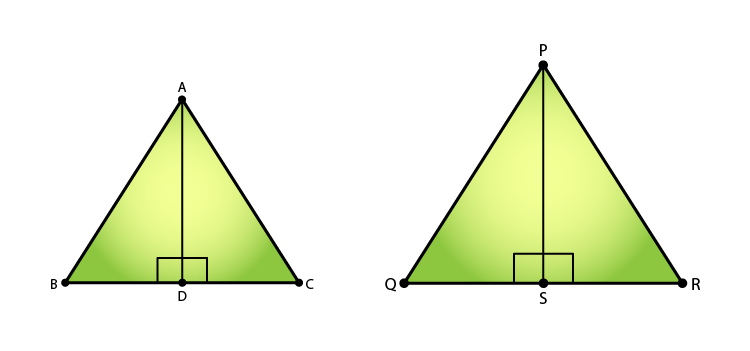Let’s consider the two similar triangles as ΔABC and ΔPQR, AD and PS be the altitudes of ΔABC and ΔPQR, respectively.

So,

By area of similar triangle theorem, we have

ar(ΔABC)/ ar(ΔPQR) = AB2/ PQ2

⇒ 81/ 49 = AB2/ PQ2

⇒ 9/ 7 = AB/ PQ

In ΔABD and ΔPQS

∠B = ∠Q [Since ΔABC ∼ ΔPQR]

∠ABD = ∠PSQ = 90o

⇒ ΔABD ∼ ΔPQS [By AA similarity]

Hence, as the corresponding parts of similar triangles are proportional, we have

Therefore,

AD/ PS = 9/7 (Ratio of altitudes)

Similarly,

The ratio of two similar triangles is equal to the ratio of the squares of their corresponding medians also.

Thus, ratio of altitudes = Ratio of medians = 9/7

4. The areas of two similar triangles are 169 cm2 and 121 cm2, respectively. If the longest side of the larger triangle is 26 cm, find the longest side of the smaller triangle.

Solution:

Given:

The area of two similar triangles is 169cm2 and 121cm2.

The longest side of the larger triangle is 26cm.

Required to find: The longest side of the smaller triangle.

Let the longer side of the smaller triangle = x

We know that the ratio of areas of two similar triangles is equal to the ratio of squares of their corresponding sides, we have,

ar(larger triangle)/ ar(smaller triangle) = (side of the larger triangle/ side of the smaller triangle)2

= 169/ 121

Taking the square roots of LHS and RHS, we get

= 13/ 11

Since sides of similar triangles are propositional, we can say

3/ 11 = (Longer side of the larger triangle)/ (Longer side of the smaller triangle)

⇒ 13/ 11 = 26/ x

x = 22

Therefore, the longest side of the smaller triangle is 22 cm.

5. The areaS of two similar triangles are 25 cm2 and 36 cm2, respectively. If the altitude of the first triangle is 2.4 cm, find the corresponding altitude of the other.

Solution:

Given: The area of two similar triangles are 25 cm2 and 36 cm2, respectively, and the altitude of the first triangle is 2.4 cm.

Required to find: the altitude of the second triangle

We know that the ratio of areas of two similar triangles is equal to the ratio of squares of their corresponding altitudes, we have

⇒ ar(triangle1)/ar(triangle2) = (altitude1/ altitude2)2

⇒ 25/ 36 = (2.4)2/ (altitude2)2

Taking the square roots of LHS and RHS, we get

5/ 6 = 2.4/ altitude2

⇒ altitude2 = (2.4 x 6)/5 = 2.88cm

Therefore, the altitude of the second triangle is 2.88cm.

6. The corresponding altitudes of two similar triangles are 6 cm and 9 cm, respectively. Find the ratio of their areas.

Solution:

Given:

The corresponding altitudes of two similar triangles are 6 cm and 9 cm.

Required to find: Ratio of areas of the two similar triangles.

We know that the ratio of areas of two similar triangles is equal to the ratio of squares of their corresponding altitudes, we have

ar(triangle1)/ar(triangle2) = (altitude1/ altitude2)2 = (6/9)2

= 36/ 81

= 4/9

Therefore, the ratio of the areas of two triangles = 4: 9

7. ABC is a triangle in which ∠ A = 90°, AN ⊥ BC, BC = 12 cm and AC = 5 cm. Find the ratio of the areas of ΔANC and ΔABC.

Solution:

Given:

Given,

ΔABC, ∠A = 90, AN ⊥ BC

BC= 12 cm

AC = 5 cm.

Required to find: ar(ΔANC)/ ar(ΔABC)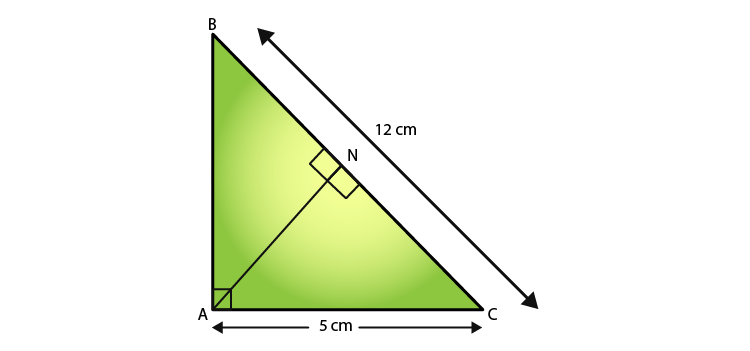We have,

In ΔANC and ΔABC,

∠ACN = ∠ACB  [Common]

∠A = ∠ANC  [each 90]

⇒ ΔANC ∼ ΔABC  [AA similarity]

Since the ratio of areas of two similar triangles is equal to the ratio of squares of their corresponding sides, we get have,

ar(ΔANC)/ ar(ΔABC) = (AC/ BC)2 = (5/12)2 = 25/ 144

Therefore, ar(ΔANC)/ ar(ΔABC) = 25:144

8. In Fig 4.179, DE || BC

(i) If DE = 4m, BC = 6 cm and Area (ΔADE) = 16cm2, find the area of ΔABC.

(ii) If DE = 4cm, BC = 8 cm and Area (ΔADE) = 25cm2, find the area of ΔABC.

(iii) If DE: BC = 3: 5. Calculate the ratio of the areas of ΔADE and the trapezium BCED.

Solution:

Given,

DE ∥ BC.

We know that,

∠DAE = ∠BAC  [Common]

Hence, ΔADE ~ ΔABC (AA Similarity)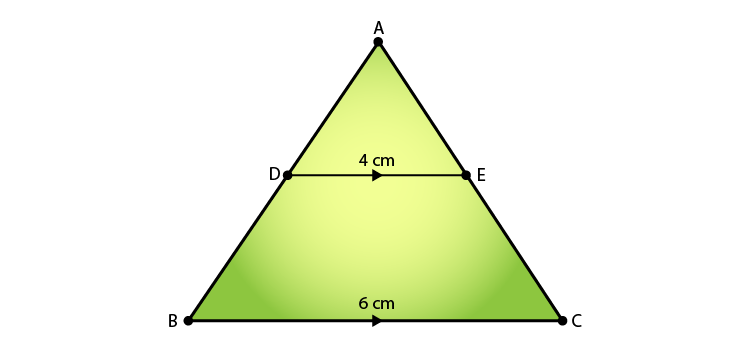(i) Since the ratio of areas of two similar triangles is equal to the ratio of squares of their corresponding sides, we have,

16/ Ar(ΔABC) = 42/ 62

⇒ Ar(ΔABC) = (62 × 16)/ 42

⇒ Ar(ΔABC) = 36 cm2

(ii) Since the ratio of areas of two similar triangles is equal to the ratio of squares of their corresponding sides, we have,

25/ Ar(ΔABC) = 42/ 82

⇒ Ar(ΔABC) = (82 × 25)/ 42

⇒ Ar(ΔABC) = 100 cm2

(iii) According to the question,

Assume that the area of ΔADE = 9x sq units

And, area of ΔABC = 25x sq units

So,

Area of trapezium BCED = Area of ΔABC – Area of ΔADE

= 25x – 9x

= 16x

Now,  Ar(ΔADE)/ Ar(trap BCED) = 9x/ 16x

9. In ΔABC, D and E are the mid-points of AB and AC, respectively. Find the ratio of the areas ΔADE and ΔABC.

Solution:

Given:

In ΔABC, D and E are the midpoints of AB and AC, respectively.

Required to find: Ratio of the areas of ΔADE and ΔABCSince D and E are the midpoints of AB and AC, respectively.

We can say,

DE || BC (By converse of mid-point theorem)

Also, DE = (1/2) BC

∠DAE = ∠BAC  (common)

Thus, ΔADE ~ ΔABC  (AA Similarity)

Now, we know that

The ratio of areas of two similar triangles is equal to the ratio of squares of their corresponding sides, so

Therefore, the ratio of the areas ΔADE and ΔABC is 1:4

10. The areas of two similar triangles are 100 cm2 and 49 cm2, respectively. If the altitude of the bigger triangles is 5 cm, find the corresponding altitude of the other.

Solution:

Given: The area of the two similar triangles is 100cm2 and 49cm2. And the altitude of the bigger triangle is 5cm.

Required to find: The corresponding altitude of the other triangle

We know that,

The ratio of the areas of the two similar triangles is equal to the ratio of squares of their corresponding altitudes.

ar(bigger triangle)/ ar(smaller triangle) = (altitude of the bigger triangle/ altitude of the smaller triangle)2

(100/ 49) = (5/ altitude of the smaller triangle)2

Taking square root on LHS and RHS, we get

(10/ 7) = (5/ altitude of the smaller triangle) = 7/2

Therefore, the altitude of the smaller triangle = 3.5cm

11. The areas of two similar triangles are 121 cm2 and 64 cm2, respectively. If the median of the first triangle is 12.1 cm, find the corresponding median of the other.

Solution:

Given: the area of the two triangles is 121cm2 and 64cm2, respectively, and the median of the first triangle is 12.1cm.

Required to find: The corresponding median of the other triangle.

We know that,

The ratio of the areas of the two similar triangles is equal to the ratio of the squares of their medians.

ar(triangle1)/ ar(triangle2) = (median of triangle 1/median of triangle 2)2

121/ 64 = (12.1/ median of triangle 2)2

Taking the square roots on both LHS and RHS, we have

11/8 = (12.1/ median of triangle 2) = (12.1 x 8)/ 11

Therefore, the median of the other triangle = 8.8cm

Exercise 4.7 Page No: 4.119

1. If the sides of a triangle are 3 cm, 4 cm, and 6 cm long, determine whether the triangle is a right-angled triangle.

Solution:

We have,

Sides of the triangle as

AB = 3 cm

BC = 4 cm

AC = 6 cm

On finding their squares, we get

AB2 = 32 = 9

BC= 42 = 16

AC2 = 62 = 36

Since, AB2 + BC2 ≠ AC2

So, by the converse of Pythagoras’ theorem, the given sides cannot be the sides of a right triangle.

2. The sides of certain triangles are given below. Determine which of them are right triangles.

(i) a = 7 cm, b = 24 cm and c = 25 cm

(ii) a = 9 cm, b = 16 cm and c = 18 cm

(iii) a = 1.6 cm, b = 3.8 cm and c = 4 cm

(iv) a = 8 cm, b = 10 cm and c = 6 cm

Solutions:

(i) Given,

a = 7 cm, b = 24 cm and c = 25 cm

∴ a2 = 49, b2 = 576 and c2 = 625

Since, a2 + b2 = 49 + 576 = 625 = c2

Then, by the converse of Pythagoras’ theorem,

The given sides are of a right triangle.

(ii) Given,

a = 9 cm, b = 16 cm and c = 18 cm

∴ a2 = 81, b2 = 256 and c2 = 324

Since, a2 + b2 = 81 + 256 = 337 ≠ c2

Then, by the converse of Pythagoras’ theorem,

The given sides cannot be of a right triangle.

(iii) Given,

a = 1.6 cm, b = 3.8 cm and C = 4 cm

∴ a2 = 2.56, b2 = 14.44 and c2 = 16

Since, a2 + b2 = 2.56 + 14.44 = 17 ≠ c2

Then, by the converse of Pythagoras’ theorem,

The given sides cannot be of a right triangle.

(iv) Given,

a = 8 cm, b = 10 cm and C = 6 cm

∴ a2 = 64, b2 = 100 and c2 = 36

Since, a2 + c2 = 64 + 36 = 100 = b2

Then, by the converse of Pythagoras’ theorem,

The given sides are of a right triangle

3. A man goes 15 metres due west and then 8 metres due north. How far is he from the starting point?

Solution:

Let the starting point of the man be O and the final point be A.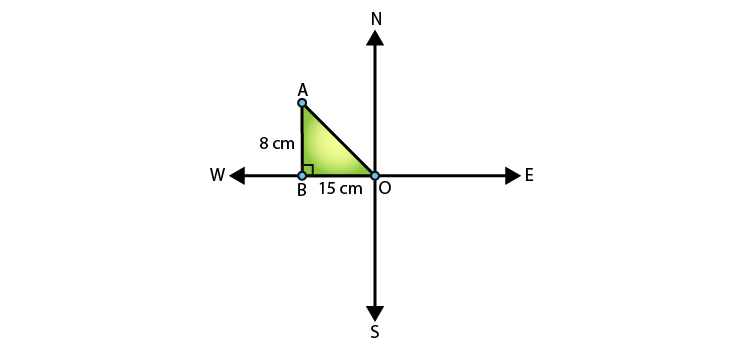In ∆ABO,

by Pythagoras theorem AO2 = AB2 + BO2

⇒ AO2 = 82 + 152

⇒ AO2 = 64 + 225 = 289

⇒ AO =  √289 = 17m

∴ the man is 17m far from the starting point.

4. A ladder 17 m long reaches a window of a building 15 m above the ground. Find the distance of the foot of the ladder from the building.

Solution: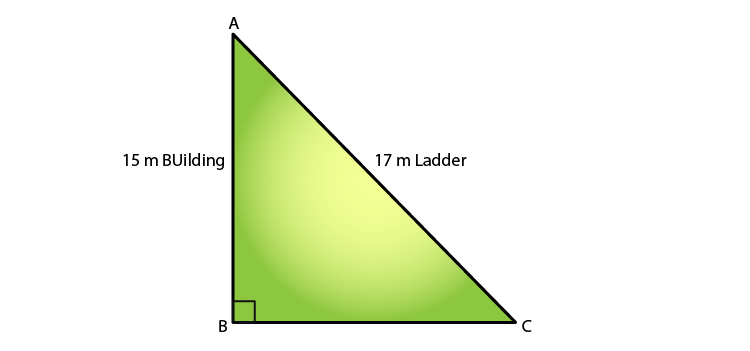In ∆ABC, by using Pythagoras’ theorem,

AB2 + BC2 = AC2

⇒ 152 + BC2 = 172

225 + BC2 = 172

BC2 = 289 – 225

BC2 = 64

∴ BC = 8 m

Therefore, the distance between the foot of the ladder from the building = 8 m

5. Two poles of heights 6 m and 11 m stand on a plane ground. If the distance between their feet is 12 m, find the distance between their tops.

Solution:

Let CD and AB be the poles of height 11m and 6m.

Then, it’s seen that CP = 11 – 6 = 5m.

From the figure, AP should be 12m (given).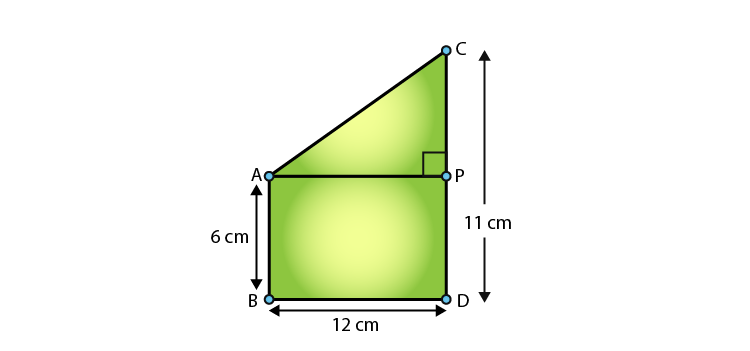In triangle APC, by applying Pythagoras’ theorem, we have

AP2 + PC2 = AC2

122 + 52 = AC2

AC2 = 144 + 25 = 169

∴ AC = 13 (by taking sq. root on both sides)

Thus, the distance between their tops = 13 m

6. In an isosceles triangle ABC, AB = AC = 25 cm, BC = 14 cm. Calculate the altitude from A to BC.

Solution:

Given,

∆ABC, AB = AC = 25 cm and BC = 14.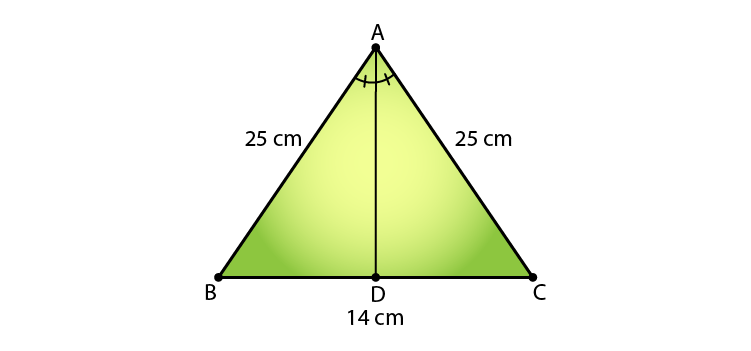In ∆ABD and ∆ACD, we see that

AB = AC                                [Given]

Then, ∆ABD ≅ ∆ACD      [By RHS condition]

Thus, BD = CD = 7 cm              [By corresponding parts of congruent triangles]

Finally,

⇒ AD2 + 72 = 252

AD2 = 625 – 49 = 576

∴ AD = √576 = 24 cm

7. The foot of a ladder is 6 m away from a wall, and its top reaches a window 8 m above the ground. If the ladder is shifted in such a way that its foot is 8 m away from the wall, to what height does its tip reach?

Solution:

Let’s assume the length of the ladder to be AD = BE = x m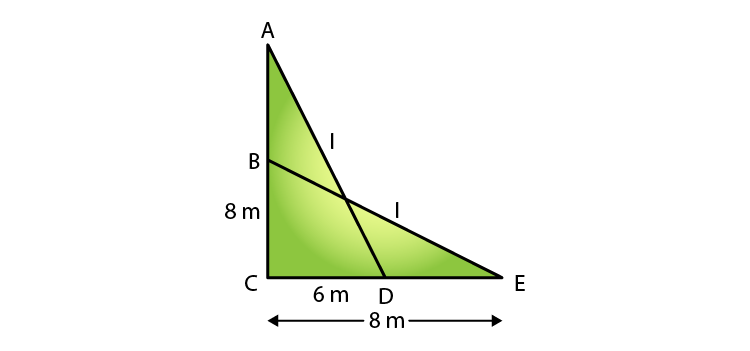So, in ∆ACD, by Pythagoras’ theorem,

We have,

⇒ x2 = 82 + 62 … (i)

Also, in ∆BCE, by using Pythagoras’ theorem,

BE2 = BC2 + CE2

⇒ x2 = BC2 + 82 … (ii)

Compare (i) and (ii).

BC2 + 82 = 82 + 62

⇒ BC2 + 62

⇒ BC = 6 m

Therefore, the tip of the ladder reaches a height od 6m.

8. Two poles of height 9 in and 14 m stand on a plane ground. If the distance between their feet is 12 m, find the distance between their tops.

Solution: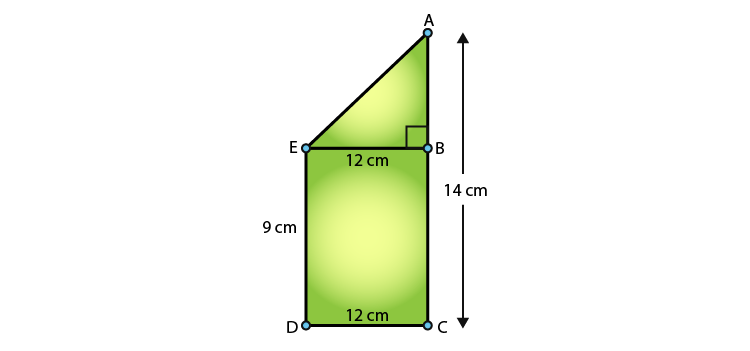Comparing the figure, and it’s given that

AC = 14 m, DC = 12m and ED = BC = 9 m

Construction: Draw EB ⊥ AC

Now,

It’s seen that AB = AC – BC = (14 – 9) = 5 m

And EB = DC = 12m [distance between their feet]

Thus,

In ∆ABE, by Pythagoras’ theorem, we have

AE2 = AB2 + BE2

AE2 = 52 + 122

AE2 = 25 + 144 = 169

⇒ AE = √169 = 13 m

Therefore, the distance between their tops = 13 m

9. Using Pythagoras’ theorem, determine the length of AD in terms of b and c shown in Fig. 4.219

Solution: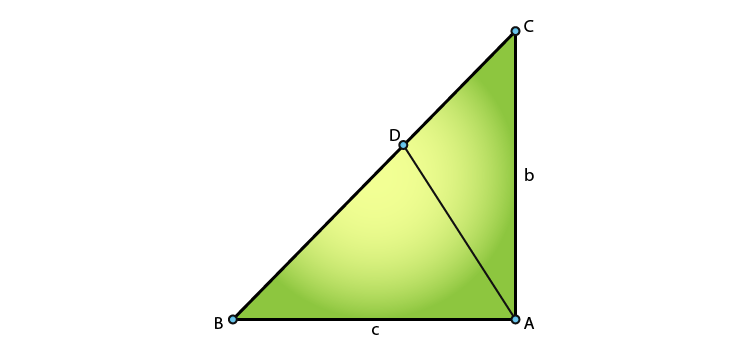We have,

In ∆BAC, by Pythagoras’ theorem, we have

BC2 = AB2 + AC2

⇒ BC2 = c2 + b2

⇒ BC = √(c2 + b2)

In ∆ABD and ∆CBA,

∠B = ∠B                      [Common]

Then, ∆ABD ͏~ ∆CBA   [By AA similarity]

Thus,

AB/ CB = AD/ CA [Corresponding parts of similar triangles are proportional]

c/ √(c2 + b2) = AD/ b

∴ AD = bc/ √(c2 + b2)

10. A triangle has sides 5 cm, 12 cm and 13 cm. Find the length, to one decimal place, of the perpendicular from the opposite vertex to the side whose length is 13 cm.

Solution:

From the fig. AB = 5cm, BC = 12 cm and AC = 13 cm

Then, AC2 = AB2 + BC2.

⇒ (13)2 = (5)2 + (12)2 = 25 + 144 = 169 = 132

This proves that ∆ABC is a right triangle, right angled at B.

Let BD be the length of the perpendicular from B on AC.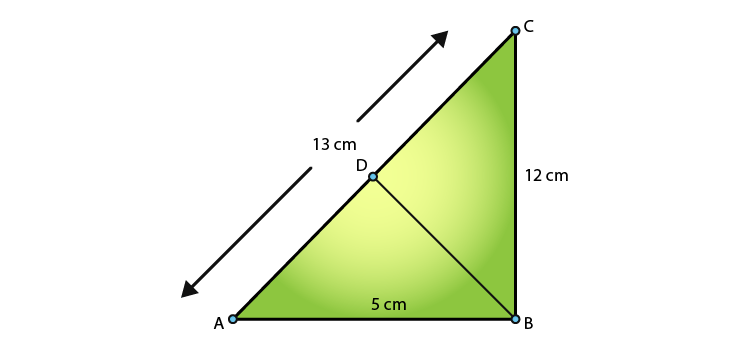So, the area of ∆ABC = (BC x BA)/ 2 [Taking BC as the altitude]

= (12 x 5)/ 2

= 30 cm2

Also, the area of ∆ABC = (AC x BD)/ 2 [Taking BD as the altitude]

= (13 x BD)/ 2

⇒ (13 x BD)/ 2 = 30

BD = 60/13 = 4.6 (to one decimal place)

11. ABCD is a square. F is the mid-point of AB. BE is one-third of BC. If the area of ∆ FBE = 108cm2, find the length of AC.

Solution:

Given,

ABCD is a square. And F is the mid-point of AB.

BE is one-third of BC.

Area of ∆ FBE = 108cm2

Required to find: length of AC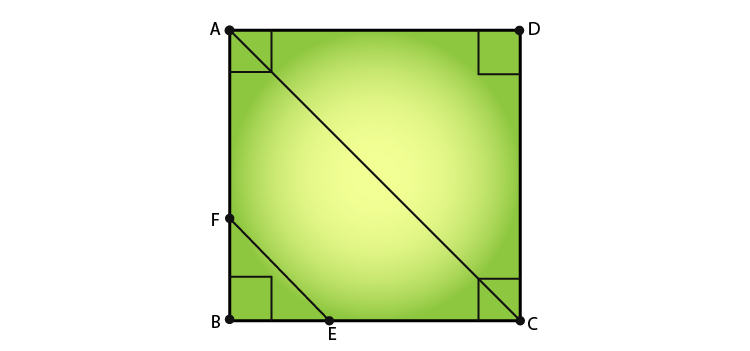Let’s assume the sides of the square to be x.

⇒ AB = BC = CD = DA = x cm

And, AF = FB = x/2 cm

So, BE = x/3 cm

Now, the area of ∆ FBE = 1/2 x BE x FB

⇒ 108 = (1/2) x (x/3) x (x/2)

⇒ x2 = 108 x 2 x 3 x 2 = 1296

⇒ x = √(1296) [taking square roots of both sides]

∴ x = 36cm

Further in ∆ ABC, by Pythagoras’ theorem, we have

AC2 = AB2 + BC2

⇒ AC2 = x2 + x2 = 2x2

⇒ AC2 = 2 x (36)2

⇒ AC = 36√2 = 36 x 1.414 = 50.904 cm

Therefore, the length of AC is 50.904 cm.

12. In an isosceles triangle ABC, if AB = AC = 13cm and the altitude from A on BC is 5cm, find BC.

Solution:

Given,

An isosceles triangle ABC, AB = AC = 13cm, AD = 5cm

Required to find: BC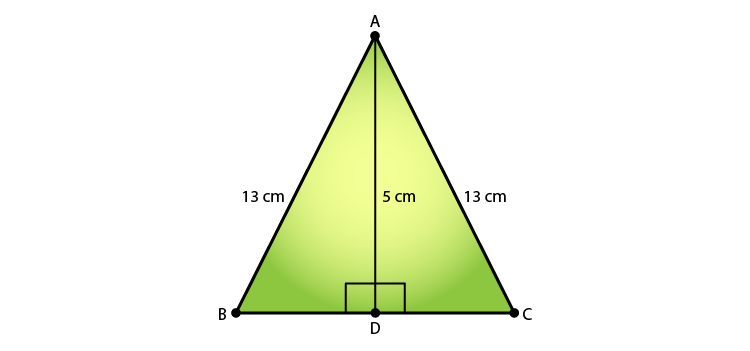In ∆ ADB, by using Pythagoras’ theorem, we have

52 + BD2 = 169

BD2 = 169 – 25 = 144

⇒BD = √144 = 12 cm

Similarly, applying Pythagoras’ theorem is ∆ ADC we can have,

132 = 52 + DC2

⇒ DC = √144 = 12 cm

Thus, BC = BD + DC = 12 + 12 = 24 cm

13. In a ∆ABC, AB = BC = CA = 2a and AD ⊥ BC. Prove that

(i) AD = a√3 (ii) Area (∆ABC) = √3 a2

Solution: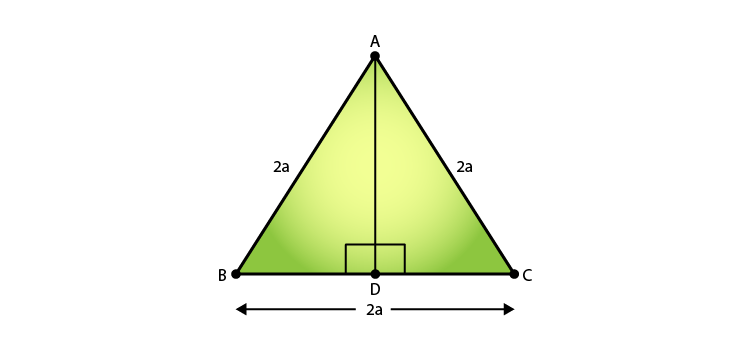(i) In ∆ABD and ∆ACD, we have

AB = AC     [Given]

So, ∆ABD ≅ ∆ACD   [By RHS condition]

Hence, BD = CD = a [By C.P.C.T]

Now, in ∆ABD, by using Pythagoras’ theorem,

AD2 = 4a2 – a2 = 3a2

(ii) Area (∆ABC) = 1/2 x BC x AD

= 1/2 x (2a) x (a√3)

= √3 a2

14. The lengths of the diagonals of a rhombus are 24cm and 10cm. Find each side of the rhombus.

Solution:

Let ABCD be a rhombus and AC and BD be the diagonals of ABCD.

So, AC = 24cm and BD = 10cm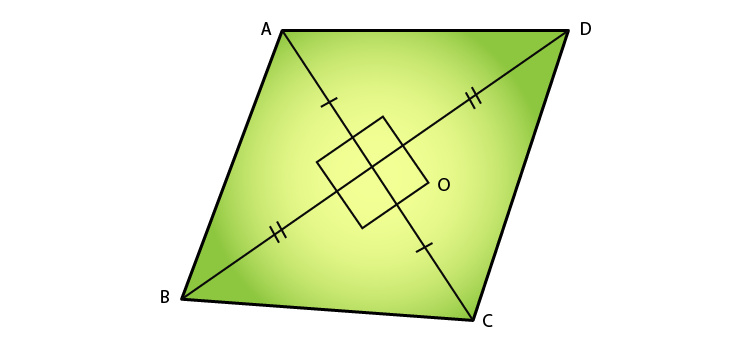We know that diagonals of a rhombus bisect each other at the right angle. (Perpendicular to each other)

So,

AO = OC = 12cm and BO = OD = 3cm

In ∆AOB, by Pythagoras’ theorem, we have

AB2 = AO2 + BO2

= 122 + 52

= 144 + 25

= 169

⇒ AB = √169 = 13cm

The sides of the rhombus are all equal.

Therefore, AB = BC = CD = AD = 13cm.

## Frequently Asked Questions on RD Sharma Solutions for Class 10 Maths Chapter 4

### Is it necessary to learn all the topics provided in RD Sharma Solutions for Class 10 Maths Chapter 4?

Yes, it is compulsory to learn all the topics provided in RD Sharma Solutions for Class 10 Maths Chapter 4 to score high marks in Class 10 board exams. These solutions are designed by subject matter experts after conducting vast research on each topic. They also focus on cracking the Solutions of Maths in such a way that it is easy for the students to understand.

### Are RD Sharma Solutions for Class 10 Maths Chapter 4 enough to score high marks in the board exam?

Yes, it is enough to solve all the questions of RD Sharma Solutions for Class 10 Maths Chapter 4 to excel in the board exam. Practising this chapter can make them learn the concepts flawlessly. The answers have been developed as per the RD Sharma syllabus and the guidelines. This allows the students to score good marks in the finals, and students will be able to grasp the concepts perfectly. It also helps in boosting their confidence, which plays a pivotal role in the examinations.

### Mention the topics covered in RD Sharma Solutions for Class 10 Maths Chapter 4.

The main topics covered in RD Sharma Solutions for Class 10 Maths Chapter 4 are:
1. Similar polygons
2. Similar triangles and their properties
3. Basic results on proportionality
4. Basic Proportionality Theorem
5. Criteria for the similarity of triangles
6. Areas of two similar triangles